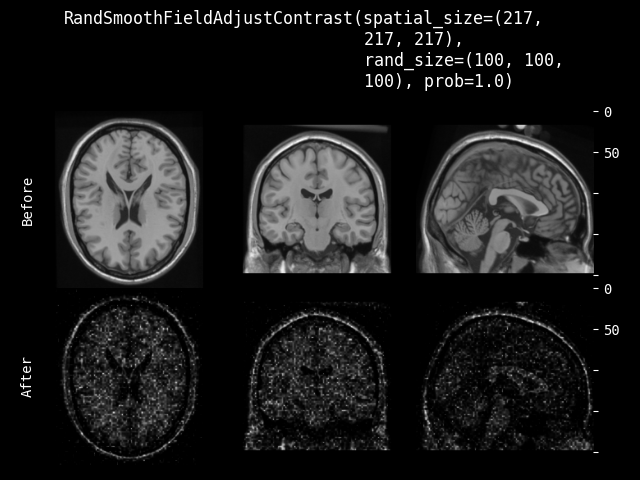# Transforms#

## Generic Interfaces#

### Transform#

class monai.transforms.Transform[source]#

An abstract class of a `Transform`. A transform is callable that processes `data`.

It could be stateful and may modify `data` in place, the implementation should be aware of:

1. thread safety when mutating its own states. When used from a multi-process context, transform’s instance variables are read-only. thread-unsafe transforms should inherit `monai.transforms.ThreadUnsafe`.

2. `data` content unused by this transform may still be used in the subsequent transforms in a composed transform.

3. storing too much information in `data` may cause some memory issue or IPC sync issue, especially in the multi-processing environment of PyTorch DataLoader.

abstract __call__(data)[source]#

`data` is an element which often comes from an iteration over an iterable, such as `torch.utils.data.Dataset`. This method should return an updated version of `data`. To simplify the input validations, most of the transforms assume that

• `data` is a Numpy ndarray, PyTorch Tensor or string,

• the data shape can be:

1. string data without shape, LoadImage transform expects file paths,

2. most of the pre-/post-processing transforms expect: `(num_channels, spatial_dim_1[, spatial_dim_2, ...])`, except for example: AddChannel expects (spatial_dim_1[, spatial_dim_2, …])

• the channel dimension is often not omitted even if number of channels is one.

This method can optionally take additional arguments to help execute transformation operation.

Raises:

NotImplementedError – When the subclass does not override this method.

### MapTransform#

class monai.transforms.MapTransform(keys, allow_missing_keys=False)[source]#

A subclass of `monai.transforms.Transform` with an assumption that the `data` input of `self.__call__` is a MutableMapping such as `dict`.

The `keys` parameter will be used to get and set the actual data item to transform. That is, the callable of this transform should follow the pattern:

```def __call__(self, data):
for key in self.keys:
if key in data:
# update output data with some_transform_function(data[key]).
else:
# raise exception unless allow_missing_keys==True.
return data
```
Raises:
• ValueError – When `keys` is an empty iterable.

• TypeError – When `keys` type is not in `Union[Hashable, Iterable[Hashable]]`.

abstract __call__(data)[source]#

`data` often comes from an iteration over an iterable, such as `torch.utils.data.Dataset`.

To simplify the input validations, this method assumes:

• `data` is a Python dictionary,

• `data[key]` is a Numpy ndarray, PyTorch Tensor or string, where `key` is an element of `self.keys`, the data shape can be:

1. string data without shape, LoadImaged transform expects file paths,

2. most of the pre-/post-processing transforms expect: `(num_channels, spatial_dim_1[, spatial_dim_2, ...])`, except for example: AddChanneld expects (spatial_dim_1[, spatial_dim_2, …])

• the channel dimension is often not omitted even if number of channels is one.

Raises:

NotImplementedError – When the subclass does not override this method.

Returns:

An updated dictionary version of `data` by applying the transform.

call_update(data)[source]#

This function is to be called after every self.__call__(data), update data[key_transforms] and data[key_meta_dict] using the content from MetaTensor data[key], for MetaTensor backward compatibility 0.9.0.

first_key(data)[source]#

Get the first available key of self.keys in the input data dictionary. If no available key, return an empty tuple ().

Parameters:

data (`dict`[`Hashable`, `Any`]) – data that the transform will be applied to.

key_iterator(data, *extra_iterables)[source]#

Iterate across keys and optionally extra iterables. If key is missing, exception is raised if allow_missing_keys==False (default). If allow_missing_keys==True, key is skipped.

Parameters:
• data – data that the transform will be applied to

• extra_iterables – anything else to be iterated through

### RandomizableTrait#

class monai.transforms.RandomizableTrait[source]#

An interface to indicate that the transform has the capability to perform randomized transforms to the data that it is called upon. This interface can be extended from by people adapting transforms to the MONAI framework as well as by implementors of MONAI transforms.

### LazyTrait#

class monai.transforms.LazyTrait[source]#

An interface to indicate that the transform has the capability to execute using MONAI’s lazy resampling feature. In order to do this, the implementing class needs to be able to describe its operation as an affine matrix or grid with accompanying metadata. This interface can be extended from by people adapting transforms to the MONAI framework as well as by implementors of MONAI transforms.

property lazy#

Get whether lazy evaluation is enabled for this transform instance. :returns: True if the transform is operating in a lazy fashion, False if not.

property requires_current_data#

Get whether the transform requires the input data to be up to date before the transform executes. Such transforms can still execute lazily by adding pending operations to the output tensors. :returns: True if the transform requires its inputs to be up to date and False if it does not

### MultiSampleTrait#

class monai.transforms.MultiSampleTrait[source]#

An interface to indicate that the transform has the capability to return multiple samples given an input, such as when performing random crops of a sample. This interface can be extended from by people adapting transforms to the MONAI framework as well as by implementors of MONAI transforms.

### Randomizable#

class monai.transforms.Randomizable[source]#

An interface for handling random state locally, currently based on a class variable R, which is an instance of np.random.RandomState. This provides the flexibility of component-specific determinism without affecting the global states. It is recommended to use this API with `monai.data.DataLoader` for deterministic behaviour of the preprocessing pipelines. This API is not thread-safe. Additionally, deepcopying instance of this class often causes insufficient randomness as the random states will be duplicated.

randomize(data)[source]#

Within this method, `self.R` should be used, instead of np.random, to introduce random factors.

all `self.R` calls happen here so that we have a better chance to identify errors of sync the random state.

This method can generate the random factors based on properties of the input data.

Raises:

NotImplementedError – When the subclass does not override this method.

Return type:

`None`

set_random_state(seed=None, state=None)[source]#

Set the random state locally, to control the randomness, the derived classes should use `self.R` instead of np.random to introduce random factors.

Parameters:
• seed – set the random state with an integer seed.

• state – set the random state with a np.random.RandomState object.

Raises:

TypeError – When `state` is not an `Optional[np.random.RandomState]`.

Returns:

a Randomizable instance.

### LazyTransform#

class monai.transforms.LazyTransform(lazy=False)[source]#

An implementation of functionality for lazy transforms that can be subclassed by array and dictionary transforms to simplify implementation of new lazy transforms.

property lazy#

Get whether lazy evaluation is enabled for this transform instance. :returns: True if the transform is operating in a lazy fashion, False if not.

property requires_current_data#

Get whether the transform requires the input data to be up to date before the transform executes. Such transforms can still execute lazily by adding pending operations to the output tensors. :returns: True if the transform requires its inputs to be up to date and False if it does not

### RandomizableTransform#

class monai.transforms.RandomizableTransform(prob=1.0, do_transform=True)[source]#

An interface for handling random state locally, currently based on a class variable R, which is an instance of np.random.RandomState. This class introduces a randomized flag _do_transform, is mainly for randomized data augmentation transforms. For example:

```from monai.transforms import RandomizableTransform

class RandShiftIntensity100(RandomizableTransform):
def randomize(self):
super().randomize(None)
self._offset = self.R.uniform(low=0, high=100)

def __call__(self, img):
self.randomize()
if not self._do_transform:
return img
return img + self._offset

transform = RandShiftIntensity()
transform.set_random_state(seed=0)
print(transform(10))
```
randomize(data)[source]#

Within this method, `self.R` should be used, instead of np.random, to introduce random factors.

all `self.R` calls happen here so that we have a better chance to identify errors of sync the random state.

This method can generate the random factors based on properties of the input data.

Return type:

`None`

### Compose#

class monai.transforms.Compose(transforms=None, map_items=True, unpack_items=False, log_stats=False, lazy=False, overrides=None)[source]#

`Compose` provides the ability to chain a series of callables together in a sequential manner. Each transform in the sequence must take a single argument and return a single value.

`Compose` can be used in two ways:

1. With a series of transforms that accept and return a single ndarray / tensor / tensor-like parameter.

2. With a series of transforms that accept and return a dictionary that contains one or more parameters. Such transforms must have pass-through semantics that unused values in the dictionary must be copied to the return dictionary. It is required that the dictionary is copied between input and output of each transform.

If some transform takes a data item dictionary as input, and returns a sequence of data items in the transform chain, all following transforms will be applied to each item of this list if map_items is True (the default). If map_items is False, the returned sequence is passed whole to the next callable in the chain.

For example:

A Compose([transformA, transformB, transformC], map_items=True)(data_dict) could achieve the following patch-based transformation on the data_dict input:

1. transformA normalizes the intensity of ‘img’ field in the data_dict.

2. transformB crops out image patches from the ‘img’ and ‘seg’ of data_dict, and return a list of three patch samples:

```{'img': 3x100x100 data, 'seg': 1x100x100 data, 'shape': (100, 100)}
applying transformB
---------->
[{'img': 3x20x20 data, 'seg': 1x20x20 data, 'shape': (20, 20)},
{'img': 3x20x20 data, 'seg': 1x20x20 data, 'shape': (20, 20)},
{'img': 3x20x20 data, 'seg': 1x20x20 data, 'shape': (20, 20)},]
```
3. transformC then randomly rotates or flips ‘img’ and ‘seg’ of each dictionary item in the list returned by transformB.

The composed transforms will be set the same global random seed if user called set_determinism().

When using the pass-through dictionary operation, you can make use of `monai.transforms.adaptors.adaptor` to wrap transforms that don’t conform to the requirements. This approach allows you to use transforms from otherwise incompatible libraries with minimal additional work.

Note

In many cases, Compose is not the best way to create pre-processing pipelines. Pre-processing is often not a strictly sequential series of operations, and much of the complexity arises when a not-sequential set of functions must be called as if it were a sequence.

Example: images and labels Images typically require some kind of normalization that labels do not. Both are then typically augmented through the use of random rotations, flips, and deformations. Compose can be used with a series of transforms that take a dictionary that contains ‘image’ and ‘label’ entries. This might require wrapping torchvision transforms before passing them to compose. Alternatively, one can create a class with a __call__ function that calls your pre-processing functions taking into account that not all of them are called on the labels.

Lazy resampling:

Lazy resampling is an experimental feature introduced in 1.2. Its purpose is to reduce the number of resample operations that must be carried out when executing a pipeline of transforms. This can provide significant performance improvements in terms of pipeline executing speed and memory usage, and can also significantly reduce the loss of information that occurs when performing a number of spatial resamples in succession.

Lazy resampling can be enabled or disabled through the `lazy` parameter, either by specifying it at initialisation time or overriding it at call time.

• False (default): Don’t perform any lazy resampling

• None: Perform lazy resampling based on the ‘lazy’ properties of the transform instances.

• True: Always perform lazy resampling if possible. This will ignore the `lazy` properties of the transform instances

Please see the Lazy Resampling topic for more details of this feature and examples of its use.

Parameters:
• transforms – sequence of callables.

• map_items – whether to apply transform to each item in the input data if data is a list or tuple. defaults to True.

• unpack_items – whether to unpack input data with * as parameters for the callable function of transform. defaults to False.

• log_stats – this optional parameter allows you to specify a logger by name for logging of pipeline execution. Setting this to False disables logging. Setting it to True enables logging to the default loggers. Setting a string overrides the logger name to which logging is performed.

• lazy – whether to enable Lazy Resampling for lazy transforms. If False, transforms will be carried out on a transform by transform basis. If True, all lazy transforms will be executed by accumulating changes and resampling as few times as possible. If lazy is None, Compose will perform lazy execution on lazy transforms that have their lazy property set to True.

• overrides – this optional parameter allows you to specify a dictionary of parameters that should be overridden when executing a pipeline. These each parameter that is compatible with a given transform is then applied to that transform before it is executed. Note that overrides are currently only applied when Lazy Resampling is enabled for the pipeline or a given transform. If lazy is False they are ignored. Currently supported args are: {`"mode"`, `"padding_mode"`, `"dtype"`, `"align_corners"`, `"resample_mode"`, `device`}.

Call self as a function.

flatten()[source]#

Return a Composition with a simple list of transforms, as opposed to any nested Compositions.

e.g., t1 = Compose([x, x, x, x, Compose([Compose([x, x]), x, x])]).flatten() will result in the equivalent of t1 = Compose([x, x, x, x, x, x, x, x]).

get_index_of_first(predicate)[source]#

get_index_of_first takes a `predicate` and returns the index of the first transform that satisfies the predicate (ie. makes the predicate return True). If it is unable to find a transform that satisfies the `predicate`, it returns None.

Example

c = Compose([Flip(…), Rotate90(…), Zoom(…), RandRotate(…), Resize(…)])

print(c.get_index_of_first(lambda t: isinstance(t, RandomTrait))) >>> 3 print(c.get_index_of_first(lambda t: isinstance(t, Compose))) >>> None

Note

This is only performed on the transforms directly held by this instance. If this instance has nested `Compose` transforms or other transforms that contain transforms, it does not iterate into them.

Parameters:
• predicate – a callable that takes a single argument and returns a bool. When called

• compose (it is passed a transform from the sequence of transforms contained by this) –

• instance.

Returns:

The index of the first transform in the sequence for which `predicate` returns True. None if no transform satisfies the `predicate`

inverse(data)[source]#

Inverse of `__call__`.

Raises:

NotImplementedError – When the subclass does not override this method.

property lazy#

Get whether lazy evaluation is enabled for this transform instance. :returns: True if the transform is operating in a lazy fashion, False if not.

randomize(data=None)[source]#

Within this method, `self.R` should be used, instead of np.random, to introduce random factors.

all `self.R` calls happen here so that we have a better chance to identify errors of sync the random state.

This method can generate the random factors based on properties of the input data.

Raises:

NotImplementedError – When the subclass does not override this method.

set_random_state(seed=None, state=None)[source]#

Set the random state locally, to control the randomness, the derived classes should use `self.R` instead of np.random to introduce random factors.

Parameters:
• seed – set the random state with an integer seed.

• state – set the random state with a np.random.RandomState object.

Raises:

TypeError – When `state` is not an `Optional[np.random.RandomState]`.

Returns:

a Randomizable instance.

### InvertibleTransform#

class monai.transforms.InvertibleTransform[source]#

Classes for invertible transforms.

This class exists so that an `invert` method can be implemented. This allows, for example, images to be cropped, rotated, padded, etc., during training and inference, and after be returned to their original size before saving to file for comparison in an external viewer.

When the `inverse` method is called:

• the inverse is called on each key individually, which allows for different parameters being passed to each label (e.g., different interpolation for image and label).

• the inverse transforms are applied in a last-in-first-out order. As the inverse is applied, its entry is removed from the list detailing the applied transformations. That is to say that during the forward pass, the list of applied transforms grows, and then during the inverse it shrinks back down to an empty list.

We currently check that the `id()` of the transform is the same in the forward and inverse directions. This is a useful check to ensure that the inverses are being processed in the correct order.

Note to developers: When converting a transform to an invertible transform, you need to:

1. Inherit from this class.

2. In `__call__`, add a call to `push_transform`.

3. Any extra information that might be needed for the inverse can be included with the dictionary `extra_info`. This dictionary should have the same keys regardless of whether `do_transform` was True or False and can only contain objects that are accepted in pytorch data loader’s collate function (e.g., None is not allowed).

4. Implement an `inverse` method. Make sure that after performing the inverse, `pop_transform` is called.

inverse(data)[source]#

Inverse of `__call__`.

Raises:

NotImplementedError – When the subclass does not override this method.

Return type:

`Any`

inverse_update(data)[source]#

This function is to be called before every self.inverse(data), update each MetaTensor data[key] using data[key_transforms] and data[key_meta_dict], for MetaTensor backward compatibility 0.9.0.

### TraceableTransform#

class monai.transforms.TraceableTransform[source]#

Maintains a stack of applied transforms to data.

Data can be one of two types:
1. A MetaTensor (this is the preferred data type).

2. A dictionary of data containing arrays/tensors and auxiliary metadata. In

this case, a key must be supplied (this dictionary-based approach is deprecated).

If data is of type MetaTensor, then the applied transform will be added to `data.applied_operations`.

If data is a dictionary, then one of two things can happen:
1. If data[key] is a MetaTensor, the applied transform will be added to `data[key].applied_operations`.

2. Else, the applied transform will be appended to an adjacent list using

trace_key. If, for example, the key is image, then the transform will be appended to image_transforms (this dictionary-based approach is deprecated).

Hopefully it is clear that there are three total possibilities:
1. data is MetaTensor

2. data is dictionary, data[key] is MetaTensor

3. data is dictionary, data[key] is not MetaTensor (this is a deprecated approach).

The `__call__` method of this transform class must be implemented so that the transformation information is stored during the data transformation.

The information in the stack of applied transforms must be compatible with the default collate, by only storing strings, numbers and arrays.

tracing could be enabled by self.set_tracing or setting MONAI_TRACE_TRANSFORM when initializing the class.

check_transforms_match(transform)[source]#

Check transforms are of same instance.

Return type:

`None`

get_most_recent_transform(data, key=None, check=True, pop=False)[source]#

Get most recent transform for the stack.

Parameters:
• data – dictionary of data or MetaTensor.

• key (`Optional`[`Hashable`]) – if data is a dictionary, data[key] will be modified.

• check (`bool`) – if true, check that self is the same type as the most recently-applied transform.

• pop (`bool`) – if true, remove the transform as it is returned.

Returns:

Dictionary of most recently applied transform

Raises:

- RuntimeError – data is neither MetaTensor nor dictionary

get_transform_info()[source]#

Return a dictionary with the relevant information pertaining to an applied transform.

Return type:

`dict`

pop_transform(data, key=None, check=True)[source]#

Return and pop the most recent transform.

Parameters:
• data – dictionary of data or MetaTensor

• key (`Optional`[`Hashable`]) – if data is a dictionary, data[key] will be modified

• check (`bool`) – if true, check that self is the same type as the most recently-applied transform.

Returns:

Dictionary of most recently applied transform

Raises:

- RuntimeError – data is neither MetaTensor nor dictionary

push_transform(data, *args, **kwargs)[source]#

Push to a stack of applied transforms of `data`.

Parameters:
• data – dictionary of data or MetaTensor.

• args – additional positional arguments to track_transform_meta.

• kwargs – additional keyword arguments to track_transform_meta, set `replace=True` (default False) to rewrite the last transform infor in applied_operation/pending_operation based on `self.get_transform_info()`.

set_tracing(tracing)[source]#

Set whether to trace transforms.

Return type:

`None`

static trace_key(key=None)[source]#

The key to store the stack of applied transforms.

trace_transform(to_trace)[source]#

Temporarily set the tracing status of a transform with a context manager.

classmethod track_transform_meta(data, key=None, sp_size=None, affine=None, extra_info=None, orig_size=None, transform_info=None, lazy=False)[source]#

Update a stack of applied/pending transforms metadata of `data`.

Parameters:
• data – dictionary of data or MetaTensor.

• key – if data is a dictionary, data[key] will be modified.

• sp_size – the expected output spatial size when the transform is applied. it can be tensor or numpy, but will be converted to a list of integers.

• affine – the affine representation of the (spatial) transform in the image space. When the transform is applied, meta_tensor.affine will be updated to `meta_tensor.affine @ affine`.

• extra_info – if desired, any extra information pertaining to the applied transform can be stored in this dictionary. These are often needed for computing the inverse transformation.

• orig_size – sometimes during the inverse it is useful to know what the size of the original image was, in which case it can be supplied here.

• transform_info – info from self.get_transform_info().

• lazy – whether to push the transform to pending_operations or applied_operations.

Returns:

For backward compatibility, if `data` is a dictionary, it returns the dictionary with updated `data[key]`. Otherwise, this function returns a MetaObj with updated transform metadata.

static transform_info_keys()[source]#

The keys to store necessary info of an applied transform.

### BatchInverseTransform#

Perform inverse on a batch of data. This is useful if you have inferred a batch of images and want to invert them all.

Parameters:
• transform – a callable data transform on input data.

• loader – data loader used to run transforms and generate the batch of data.

• collate_fn – how to collate data after inverse transformations. default won’t do any collation, so the output will be a list of size batch size.

• num_workers – number of workers when run data loader for inverse transforms, default to 0 as only run 1 iteration and multi-processing may be even slower. if the transforms are really slow, set num_workers for multi-processing. if set to None, use the num_workers of the transform data loader.

• detach – whether to detach the tensors. Scalars tensors will be detached into number types instead of torch tensors.

• pad_batch – when the items in a batch indicate different batch size, whether to pad all the sequences to the longest. If False, the batch size will be the length of the shortest sequence.

• fill_value – the value to fill the padded sequences when pad_batch=True.

### Decollated#

class monai.transforms.Decollated(keys=None, detach=True, pad_batch=True, fill_value=None, allow_missing_keys=False)[source]#

Decollate a batch of data. If input is a dictionary, it also supports to only decollate specified keys. Note that unlike most MapTransforms, it will delete the other keys that are not specified. if keys=None, it will decollate all the data in the input. It replicates the scalar values to every item of the decollated list.

Parameters:
• keys – keys of the corresponding items to decollate, note that it will delete other keys not specified. if None, will decollate all the keys. see also: `monai.transforms.compose.MapTransform`.

• detach – whether to detach the tensors. Scalars tensors will be detached into number types instead of torch tensors.

• pad_batch – when the items in a batch indicate different batch size, whether to pad all the sequences to the longest. If False, the batch size will be the length of the shortest sequence.

• fill_value – the value to fill the padded sequences when pad_batch=True.

• allow_missing_keys – don’t raise exception if key is missing.

### OneOf#

class monai.transforms.OneOf(transforms=None, weights=None, map_items=True, unpack_items=False, log_stats=False, lazy=False, overrides=None)[source]#

`OneOf` provides the ability to randomly choose one transform out of a list of callables with pre-defined probabilities for each.

Parameters:
• transforms – sequence of callables.

• weights – probabilities corresponding to each callable in transforms. Probabilities are normalized to sum to one.

• map_items – whether to apply transform to each item in the input data if data is a list or tuple. defaults to True.

• unpack_items – whether to unpack input data with * as parameters for the callable function of transform. defaults to False.

• log_stats – this optional parameter allows you to specify a logger by name for logging of pipeline execution. Setting this to False disables logging. Setting it to True enables logging to the default loggers. Setting a string overrides the logger name to which logging is performed.

• lazy – whether to enable Lazy Resampling for lazy transforms. If False, transforms will be carried out on a transform by transform basis. If True, all lazy transforms will be executed by accumulating changes and resampling as few times as possible. If lazy is None, Compose will perform lazy execution on lazy transforms that have their lazy property set to True.

• overrides – this optional parameter allows you to specify a dictionary of parameters that should be overridden when executing a pipeline. These each parameter that is compatible with a given transform is then applied to that transform before it is executed. Note that overrides are currently only applied when Lazy Resampling is enabled for the pipeline or a given transform. If lazy is False they are ignored. Currently supported args are: {`"mode"`, `"padding_mode"`, `"dtype"`, `"align_corners"`, `"resample_mode"`, `device`}.

flatten()[source]#

Return a Composition with a simple list of transforms, as opposed to any nested Compositions.

e.g., t1 = Compose([x, x, x, x, Compose([Compose([x, x]), x, x])]).flatten() will result in the equivalent of t1 = Compose([x, x, x, x, x, x, x, x]).

inverse(data)[source]#

Inverse of `__call__`.

Raises:

NotImplementedError – When the subclass does not override this method.

### RandomOrder#

class monai.transforms.RandomOrder(transforms=None, map_items=True, unpack_items=False, log_stats=False, lazy=False, overrides=None)[source]#

`RandomOrder` provides the ability to apply a list of transformations in random order.

Parameters:
• transforms – sequence of callables.

• map_items – whether to apply transform to each item in the input data if data is a list or tuple. defaults to True.

• unpack_items – whether to unpack input data with * as parameters for the callable function of transform. defaults to False.

• log_stats – this optional parameter allows you to specify a logger by name for logging of pipeline execution. Setting this to False disables logging. Setting it to True enables logging to the default loggers. Setting a string overrides the logger name to which logging is performed.

• lazy – whether to enable Lazy Resampling for lazy transforms. If False, transforms will be carried out on a transform by transform basis. If True, all lazy transforms will be executed by accumulating changes and resampling as few times as possible. If lazy is None, Compose will perform lazy execution on lazy transforms that have their lazy property set to True.

• overrides – this optional parameter allows you to specify a dictionary of parameters that should be overridden when executing a pipeline. These each parameter that is compatible with a given transform is then applied to that transform before it is executed. Note that overrides are currently only applied when Lazy Resampling is enabled for the pipeline or a given transform. If lazy is False they are ignored. Currently supported args are: {`"mode"`, `"padding_mode"`, `"dtype"`, `"align_corners"`, `"resample_mode"`, `device`}.

inverse(data)[source]#

Inverse of `__call__`.

Raises:

NotImplementedError – When the subclass does not override this method.

### SomeOf#

class monai.transforms.SomeOf(transforms=None, map_items=True, unpack_items=False, log_stats=False, num_transforms=None, replace=False, weights=None, lazy=False, overrides=None)[source]#

`SomeOf` samples a different sequence of transforms to apply each time it is called.

It can be configured to sample a fixed or varying number of transforms each time its called. Samples are drawn uniformly, or from user supplied transform weights. When varying the number of transforms sampled per call, the number of transforms to sample that call is sampled uniformly from a range supplied by the user.

Parameters:
• transforms – list of callables.

• map_items – whether to apply transform to each item in the input data if data is a list or tuple. Defaults to True.

• unpack_items – whether to unpack input data with * as parameters for the callable function of transform. Defaults to False.

• log_stats – this optional parameter allows you to specify a logger by name for logging of pipeline execution. Setting this to False disables logging. Setting it to True enables logging to the default loggers. Setting a string overrides the logger name to which logging is performed.

• num_transforms – a 2-tuple, int, or None. The 2-tuple specifies the minimum and maximum (inclusive) number of transforms to sample at each iteration. If an int is given, the lower and upper bounds are set equal. None sets it to len(transforms). Default to None.

• replace – whether to sample with replacement. Defaults to False.

• weights – weights to use in for sampling transforms. Will be normalized to 1. Default: None (uniform).

• lazy – whether to enable Lazy Resampling for lazy transforms. If False, transforms will be carried out on a transform by transform basis. If True, all lazy transforms will be executed by accumulating changes and resampling as few times as possible. If lazy is None, Compose will perform lazy execution on lazy transforms that have their lazy property set to True.

• overrides – this optional parameter allows you to specify a dictionary of parameters that should be overridden when executing a pipeline. These each parameter that is compatible with a given transform is then applied to that transform before it is executed. Note that overrides are currently only applied when Lazy Resampling is enabled for the pipeline or a given transform. If lazy is False they are ignored. Currently supported args are: {`"mode"`, `"padding_mode"`, `"dtype"`, `"align_corners"`, `"resample_mode"`, `device`}.

inverse(data)[source]#

Inverse of `__call__`.

Raises:

NotImplementedError – When the subclass does not override this method.

## Functionals#

A collection of “functional” transforms for spatial operations.

Functional implementation of cropping a MetaTensor. This function operates eagerly or lazily according to `lazy` (default `False`).

Parameters:
• img (`Tensor`) – data to be transformed, assuming img is channel-first and cropping doesn’t apply to the channel dim.

• slices (`tuple`[`slice`, …]) – the crop slices computed based on specified center & size or start & end or slices.

• lazy (`bool`) – a flag indicating whether the operation should be performed in a lazy fashion or not.

• transform_info (`dict`) – a dictionary with the relevant information pertaining to an applied transform.

Return type:

`Tensor`

Crop or pad using the translation matrix and spatial size. The translation coefficients are rounded to the nearest integers. For a more generic implementation, please see `monai.transforms.SpatialResample`.

Parameters:
• img (`Tensor`) – data to be transformed, assuming img is channel-first and padding doesn’t apply to the channel dim.

• translation_mat – the translation matrix to be applied to the image. A translation matrix generated by, for example, `monai.transforms.utils.create_translate()`. The translation coefficients are rounded to the nearest integers.

• spatial_size (`tuple`[`int`, …]) – the spatial size of the output image.

• mode (`str`) – the padding mode.

Functional implementation of padding a MetaTensor. This function operates eagerly or lazily according to `lazy` (default `False`).

torch.nn.functional.pad is used unless the mode or kwargs are not available in torch, in which case np.pad will be used.

Parameters:
• img (`Tensor`) – data to be transformed, assuming img is channel-first and padding doesn’t apply to the channel dim.

• to_pad (`tuple`[`tuple`[`int`, `int`]]) – the amount to be padded in each dimension [(low_H, high_H), (low_W, high_W), …]. note that it including channel dimension.

• transform_info (`dict`) – a dictionary with the relevant information pertaining to an applied transform.

• mode (`str`) – available modes: (Numpy) {`"constant"`, `"edge"`, `"linear_ramp"`, `"maximum"`, `"mean"`, `"median"`, `"minimum"`, `"reflect"`, `"symmetric"`, `"wrap"`, `"empty"`} (PyTorch) {`"constant"`, `"reflect"`, `"replicate"`, `"circular"`}. One of the listed string values or a user supplied function. Defaults to `"constant"`. See also: https://numpy.org/doc/stable/reference/generated/numpy.pad.html https://pytorch.org/docs/stable/generated/torch.nn.functional.pad.html

• lazy (`bool`) – a flag indicating whether the operation should be performed in a lazy fashion or not.

• transform_info – a dictionary with the relevant information pertaining to an applied transform.

• kwargs – other arguments for the np.pad or torch.pad function. note that np.pad treats channel dimension as the first dimension.

Return type:

`Tensor`

Pad img for a given an amount of padding in each dimension.

torch.nn.functional.pad is used unless the mode or kwargs are not available in torch, in which case np.pad will be used.

Parameters:
• img (~NdarrayTensor) – data to be transformed, assuming img is channel-first and padding doesn’t apply to the channel dim.

• to_pad (`list`[`tuple`[`int`, `int`]]) – the amount to be padded in each dimension [(low_H, high_H), (low_W, high_W), …]. default to self.to_pad.

• mode (`str`) – available modes: (Numpy) {`"constant"`, `"edge"`, `"linear_ramp"`, `"maximum"`, `"mean"`, `"median"`, `"minimum"`, `"reflect"`, `"symmetric"`, `"wrap"`, `"empty"`} (PyTorch) {`"constant"`, `"reflect"`, `"replicate"`, `"circular"`}. One of the listed string values or a user supplied function. Defaults to `"constant"`. See also: https://numpy.org/doc/stable/reference/generated/numpy.pad.html https://pytorch.org/docs/stable/generated/torch.nn.functional.pad.html

• kwargs – other arguments for the np.pad or torch.pad function. note that np.pad treats channel dimension as the first dimension.

Return type:

~NdarrayTensor

### Spatial (functional)#

A collection of “functional” transforms for spatial operations.

monai.transforms.spatial.functional.affine_func(img, affine, grid, resampler, sp_size, mode, padding_mode, do_resampling, image_only, lazy, transform_info)[source]#

Functional implementation of affine. This function operates eagerly or lazily according to `lazy` (default `False`).

Parameters:
• img – data to be changed, assuming img is channel-first.

• affine – the affine transformation to be applied, it can be a 3x3 or 4x4 matrix. This should be defined for the voxel space spatial centers (`float(size - 1)/2`).

• grid – used in non-lazy mode to pre-compute the grid to do the resampling.

• resampler – the resampler function, see also: `monai.transforms.Resample`.

• sp_size – output image spatial size.

• mode – {`"bilinear"`, `"nearest"`} or spline interpolation order 0-5 (integers). Interpolation mode to calculate output values. See also: https://pytorch.org/docs/stable/generated/torch.nn.functional.grid_sample.html When it’s an integer, the numpy (cpu tensor)/cupy (cuda tensor) backends will be used and the value represents the order of the spline interpolation. See also: https://docs.scipy.org/doc/scipy/reference/generated/scipy.ndimage.map_coordinates.html

• padding_mode – {`"zeros"`, `"border"`, `"reflection"`} Padding mode for outside grid values. See also: https://pytorch.org/docs/stable/generated/torch.nn.functional.grid_sample.html When mode is an integer, using numpy/cupy backends, this argument accepts {‘reflect’, ‘grid-mirror’, ‘constant’, ‘grid-constant’, ‘nearest’, ‘mirror’, ‘grid-wrap’, ‘wrap’}. See also: https://docs.scipy.org/doc/scipy/reference/generated/scipy.ndimage.map_coordinates.html

• do_resampling – whether to do the resampling, this is a flag for the use case of updating metadata but skipping the actual (potentially heavy) resampling operation.

• image_only – if True return only the image volume, otherwise return (image, affine).

• lazy – a flag that indicates whether the operation should be performed lazily or not

• transform_info – a dictionary with the relevant information pertaining to an applied transform.

monai.transforms.spatial.functional.flip(img, sp_axes, lazy, transform_info)[source]#

Functional implementation of flip. This function operates eagerly or lazily according to `lazy` (default `False`).

Parameters:
• img – data to be changed, assuming img is channel-first.

• sp_axes – spatial axes along which to flip over. If None, will flip over all of the axes of the input array. If axis is negative it counts from the last to the first axis. If axis is a tuple of ints, flipping is performed on all of the axes specified in the tuple.

• lazy – a flag that indicates whether the operation should be performed lazily or not

• transform_info – a dictionary with the relevant information pertaining to an applied transform.

monai.transforms.spatial.functional.orientation(img, original_affine, spatial_ornt, lazy, transform_info)[source]#

Functional implementation of changing the input image’s orientation into the specified based on spatial_ornt. This function operates eagerly or lazily according to `lazy` (default `False`).

Parameters:
• img – data to be changed, assuming img is channel-first.

• original_affine – original affine of the input image.

• lazy – a flag that indicates whether the operation should be performed lazily or not

• transform_info – a dictionary with the relevant information pertaining to an applied transform.

Return type:

`Tensor`

monai.transforms.spatial.functional.resize(img, out_size, mode, align_corners, dtype, input_ndim, anti_aliasing, anti_aliasing_sigma, lazy, transform_info)[source]#

Functional implementation of resize. This function operates eagerly or lazily according to `lazy` (default `False`).

Parameters:
• img – data to be changed, assuming img is channel-first.

• out_size – expected shape of spatial dimensions after resize operation.

• mode – {`"nearest"`, `"nearest-exact"`, `"linear"`, `"bilinear"`, `"bicubic"`, `"trilinear"`, `"area"`} The interpolation mode. See also: https://pytorch.org/docs/stable/generated/torch.nn.functional.interpolate.html

• align_corners – This only has an effect when mode is ‘linear’, ‘bilinear’, ‘bicubic’ or ‘trilinear’.

• dtype – data type for resampling computation. If None, use the data type of input data.

• input_ndim – number of spatial dimensions.

• anti_aliasing – whether to apply a Gaussian filter to smooth the image prior to downsampling. It is crucial to filter when downsampling the image to avoid aliasing artifacts. See also `skimage.transform.resize`

• anti_aliasing_sigma – {float, tuple of floats}, optional Standard deviation for Gaussian filtering used when anti-aliasing.

• lazy – a flag that indicates whether the operation should be performed lazily or not

• transform_info – a dictionary with the relevant information pertaining to an applied transform.

monai.transforms.spatial.functional.rotate(img, angle, output_shape, mode, padding_mode, align_corners, dtype, lazy, transform_info)[source]#

Functional implementation of rotate. This function operates eagerly or lazily according to `lazy` (default `False`).

Parameters:
monai.transforms.spatial.functional.rotate90(img, axes, k, lazy, transform_info)[source]#

Functional implementation of rotate90. This function operates eagerly or lazily according to `lazy` (default `False`).

Parameters:
• img – data to be changed, assuming img is channel-first.

• axes – 2 int numbers, defines the plane to rotate with 2 spatial axes. If axis is negative it counts from the last to the first axis.

• k – number of times to rotate by 90 degrees.

• lazy – a flag that indicates whether the operation should be performed lazily or not

• transform_info – a dictionary with the relevant information pertaining to an applied transform.

monai.transforms.spatial.functional.spatial_resample(img, dst_affine, spatial_size, mode, padding_mode, align_corners, dtype_pt, lazy, transform_info)[source]#

Functional implementation of resampling the input image to the specified `dst_affine` matrix and `spatial_size`. This function operates eagerly or lazily according to `lazy` (default `False`).

Parameters:
Return type:

`Tensor`

monai.transforms.spatial.functional.zoom(img, scale_factor, keep_size, mode, padding_mode, align_corners, dtype, lazy, transform_info)[source]#

Functional implementation of zoom. This function operates eagerly or lazily according to `lazy` (default `False`).

Parameters:
• img – data to be changed, assuming img is channel-first.

• scale_factor – The zoom factor along the spatial axes. If a float, zoom is the same for each spatial axis. If a sequence, zoom should contain one value for each spatial axis.

• keep_size – Whether keep original size (padding/slicing if needed).

• mode – {`"bilinear"`, `"nearest"`} Interpolation mode to calculate output values. See also: https://pytorch.org/docs/stable/generated/torch.nn.functional.grid_sample.html

• padding_mode – {`"zeros"`, `"border"`, `"reflection"`} Padding mode for outside grid values. See also: https://pytorch.org/docs/stable/generated/torch.nn.functional.grid_sample.html

• dtype – data type for resampling computation. If None, use the data type of input data. To be compatible with other modules, the output data type is always `float32`.

• lazy – a flag that indicates whether the operation should be performed lazily or not

• transform_info – a dictionary with the relevant information pertaining to an applied transform.

## Vanilla Transforms#

Same as MONAI’s `list_data_collate`, except any tensors are centrally padded to match the shape of the biggest tensor in each dimension. This transform is useful if some of the applied transforms generate batch data of different sizes.

This can be used on both list and dictionary data. Note that in the case of the dictionary data, it may add the transform information to the list of invertible transforms if input batch have different spatial shape, so need to call static method: inverse before inverting other transforms.

Note that normally, a user won’t explicitly use the __call__ method. Rather this would be passed to the DataLoader. This means that __call__ handles data as it comes out of a DataLoader, containing batch dimension. However, the inverse operates on dictionaries containing images of shape C,H,W,[D]. This asymmetry is necessary so that we can pass the inverse through multiprocessing.

Parameters:
__call__(batch)[source]#
Parameters:

batch (`Any`) – batch of data to pad-collate

static inverse(data)[source]#

Inverse of `__call__`.

Raises:

NotImplementedError – When the subclass does not override this method.

Return type:

`dict`[`Hashable`, `ndarray`]

Perform padding for a given an amount of padding in each dimension.

torch.nn.functional.pad is used unless the mode or kwargs are not available in torch, in which case np.pad will be used.

This transform is capable of lazy execution. See the Lazy Resampling topic for more information.

Parameters:
• to_pad – the amount to pad in each dimension (including the channel) [(low_H, high_H), (low_W, high_W), …]. if None, must provide in the __call__ at runtime.

• mode – available modes: (Numpy) {`"constant"`, `"edge"`, `"linear_ramp"`, `"maximum"`, `"mean"`, `"median"`, `"minimum"`, `"reflect"`, `"symmetric"`, `"wrap"`, `"empty"`} (PyTorch) {`"constant"`, `"reflect"`, `"replicate"`, `"circular"`}. One of the listed string values or a user supplied function. Defaults to `"constant"`. See also: https://numpy.org/doc/1.18/reference/generated/numpy.pad.html https://pytorch.org/docs/stable/generated/torch.nn.functional.pad.html requires pytorch >= 1.10 for best compatibility.

• lazy – a flag to indicate whether this transform should execute lazily or not. Defaults to False.

• kwargs – other arguments for the np.pad or torch.pad function. note that np.pad treats channel dimension as the first dimension.

Parameters:
• img – data to be transformed, assuming img is channel-first and padding doesn’t apply to the channel dim.

• to_pad – the amount to be padded in each dimension [(low_H, high_H), (low_W, high_W), …]. default to self.to_pad.

• mode – available modes: (Numpy) {`"constant"`, `"edge"`, `"linear_ramp"`, `"maximum"`, `"mean"`, `"median"`, `"minimum"`, `"reflect"`, `"symmetric"`, `"wrap"`, `"empty"`} (PyTorch) {`"constant"`, `"reflect"`, `"replicate"`, `"circular"`}. One of the listed string values or a user supplied function. Defaults to `"constant"`. See also: https://numpy.org/doc/1.18/reference/generated/numpy.pad.html https://pytorch.org/docs/stable/generated/torch.nn.functional.pad.html

• lazy – a flag to override the lazy behaviour for this call, if set. Defaults to None.

• kwargs – other arguments for the np.pad or torch.pad function. note that np.pad treats channel dimension as the first dimension.

dynamically compute the pad width according to the spatial shape. the output is the amount of padding for all dimensions including the channel.

Parameters:

spatial_shape (`Sequence`[`int`]) – spatial shape of the original image.

Return type:

`tuple`[`tuple`[`int`, `int`]]

inverse(data)[source]#

Inverse of `__call__`.

Raises:

NotImplementedError – When the subclass does not override this method.

Return type:

`MetaTensor`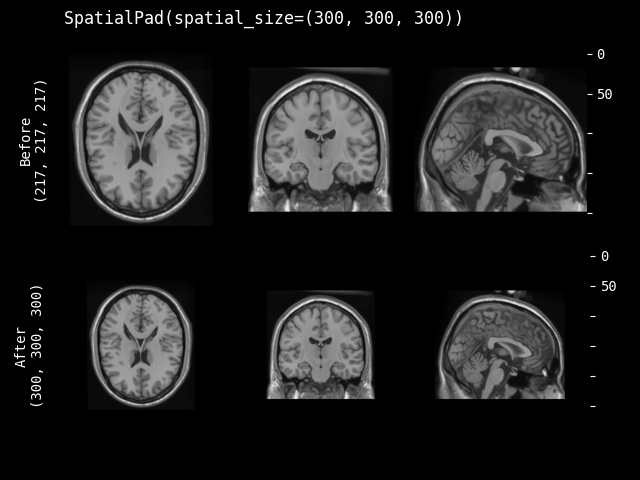Performs padding to the data, symmetric for all sides or all on one side for each dimension.

This transform is capable of lazy execution. See the Lazy Resampling topic for more information.

Parameters:
• spatial_size – the spatial size of output data after padding, if a dimension of the input data size is larger than the pad size, will not pad that dimension. If its components have non-positive values, the corresponding size of input image will be used (no padding). for example: if the spatial size of input data is [30, 30, 30] and spatial_size=[32, 25, -1], the spatial size of output data will be [32, 30, 30].

• method – {`"symmetric"`, `"end"`} Pad image symmetrically on every side or only pad at the end sides. Defaults to `"symmetric"`.

• mode – available modes for numpy array:{`"constant"`, `"edge"`, `"linear_ramp"`, `"maximum"`, `"mean"`, `"median"`, `"minimum"`, `"reflect"`, `"symmetric"`, `"wrap"`, `"empty"`} available modes for PyTorch Tensor: {`"constant"`, `"reflect"`, `"replicate"`, `"circular"`}. One of the listed string values or a user supplied function. Defaults to `"constant"`. See also: https://numpy.org/doc/1.18/reference/generated/numpy.pad.html https://pytorch.org/docs/stable/generated/torch.nn.functional.pad.html

• lazy – a flag to indicate whether this transform should execute lazily or not. Defaults to False.

• kwargs – other arguments for the np.pad or torch.pad function. note that np.pad treats channel dimension as the first dimension.

dynamically compute the pad width according to the spatial shape.

Parameters:

spatial_shape (`Sequence`[`int`]) – spatial shape of the original image.

Return type:

`tuple`[`tuple`[`int`, `int`]]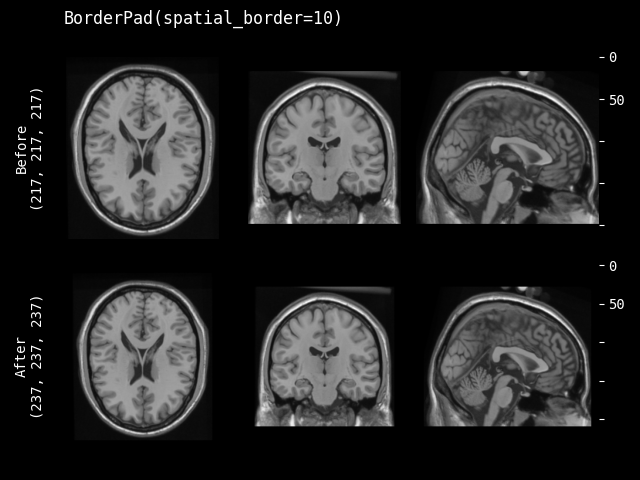This transform is capable of lazy execution. See the Lazy Resampling topic for more information.

Parameters:
• spatial_border

specified size for every spatial border. Any -ve values will be set to 0. It can be 3 shapes:

• single int number, pad all the borders with the same size.

• length equals the length of image shape, pad every spatial dimension separately. for example, image shape(CHW) is [1, 4, 4], spatial_border is [2, 1], pad every border of H dim with 2, pad every border of W dim with 1, result shape is [1, 8, 6].

• length equals 2 x (length of image shape), pad every border of every dimension separately. for example, image shape(CHW) is [1, 4, 4], spatial_border is [1, 2, 3, 4], pad top of H dim with 1, pad bottom of H dim with 2, pad left of W dim with 3, pad right of W dim with 4. the result shape is [1, 7, 11].

• mode – available modes for numpy array:{`"constant"`, `"edge"`, `"linear_ramp"`, `"maximum"`, `"mean"`, `"median"`, `"minimum"`, `"reflect"`, `"symmetric"`, `"wrap"`, `"empty"`} available modes for PyTorch Tensor: {`"constant"`, `"reflect"`, `"replicate"`, `"circular"`}. One of the listed string values or a user supplied function. Defaults to `"constant"`. See also: https://numpy.org/doc/1.18/reference/generated/numpy.pad.html https://pytorch.org/docs/stable/generated/torch.nn.functional.pad.html

• lazy – a flag to indicate whether this transform should execute lazily or not. Defaults to False.

• kwargs – other arguments for the np.pad or torch.pad function. note that np.pad treats channel dimension as the first dimension.

dynamically compute the pad width according to the spatial shape. the output is the amount of padding for all dimensions including the channel.

Parameters:

spatial_shape (`Sequence`[`int`]) – spatial shape of the original image.

Return type:

`tuple`[`tuple`[`int`, `int`]]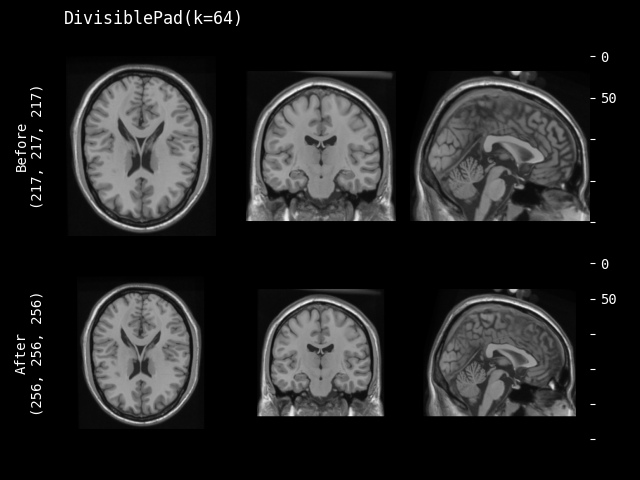Pad the input data, so that the spatial sizes are divisible by k.

This transform is capable of lazy execution. See the Lazy Resampling topic for more information.

Parameters:
• k – the target k for each spatial dimension. if k is negative or 0, the original size is preserved. if k is an int, the same k be applied to all the input spatial dimensions.

• mode – available modes for numpy array:{`"constant"`, `"edge"`, `"linear_ramp"`, `"maximum"`, `"mean"`, `"median"`, `"minimum"`, `"reflect"`, `"symmetric"`, `"wrap"`, `"empty"`} available modes for PyTorch Tensor: {`"constant"`, `"reflect"`, `"replicate"`, `"circular"`}. One of the listed string values or a user supplied function. Defaults to `"constant"`. See also: https://numpy.org/doc/1.18/reference/generated/numpy.pad.html https://pytorch.org/docs/stable/generated/torch.nn.functional.pad.html

• method – {`"symmetric"`, `"end"`} Pad image symmetrically on every side or only pad at the end sides. Defaults to `"symmetric"`.

• lazy – a flag to indicate whether this transform should execute lazily or not. Defaults to False.

• kwargs – other arguments for the np.pad or torch.pad function. note that np.pad treats channel dimension as the first dimension.

dynamically compute the pad width according to the spatial shape. the output is the amount of padding for all dimensions including the channel.

Parameters:

spatial_shape (`Sequence`[`int`]) – spatial shape of the original image.

Return type:

`tuple`[`tuple`[`int`, `int`]]

#### Crop#

class monai.transforms.Crop(lazy=False)[source]#

Perform crop operations on the input image.

This transform is capable of lazy execution. See the Lazy Resampling topic for more information.

Parameters:

lazy (`bool`) – a flag to indicate whether this transform should execute lazily or not. Defaults to False.

__call__(img, slices, lazy=None)[source]#

Apply the transform to img, assuming img is channel-first and slicing doesn’t apply to the channel dim.

static compute_slices(roi_center=None, roi_size=None, roi_start=None, roi_end=None, roi_slices=None)[source]#

Compute the crop slices based on specified center & size or start & end or slices.

Parameters:
• roi_center – voxel coordinates for center of the crop ROI.

• roi_size – size of the crop ROI, if a dimension of ROI size is larger than image size, will not crop that dimension of the image.

• roi_start – voxel coordinates for start of the crop ROI.

• roi_end – voxel coordinates for end of the crop ROI, if a coordinate is out of image, use the end coordinate of image.

• roi_slices – list of slices for each of the spatial dimensions.

inverse(img)[source]#

Inverse of `__call__`.

Raises:

NotImplementedError – When the subclass does not override this method.

Return type:

`MetaTensor`

#### SpatialCrop#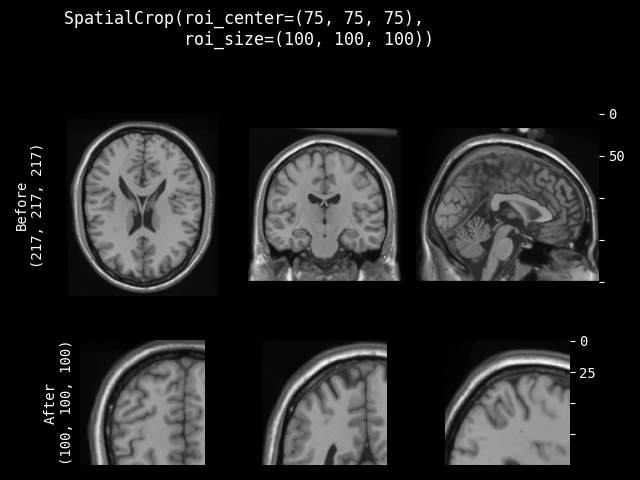class monai.transforms.SpatialCrop(roi_center=None, roi_size=None, roi_start=None, roi_end=None, roi_slices=None, lazy=False)[source]#

General purpose cropper to produce sub-volume region of interest (ROI). If a dimension of the expected ROI size is larger than the input image size, will not crop that dimension. So the cropped result may be smaller than the expected ROI, and the cropped results of several images may not have exactly the same shape. It can support to crop ND spatial (channel-first) data.

The cropped region can be parameterised in various ways:
• a list of slices for each spatial dimension (allows for use of negative indexing and None)

• a spatial center and size

• the start and end coordinates of the ROI

This transform is capable of lazy execution. See the Lazy Resampling topic for more information.

__call__(img, lazy=None)[source]#

Apply the transform to img, assuming img is channel-first and slicing doesn’t apply to the channel dim.

__init__(roi_center=None, roi_size=None, roi_start=None, roi_end=None, roi_slices=None, lazy=False)[source]#
Parameters:
• roi_center – voxel coordinates for center of the crop ROI.

• roi_size – size of the crop ROI, if a dimension of ROI size is larger than image size, will not crop that dimension of the image.

• roi_start – voxel coordinates for start of the crop ROI.

• roi_end – voxel coordinates for end of the crop ROI, if a coordinate is out of image, use the end coordinate of image.

• roi_slices – list of slices for each of the spatial dimensions.

• lazy – a flag to indicate whether this transform should execute lazily or not. Defaults to False.

#### CenterSpatialCrop#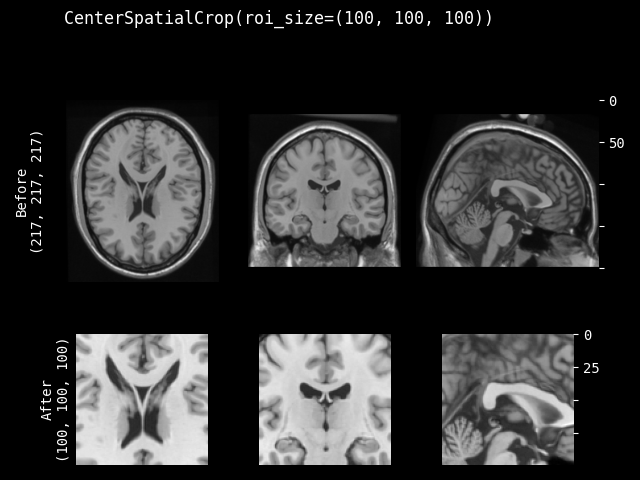class monai.transforms.CenterSpatialCrop(roi_size, lazy=False)[source]#

Crop at the center of image with specified ROI size. If a dimension of the expected ROI size is larger than the input image size, will not crop that dimension. So the cropped result may be smaller than the expected ROI, and the cropped results of several images may not have exactly the same shape.

This transform is capable of lazy execution. See the Lazy Resampling topic for more information.

Parameters:
• roi_size – the spatial size of the crop region e.g. [224,224,128] if a dimension of ROI size is larger than image size, will not crop that dimension of the image. If its components have non-positive values, the corresponding size of input image will be used. for example: if the spatial size of input data is [40, 40, 40] and roi_size=[32, 64, -1], the spatial size of output data will be [32, 40, 40].

• lazy – a flag to indicate whether this transform should execute lazily or not. Defaults to False.

__call__(img, lazy=None)[source]#

Apply the transform to img, assuming img is channel-first and slicing doesn’t apply to the channel dim.

compute_slices(spatial_size)[source]#

Compute the crop slices based on specified center & size or start & end or slices.

Parameters:
• roi_center – voxel coordinates for center of the crop ROI.

• roi_size – size of the crop ROI, if a dimension of ROI size is larger than image size, will not crop that dimension of the image.

• roi_start – voxel coordinates for start of the crop ROI.

• roi_end – voxel coordinates for end of the crop ROI, if a coordinate is out of image, use the end coordinate of image.

• roi_slices – list of slices for each of the spatial dimensions.

Return type:

`tuple`[`slice`]

#### RandSpatialCrop#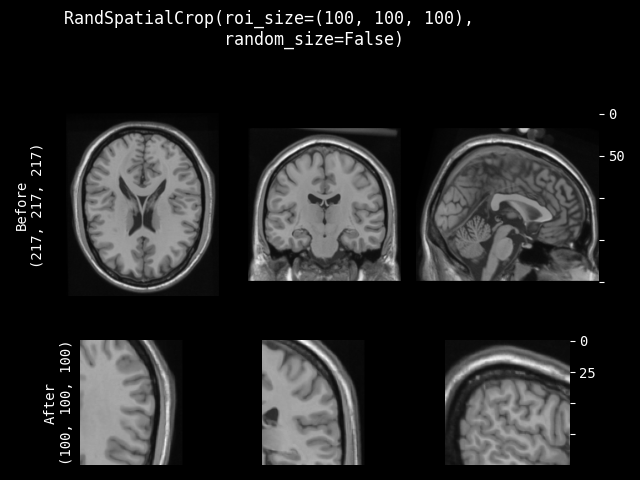class monai.transforms.RandSpatialCrop(roi_size, max_roi_size=None, random_center=True, random_size=False, lazy=False)[source]#

Crop image with random size or specific size ROI. It can crop at a random position as center or at the image center. And allows to set the minimum and maximum size to limit the randomly generated ROI.

Note: even random_size=False, if a dimension of the expected ROI size is larger than the input image size, will not crop that dimension. So the cropped result may be smaller than the expected ROI, and the cropped results of several images may not have exactly the same shape.

This transform is capable of lazy execution. See the Lazy Resampling topic for more information.

Parameters:
• roi_size – if random_size is True, it specifies the minimum crop region. if random_size is False, it specifies the expected ROI size to crop. e.g. [224, 224, 128] if a dimension of ROI size is larger than image size, will not crop that dimension of the image. If its components have non-positive values, the corresponding size of input image will be used. for example: if the spatial size of input data is [40, 40, 40] and roi_size=[32, 64, -1], the spatial size of output data will be [32, 40, 40].

• max_roi_size – if random_size is True and roi_size specifies the min crop region size, max_roi_size can specify the max crop region size. if None, defaults to the input image size. if its components have non-positive values, the corresponding size of input image will be used.

• random_center – crop at random position as center or the image center.

• random_size – crop with random size or specific size ROI. if True, the actual size is sampled from randint(roi_size, max_roi_size + 1).

• lazy – a flag to indicate whether this transform should execute lazily or not. Defaults to False.

__call__(img, randomize=True, lazy=None)[source]#

Apply the transform to img, assuming img is channel-first and slicing doesn’t apply to the channel dim.

randomize(img_size)[source]#

Within this method, `self.R` should be used, instead of np.random, to introduce random factors.

all `self.R` calls happen here so that we have a better chance to identify errors of sync the random state.

This method can generate the random factors based on properties of the input data.

Raises:

NotImplementedError – When the subclass does not override this method.

Return type:

`None`

#### RandSpatialCropSamples#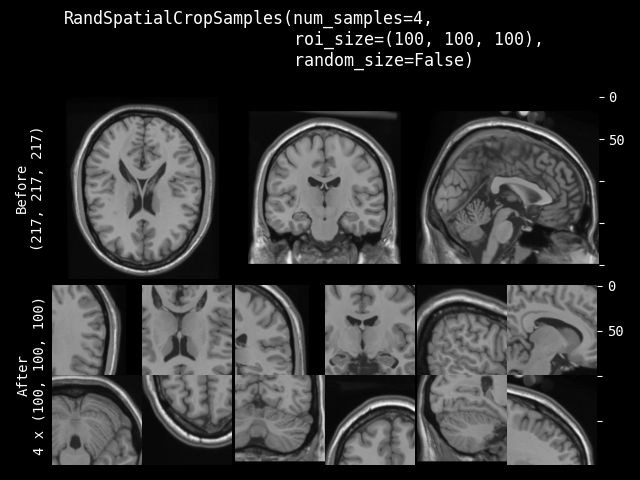class monai.transforms.RandSpatialCropSamples(roi_size, num_samples, max_roi_size=None, random_center=True, random_size=False, lazy=False)[source]#

Crop image with random size or specific size ROI to generate a list of N samples. It can crop at a random position as center or at the image center. And allows to set the minimum size to limit the randomly generated ROI. It will return a list of cropped images.

Note: even random_size=False, if a dimension of the expected ROI size is larger than the input image size, will not crop that dimension. So the cropped result may be smaller than the expected ROI, and the cropped results of several images may not have exactly the same shape.

This transform is capable of lazy execution. See the Lazy Resampling topic for more information.

Parameters:
• roi_size – if random_size is True, it specifies the minimum crop region. if random_size is False, it specifies the expected ROI size to crop. e.g. [224, 224, 128] if a dimension of ROI size is larger than image size, will not crop that dimension of the image. If its components have non-positive values, the corresponding size of input image will be used. for example: if the spatial size of input data is [40, 40, 40] and roi_size=[32, 64, -1], the spatial size of output data will be [32, 40, 40].

• num_samples – number of samples (crop regions) to take in the returned list.

• max_roi_size – if random_size is True and roi_size specifies the min crop region size, max_roi_size can specify the max crop region size. if None, defaults to the input image size. if its components have non-positive values, the corresponding size of input image will be used.

• random_center – crop at random position as center or the image center.

• random_size – crop with random size or specific size ROI. The actual size is sampled from randint(roi_size, img_size).

• lazy – a flag to indicate whether this transform should execute lazily or not. Defaults to False.

Raises:

ValueError – When `num_samples` is nonpositive.

__call__(img, lazy=None)[source]#

Apply the transform to img, assuming img is channel-first and cropping doesn’t change the channel dim.

property lazy#

Get whether lazy evaluation is enabled for this transform instance. :returns: True if the transform is operating in a lazy fashion, False if not.

randomize(data=None)[source]#

Within this method, `self.R` should be used, instead of np.random, to introduce random factors.

all `self.R` calls happen here so that we have a better chance to identify errors of sync the random state.

This method can generate the random factors based on properties of the input data.

Raises:

NotImplementedError – When the subclass does not override this method.

set_random_state(seed=None, state=None)[source]#

Set the random state locally, to control the randomness, the derived classes should use `self.R` instead of np.random to introduce random factors.

Parameters:
• seed – set the random state with an integer seed.

• state – set the random state with a np.random.RandomState object.

Raises:

TypeError – When `state` is not an `Optional[np.random.RandomState]`.

Returns:

a Randomizable instance.

#### CropForeground#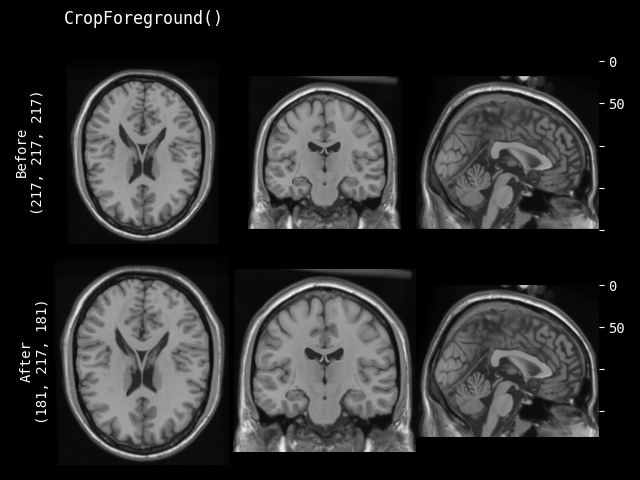Crop an image using a bounding box. The bounding box is generated by selecting foreground using select_fn at channels channel_indices. margin is added in each spatial dimension of the bounding box. The typical usage is to help training and evaluation if the valid part is small in the whole medical image. Users can define arbitrary function to select expected foreground from the whole image or specified channels. And it can also add margin to every dim of the bounding box of foreground object. For example:

```image = np.array(
[[[0, 0, 0, 0, 0],
[0, 1, 2, 1, 0],
[0, 1, 3, 2, 0],
[0, 1, 2, 1, 0],
[0, 0, 0, 0, 0]]])  # 1x5x5, single channel 5x5 image

def threshold_at_one(x):
# threshold at 1
return x > 1

cropper = CropForeground(select_fn=threshold_at_one, margin=0)
print(cropper(image))
[[[2, 1],
[3, 2],
[2, 1]]]
```

This transform is capable of lazy execution. See the Lazy Resampling topic for more information.

Apply the transform to img, assuming img is channel-first and slicing doesn’t change the channel dim.

Parameters:
• select_fn – function to select expected foreground, default is to select values > 0.

• channel_indices – if defined, select foreground only on the specified channels of image. if None, select foreground on the whole image.

• margin – add margin value to spatial dims of the bounding box, if only 1 value provided, use it for all dims.

• allow_smaller – when computing box size with margin, whether to allow the image edges to be smaller than the final box edges. If False, part of a padded output box might be outside of the original image, if True, the image edges will be used as the box edges. Default to True.

• return_coords – whether return the coordinates of spatial bounding box for foreground.

• k_divisible – make each spatial dimension to be divisible by k, default to 1. if k_divisible is an int, the same k be applied to all the input spatial dimensions.

• mode – available modes for numpy array:{`"constant"`, `"edge"`, `"linear_ramp"`, `"maximum"`, `"mean"`, `"median"`, `"minimum"`, `"reflect"`, `"symmetric"`, `"wrap"`, `"empty"`} available modes for PyTorch Tensor: {`"constant"`, `"reflect"`, `"replicate"`, `"circular"`}. One of the listed string values or a user supplied function. Defaults to `"constant"`. See also: https://numpy.org/doc/1.18/reference/generated/numpy.pad.html https://pytorch.org/docs/stable/generated/torch.nn.functional.pad.html

• lazy – a flag to indicate whether this transform should execute lazily or not. Defaults to False.

compute_bounding_box(img)[source]#

Compute the start points and end points of bounding box to crop. And adjust bounding box coords to be divisible by k.

Return type:

`tuple`[`ndarray`, `ndarray`]

Crop and pad based on the bounding box.

inverse(img)[source]#

Inverse of `__call__`.

Raises:

NotImplementedError – When the subclass does not override this method.

Return type:

`MetaTensor`

property lazy#

Get whether lazy evaluation is enabled for this transform instance. :returns: True if the transform is operating in a lazy fashion, False if not.

property requires_current_data#

Get whether the transform requires the input data to be up to date before the transform executes. Such transforms can still execute lazily by adding pending operations to the output tensors. :returns: True if the transform requires its inputs to be up to date and False if it does not

#### RandWeightedCrop#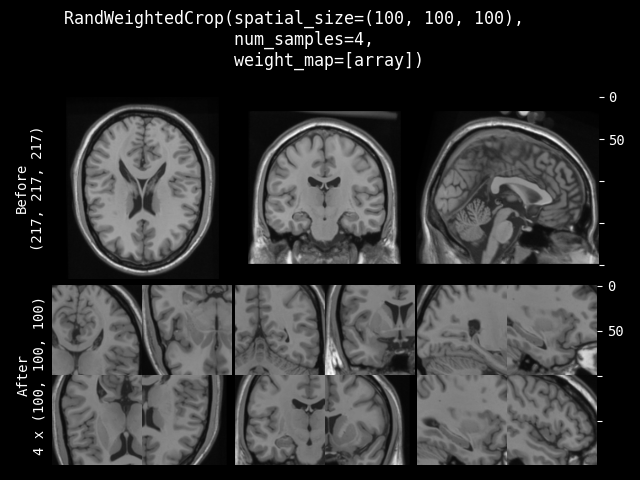class monai.transforms.RandWeightedCrop(spatial_size, num_samples=1, weight_map=None, lazy=False)[source]#

Samples a list of num_samples image patches according to the provided weight_map.

This transform is capable of lazy execution. See the Lazy Resampling topic for more information.

Parameters:
• spatial_size – the spatial size of the image patch e.g. [224, 224, 128]. If its components have non-positive values, the corresponding size of img will be used.

• num_samples – number of samples (image patches) to take in the returned list.

• weight_map – weight map used to generate patch samples. The weights must be non-negative. Each element denotes a sampling weight of the spatial location. 0 indicates no sampling. It should be a single-channel array in shape, for example, (1, spatial_dim_0, spatial_dim_1, …).

• lazy – a flag to indicate whether this transform should execute lazily or not. Defaults to False.

__call__(img, weight_map=None, randomize=True, lazy=None)[source]#
Parameters:
• img – input image to sample patches from. assuming img is a channel-first array.

• weight_map – weight map used to generate patch samples. The weights must be non-negative. Each element denotes a sampling weight of the spatial location. 0 indicates no sampling. It should be a single-channel array in shape, for example, (1, spatial_dim_0, spatial_dim_1, …)

• randomize – whether to execute random operations, default to True.

• lazy – a flag to override the lazy behaviour for this call, if set. Defaults to None.

Returns:

A list of image patches

property lazy#

Get whether lazy evaluation is enabled for this transform instance. :returns: True if the transform is operating in a lazy fashion, False if not.

randomize(weight_map)[source]#

Within this method, `self.R` should be used, instead of np.random, to introduce random factors.

all `self.R` calls happen here so that we have a better chance to identify errors of sync the random state.

This method can generate the random factors based on properties of the input data.

Raises:

NotImplementedError – When the subclass does not override this method.

Return type:

`None`

#### RandCropByPosNegLabel#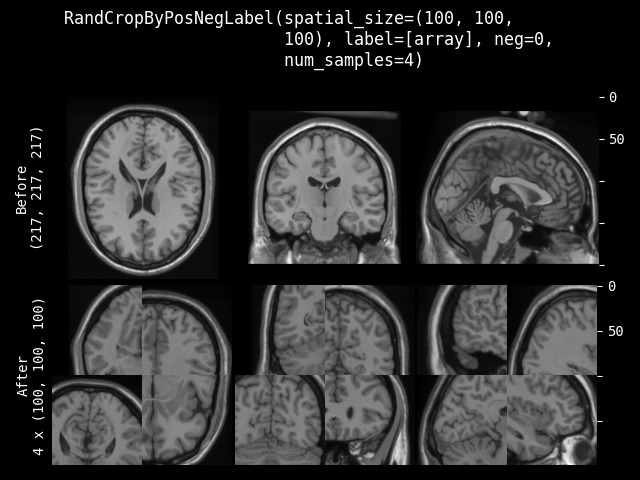class monai.transforms.RandCropByPosNegLabel(spatial_size, label=None, pos=1.0, neg=1.0, num_samples=1, image=None, image_threshold=0.0, fg_indices=None, bg_indices=None, allow_smaller=False, lazy=False)[source]#

Crop random fixed sized regions with the center being a foreground or background voxel based on the Pos Neg Ratio. And will return a list of arrays for all the cropped images. For example, crop two (3 x 3) arrays from (5 x 5) array with pos/neg=1:

```[[[0, 0, 0, 0, 0],
[0, 1, 2, 1, 0],            [[0, 1, 2],     [[2, 1, 0],
[0, 1, 3, 0, 0],     -->     [0, 1, 3],      [3, 0, 0],
[0, 0, 0, 0, 0],             [0, 0, 0]]      [0, 0, 0]]
[0, 0, 0, 0, 0]]]
```

If a dimension of the expected spatial size is larger than the input image size, will not crop that dimension. So the cropped result may be smaller than expected size, and the cropped results of several images may not have exactly same shape. And if the crop ROI is partly out of the image, will automatically adjust the crop center to ensure the valid crop ROI.

This transform is capable of lazy execution. See the Lazy Resampling topic for more information.

Parameters:
• spatial_size – the spatial size of the crop region e.g. [224, 224, 128]. if a dimension of ROI size is larger than image size, will not crop that dimension of the image. if its components have non-positive values, the corresponding size of label will be used. for example: if the spatial size of input data is [40, 40, 40] and spatial_size=[32, 64, -1], the spatial size of output data will be [32, 40, 40].

• label – the label image that is used for finding foreground/background, if None, must set at self.__call__. Non-zero indicates foreground, zero indicates background.

• pos – used with neg together to calculate the ratio `pos / (pos + neg)` for the probability to pick a foreground voxel as a center rather than a background voxel.

• neg – used with pos together to calculate the ratio `pos / (pos + neg)` for the probability to pick a foreground voxel as a center rather than a background voxel.

• num_samples – number of samples (crop regions) to take in each list.

• image – optional image data to help select valid area, can be same as img or another image array. if not None, use `label == 0 & image > image_threshold` to select the negative sample (background) center. So the crop center will only come from the valid image areas.

• image_threshold – if enabled image, use `image > image_threshold` to determine the valid image content areas.

• fg_indices – if provided pre-computed foreground indices of label, will ignore above image and image_threshold, and randomly select crop centers based on them, need to provide fg_indices and bg_indices together, expect to be 1 dim array of spatial indices after flattening. a typical usage is to call FgBgToIndices transform first and cache the results.

• bg_indices – if provided pre-computed background indices of label, will ignore above image and image_threshold, and randomly select crop centers based on them, need to provide fg_indices and bg_indices together, expect to be 1 dim array of spatial indices after flattening. a typical usage is to call FgBgToIndices transform first and cache the results.

• allow_smaller – if False, an exception will be raised if the image is smaller than the requested ROI in any dimension. If True, any smaller dimensions will be set to match the cropped size (i.e., no cropping in that dimension).

• lazy – a flag to indicate whether this transform should execute lazily or not. Defaults to False.

Raises:
• ValueError – When `pos` or `neg` are negative.

• ValueError – When `pos=0` and `neg=0`. Incompatible values.

__call__(img, label=None, image=None, fg_indices=None, bg_indices=None, randomize=True, lazy=None)[source]#
Parameters:
• img – input data to crop samples from based on the pos/neg ratio of label and image. Assumes img is a channel-first array.

• label – the label image that is used for finding foreground/background, if None, use self.label.

• image – optional image data to help select valid area, can be same as img or another image array. use `label == 0 & image > image_threshold` to select the negative sample(background) center. so the crop center will only exist on valid image area. if None, use self.image.

• fg_indices – foreground indices to randomly select crop centers, need to provide fg_indices and bg_indices together.

• bg_indices – background indices to randomly select crop centers, need to provide fg_indices and bg_indices together.

• randomize – whether to execute the random operations, default to True.

• lazy – a flag to override the lazy behaviour for this call, if set. Defaults to None.

property lazy#

Get whether lazy evaluation is enabled for this transform instance. :returns: True if the transform is operating in a lazy fashion, False if not.

randomize(label=None, fg_indices=None, bg_indices=None, image=None)[source]#

Within this method, `self.R` should be used, instead of np.random, to introduce random factors.

all `self.R` calls happen here so that we have a better chance to identify errors of sync the random state.

This method can generate the random factors based on properties of the input data.

Raises:

NotImplementedError – When the subclass does not override this method.

property requires_current_data#

Get whether the transform requires the input data to be up to date before the transform executes. Such transforms can still execute lazily by adding pending operations to the output tensors. :returns: True if the transform requires its inputs to be up to date and False if it does not

#### RandCropByLabelClasses#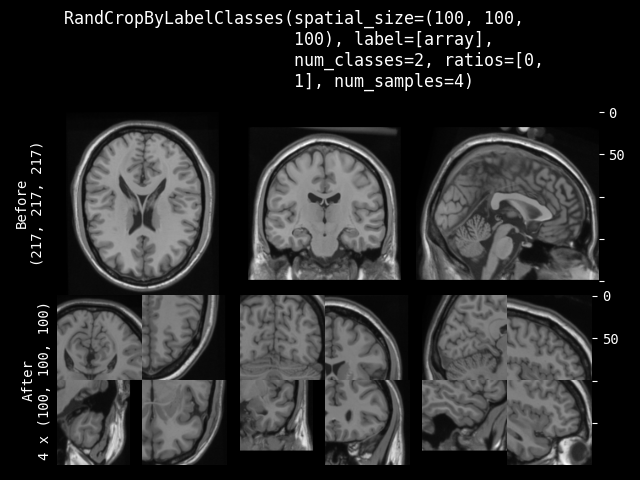class monai.transforms.RandCropByLabelClasses(spatial_size, ratios=None, label=None, num_classes=None, num_samples=1, image=None, image_threshold=0.0, indices=None, allow_smaller=False, warn=True, max_samples_per_class=None, lazy=False)[source]#

Crop random fixed sized regions with the center being a class based on the specified ratios of every class. The label data can be One-Hot format array or Argmax data. And will return a list of arrays for all the cropped images. For example, crop two (3 x 3) arrays from (5 x 5) array with ratios=[1, 2, 3, 1]:

```image = np.array([
[[0.0, 0.3, 0.4, 0.2, 0.0],
[0.0, 0.1, 0.2, 0.1, 0.4],
[0.0, 0.3, 0.5, 0.2, 0.0],
[0.1, 0.2, 0.1, 0.1, 0.0],
[0.0, 0.1, 0.2, 0.1, 0.0]]
])
label = np.array([
[[0, 0, 0, 0, 0],
[0, 1, 2, 1, 0],
[0, 1, 3, 0, 0],
[0, 0, 0, 0, 0],
[0, 0, 0, 0, 0]]
])
cropper = RandCropByLabelClasses(
spatial_size=[3, 3],
ratios=[1, 2, 3, 1],
num_classes=4,
num_samples=2,
)
label_samples = cropper(img=label, label=label, image=image)

The 2 randomly cropped samples of `label` can be:
[[0, 1, 2],     [[0, 0, 0],
[0, 1, 3],      [1, 2, 1],
[0, 0, 0]]      [1, 3, 0]]
```

If a dimension of the expected spatial size is larger than the input image size, will not crop that dimension. So the cropped result may be smaller than expected size, and the cropped results of several images may not have exactly same shape. And if the crop ROI is partly out of the image, will automatically adjust the crop center to ensure the valid crop ROI.

This transform is capable of lazy execution. See the Lazy Resampling topic for more information.

Parameters:
• spatial_size – the spatial size of the crop region e.g. [224, 224, 128]. if a dimension of ROI size is larger than image size, will not crop that dimension of the image. if its components have non-positive values, the corresponding size of label will be used. for example: if the spatial size of input data is [40, 40, 40] and spatial_size=[32, 64, -1], the spatial size of output data will be [32, 40, 40].

• ratios – specified ratios of every class in the label to generate crop centers, including background class. if None, every class will have the same ratio to generate crop centers.

• label – the label image that is used for finding every class, if None, must set at self.__call__.

• num_classes – number of classes for argmax label, not necessary for One-Hot label.

• num_samples – number of samples (crop regions) to take in each list.

• image – if image is not None, only return the indices of every class that are within the valid region of the image (`image > image_threshold`).

• image_threshold – if enabled image, use `image > image_threshold` to determine the valid image content area and select class indices only in this area.

• indices – if provided pre-computed indices of every class, will ignore above image and image_threshold, and randomly select crop centers based on them, expect to be 1 dim array of spatial indices after flattening. a typical usage is to call ClassesToIndices transform first and cache the results for better performance.

• allow_smaller – if False, an exception will be raised if the image is smaller than the requested ROI in any dimension. If True, any smaller dimensions will remain unchanged.

• warn – if True prints a warning if a class is not present in the label.

• max_samples_per_class – maximum length of indices to sample in each class to reduce memory consumption. Default is None, no subsampling.

• lazy – a flag to indicate whether this transform should execute lazily or not. Defaults to False.

__call__(img, label=None, image=None, indices=None, randomize=True, lazy=None)[source]#
Parameters:
• img – input data to crop samples from based on the ratios of every class, assumes img is a channel-first array.

• label – the label image that is used for finding indices of every class, if None, use self.label.

• image – optional image data to help select valid area, can be same as img or another image array. use `image > image_threshold` to select the centers only in valid region. if None, use self.image.

• indices – list of indices for every class in the image, used to randomly select crop centers.

• randomize – whether to execute the random operations, default to True.

• lazy – a flag to override the lazy behaviour for this call, if set. Defaults to None.

property lazy#

Get whether lazy evaluation is enabled for this transform instance. :returns: True if the transform is operating in a lazy fashion, False if not.

randomize(label=None, indices=None, image=None)[source]#

Within this method, `self.R` should be used, instead of np.random, to introduce random factors.

all `self.R` calls happen here so that we have a better chance to identify errors of sync the random state.

This method can generate the random factors based on properties of the input data.

Raises:

NotImplementedError – When the subclass does not override this method.

property requires_current_data#

Get whether the transform requires the input data to be up to date before the transform executes. Such transforms can still execute lazily by adding pending operations to the output tensors. :returns: True if the transform requires its inputs to be up to date and False if it does not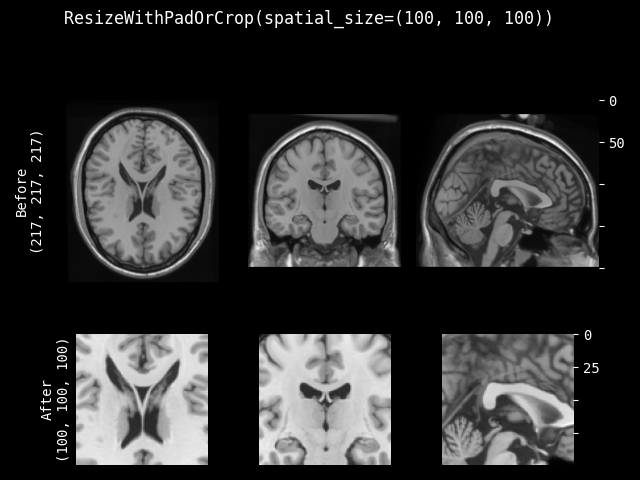Resize an image to a target spatial size by either centrally cropping the image or padding it evenly with a user-specified mode. When the dimension is smaller than the target size, do symmetric padding along that dim. When the dimension is larger than the target size, do central cropping along that dim.

This transform is capable of lazy execution. See the Lazy Resampling topic for more information.

Parameters:
• spatial_size – the spatial size of output data after padding or crop. If has non-positive values, the corresponding size of input image will be used (no padding).

• method – {`"symmetric"`, `"end"`} Pad image symmetrically on every side or only pad at the end sides. Defaults to `"symmetric"`.

• mode – available modes for numpy array:{`"constant"`, `"edge"`, `"linear_ramp"`, `"maximum"`, `"mean"`, `"median"`, `"minimum"`, `"reflect"`, `"symmetric"`, `"wrap"`, `"empty"`} available modes for PyTorch Tensor: {`"constant"`, `"reflect"`, `"replicate"`, `"circular"`}. One of the listed string values or a user supplied function. Defaults to `"constant"`. See also: https://numpy.org/doc/1.18/reference/generated/numpy.pad.html https://pytorch.org/docs/stable/generated/torch.nn.functional.pad.html

• lazy – a flag to indicate whether this transform should execute lazily or not. Defaults to False.

Parameters:
• img – data to pad or crop, assuming img is channel-first and padding or cropping doesn’t apply to the channel dim.

• mode – available modes for numpy array:{`"constant"`, `"edge"`, `"linear_ramp"`, `"maximum"`, `"mean"`, `"median"`, `"minimum"`, `"reflect"`, `"symmetric"`, `"wrap"`, `"empty"`} available modes for PyTorch Tensor: {`"constant"`, `"reflect"`, `"replicate"`, `"circular"`}. One of the listed string values or a user supplied function. Defaults to `"constant"`. See also: https://numpy.org/doc/1.18/reference/generated/numpy.pad.html https://pytorch.org/docs/stable/generated/torch.nn.functional.pad.html

• lazy – a flag to override the lazy behaviour for this call, if set. Defaults to None.

inverse(img)[source]#

Inverse of `__call__`.

Raises:

NotImplementedError – When the subclass does not override this method.

Return type:

`MetaTensor`

property lazy#

Get whether lazy evaluation is enabled for this transform instance. :returns: True if the transform is operating in a lazy fashion, False if not.

#### BoundingRect#

class monai.transforms.BoundingRect(select_fn=<function is_positive>)[source]#

Compute coordinates of axis-aligned bounding rectangles from input image img. The output format of the coordinates is (shape is [channel, 2 * spatial dims]):

[[1st_spatial_dim_start, 1st_spatial_dim_end,

2nd_spatial_dim_start, 2nd_spatial_dim_end, …, Nth_spatial_dim_start, Nth_spatial_dim_end],

[1st_spatial_dim_start, 1st_spatial_dim_end, 2nd_spatial_dim_start, 2nd_spatial_dim_end, …, Nth_spatial_dim_start, Nth_spatial_dim_end]]

The bounding boxes edges are aligned with the input image edges. This function returns [0, 0, …] if there’s no positive intensity.

Parameters:

select_fn (`Callable`) – function to select expected foreground, default is to select values > 0.

__call__(img)[source]#
Return type:

`ndarray`

#### RandScaleCrop#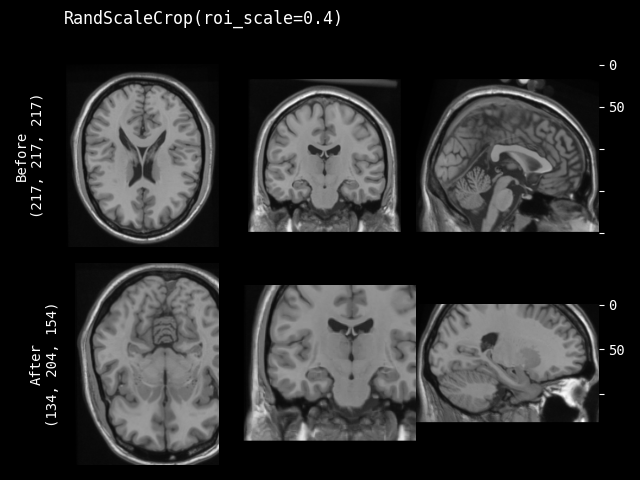class monai.transforms.RandScaleCrop(roi_scale, max_roi_scale=None, random_center=True, random_size=False, lazy=False)[source]#

Subclass of `monai.transforms.RandSpatialCrop`. Crop image with random size or specific size ROI. It can crop at a random position as center or at the image center. And allows to set the minimum and maximum scale of image size to limit the randomly generated ROI.

This transform is capable of lazy execution. See the Lazy Resampling topic for more information.

Parameters:
• roi_scale – if random_size is True, it specifies the minimum crop size: roi_scale * image spatial size. if random_size is False, it specifies the expected scale of image size to crop. e.g. [0.3, 0.4, 0.5]. If its components have non-positive values, will use 1.0 instead, which means the input image size.

• max_roi_scale – if random_size is True and roi_scale specifies the min crop region size, max_roi_scale can specify the max crop region size: max_roi_scale * image spatial size. if None, defaults to the input image size. if its components have non-positive values, will use 1.0 instead, which means the input image size.

• random_center – crop at random position as center or the image center.

• random_size – crop with random size or specified size ROI by roi_scale * image spatial size. if True, the actual size is sampled from randint(roi_scale * image spatial size, max_roi_scale * image spatial size + 1).

• lazy – a flag to indicate whether this transform should execute lazily or not. Defaults to False.

__call__(img, randomize=True, lazy=None)[source]#

Apply the transform to img, assuming img is channel-first and slicing doesn’t apply to the channel dim.

randomize(img_size)[source]#

Within this method, `self.R` should be used, instead of np.random, to introduce random factors.

all `self.R` calls happen here so that we have a better chance to identify errors of sync the random state.

This method can generate the random factors based on properties of the input data.

Raises:

NotImplementedError – When the subclass does not override this method.

Return type:

`None`

#### CenterScaleCrop#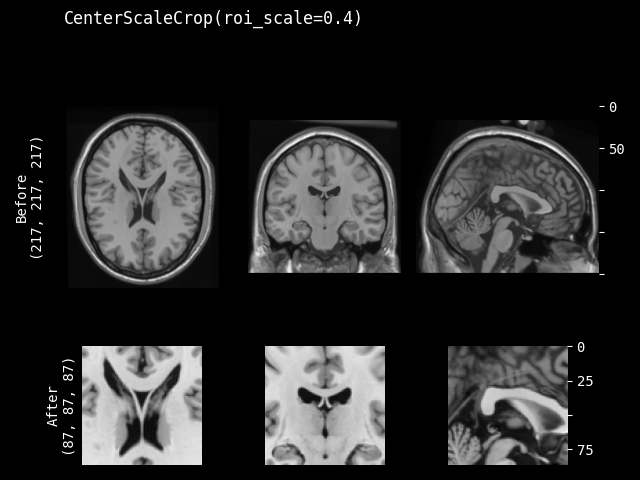class monai.transforms.CenterScaleCrop(roi_scale, lazy=False)[source]#

Crop at the center of image with specified scale of ROI size.

This transform is capable of lazy execution. See the Lazy Resampling topic for more information.

Parameters:
• roi_scale – specifies the expected scale of image size to crop. e.g. [0.3, 0.4, 0.5] or a number for all dims. If its components have non-positive values, will use 1.0 instead, which means the input image size.

• lazy – a flag to indicate whether this transform should execute lazily or not. Defaults to False.

__call__(img, lazy=None)[source]#

Apply the transform to img, assuming img is channel-first and slicing doesn’t apply to the channel dim.

### Intensity#

#### RandGaussianNoise#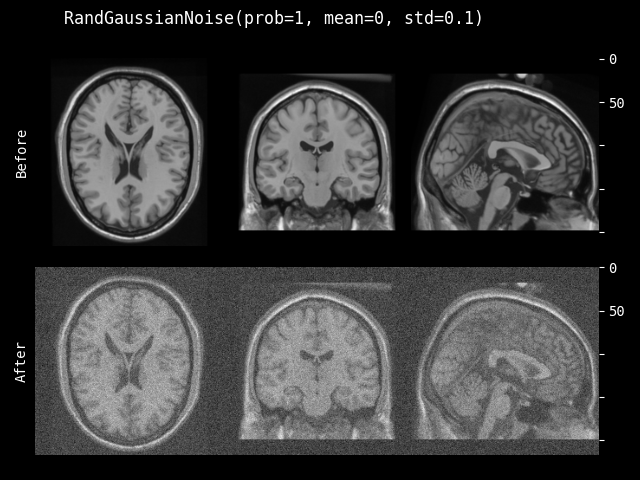class monai.transforms.RandGaussianNoise(prob=0.1, mean=0.0, std=0.1, dtype=<class 'numpy.float32'>)[source]#

Parameters:
• prob (`float`) – Probability to add Gaussian noise.

• mean (`float`) – Mean or “centre” of the distribution.

• std (`float`) – Standard deviation (spread) of distribution.

• dtype (`Union`[`dtype`, `type`, `str`, `None`]) – output data type, if None, same as input image. defaults to float32.

__call__(img, mean=None, randomize=True)[source]#

Apply the transform to img.

randomize(img, mean=None)[source]#

Within this method, `self.R` should be used, instead of np.random, to introduce random factors.

all `self.R` calls happen here so that we have a better chance to identify errors of sync the random state.

This method can generate the random factors based on properties of the input data.

#### ShiftIntensity#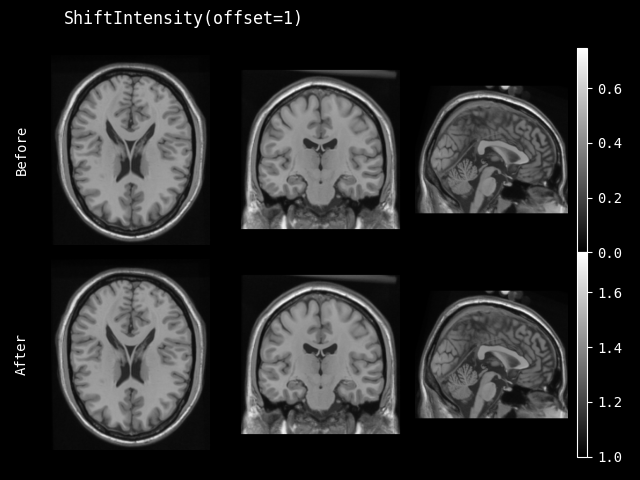class monai.transforms.ShiftIntensity(offset, safe=False)[source]#

Shift intensity uniformly for the entire image with specified offset.

Parameters:
• offset (`float`) – offset value to shift the intensity of image.

• safe (`bool`) – if True, then do safe dtype convert when intensity overflow. default to False. E.g., [256, -12] -> [array(0), array(244)]. If True, then [256, -12] -> [array(255), array(0)].

__call__(img, offset=None)[source]#

Apply the transform to img.

#### RandShiftIntensity#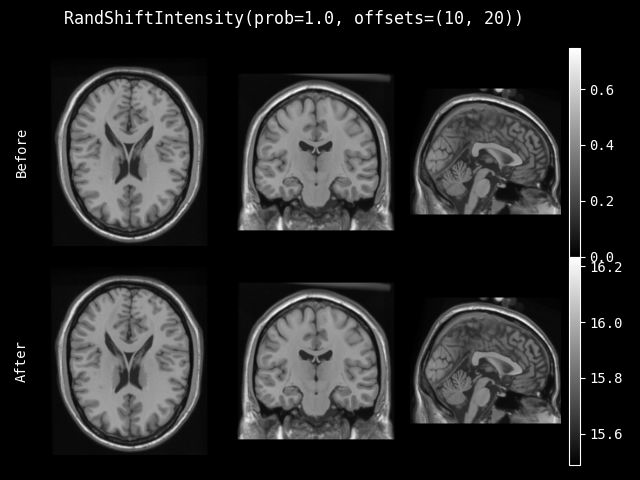class monai.transforms.RandShiftIntensity(offsets, safe=False, prob=0.1, channel_wise=False)[source]#

Randomly shift intensity with randomly picked offset.

__call__(img, factor=None, randomize=True)[source]#

Apply the transform to img.

Parameters:
• img – input image to shift intensity.

• factor – a factor to multiply the random offset, then shift. can be some image specific value at runtime, like: max(img), etc.

__init__(offsets, safe=False, prob=0.1, channel_wise=False)[source]#
Parameters:
• offsets – offset range to randomly shift. if single number, offset value is picked from (-offsets, offsets).

• safe – if True, then do safe dtype convert when intensity overflow. default to False. E.g., [256, -12] -> [array(0), array(244)]. If True, then [256, -12] -> [array(255), array(0)].

• prob – probability of shift.

• channel_wise – if True, shift intensity on each channel separately. For each channel, a random offset will be chosen. Please ensure that the first dimension represents the channel of the image if True.

randomize(data=None)[source]#

Within this method, `self.R` should be used, instead of np.random, to introduce random factors.

all `self.R` calls happen here so that we have a better chance to identify errors of sync the random state.

This method can generate the random factors based on properties of the input data.

#### StdShiftIntensity#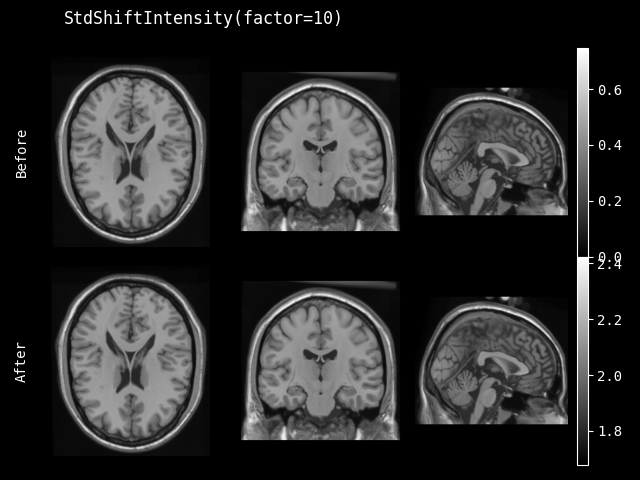class monai.transforms.StdShiftIntensity(factor, nonzero=False, channel_wise=False, dtype=<class 'numpy.float32'>)[source]#

Shift intensity for the image with a factor and the standard deviation of the image by: `v = v + factor * std(v)`. This transform can focus on only non-zero values or the entire image, and can also calculate the std on each channel separately.

Parameters:
• factor (`float`) – factor shift by `v = v + factor * std(v)`.

• nonzero (`bool`) – whether only count non-zero values.

• channel_wise (`bool`) – if True, calculate on each channel separately. Please ensure that the first dimension represents the channel of the image if True.

• dtype (`Union`[`dtype`, `type`, `str`, `None`]) – output data type, if None, same as input image. defaults to float32.

__call__(img)[source]#

Apply the transform to img.

Return type:

`Union`[`ndarray`, `Tensor`]

#### RandStdShiftIntensity#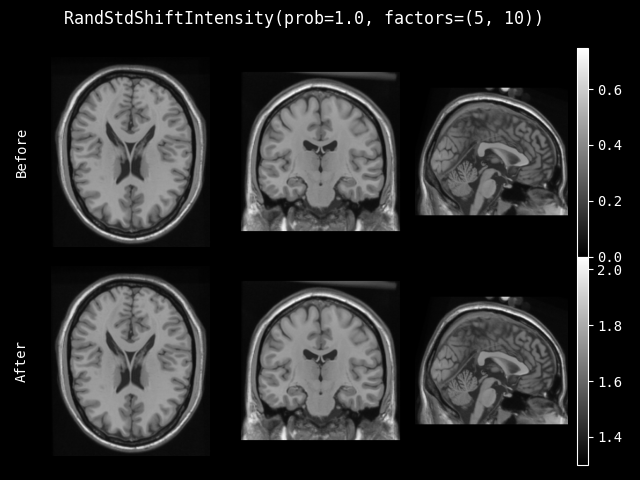class monai.transforms.RandStdShiftIntensity(factors, prob=0.1, nonzero=False, channel_wise=False, dtype=<class 'numpy.float32'>)[source]#

Shift intensity for the image with a factor and the standard deviation of the image by: `v = v + factor * std(v)` where the factor is randomly picked.

__call__(img, randomize=True)[source]#

Apply the transform to img.

Return type:

`Union`[`ndarray`, `Tensor`]

__init__(factors, prob=0.1, nonzero=False, channel_wise=False, dtype=<class 'numpy.float32'>)[source]#
Parameters:
• factors – if tuple, the randomly picked range is (min(factors), max(factors)). If single number, the range is (-factors, factors).

• prob – probability of std shift.

• nonzero – whether only count non-zero values.

• channel_wise – if True, calculate on each channel separately.

• dtype – output data type, if None, same as input image. defaults to float32.

randomize(data=None)[source]#

Within this method, `self.R` should be used, instead of np.random, to introduce random factors.

all `self.R` calls happen here so that we have a better chance to identify errors of sync the random state.

This method can generate the random factors based on properties of the input data.

#### RandBiasField#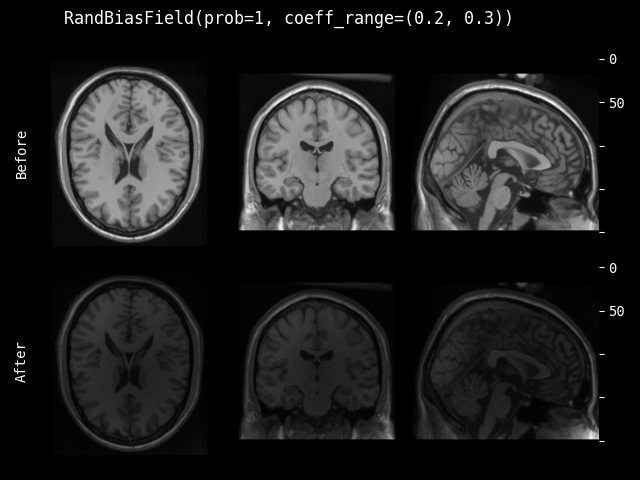class monai.transforms.RandBiasField(degree=3, coeff_range=(0.0, 0.1), dtype=<class 'numpy.float32'>, prob=0.1)[source]#

Random bias field augmentation for MR images. The bias field is considered as a linear combination of smoothly varying basis (polynomial) functions, as described in Automated Model-Based Tissue Classification of MR Images of the Brain. This implementation adapted from NiftyNet. Referred to Longitudinal segmentation of age-related white matter hyperintensities.

Parameters:
• degree (`int`) – degree of freedom of the polynomials. The value should be no less than 1. Defaults to 3.

• coeff_range (`tuple`[`float`, `float`]) – range of the random coefficients. Defaults to (0.0, 0.1).

• dtype (`Union`[`dtype`, `type`, `str`, `None`]) – output data type, if None, same as input image. defaults to float32.

• prob (`float`) – probability to do random bias field.

__call__(img, randomize=True)[source]#

Apply the transform to img.

Return type:

`Union`[`ndarray`, `Tensor`]

randomize(img_size)[source]#

Within this method, `self.R` should be used, instead of np.random, to introduce random factors.

all `self.R` calls happen here so that we have a better chance to identify errors of sync the random state.

This method can generate the random factors based on properties of the input data.

Return type:

`None`

#### ScaleIntensity#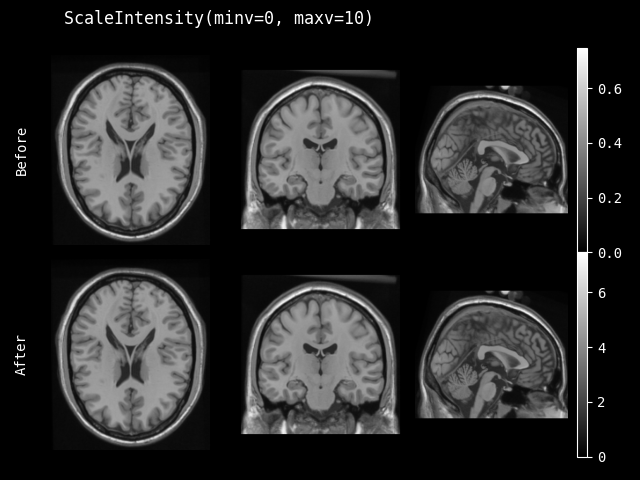class monai.transforms.ScaleIntensity(minv=0.0, maxv=1.0, factor=None, channel_wise=False, dtype=<class 'numpy.float32'>)[source]#

Scale the intensity of input image to the given value range (minv, maxv). If minv and maxv not provided, use factor to scale image by `v = v * (1 + factor)`.

__call__(img)[source]#

Apply the transform to img.

Raises:

ValueError – When `self.minv=None` or `self.maxv=None` and `self.factor=None`. Incompatible values.

Return type:

`Union`[`ndarray`, `Tensor`]

__init__(minv=0.0, maxv=1.0, factor=None, channel_wise=False, dtype=<class 'numpy.float32'>)[source]#
Parameters:
• minv – minimum value of output data.

• maxv – maximum value of output data.

• factor – factor scale by `v = v * (1 + factor)`. In order to use this parameter, please set both minv and maxv into None.

• channel_wise – if True, scale on each channel separately. Please ensure that the first dimension represents the channel of the image if True.

• dtype – output data type, if None, same as input image. defaults to float32.

#### RandScaleIntensity#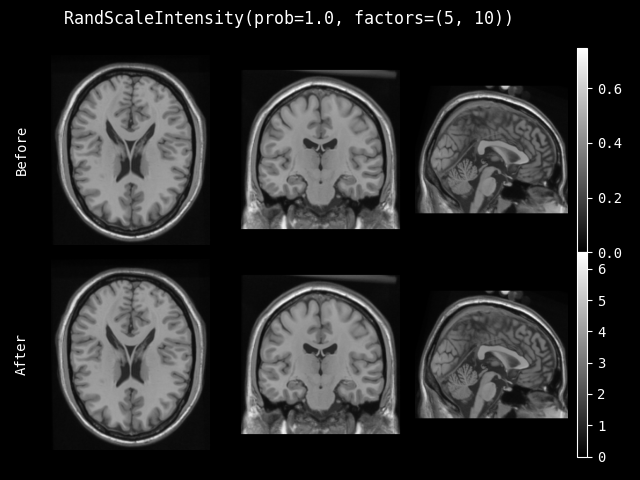class monai.transforms.RandScaleIntensity(factors, prob=0.1, channel_wise=False, dtype=<class 'numpy.float32'>)[source]#

Randomly scale the intensity of input image by `v = v * (1 + factor)` where the factor is randomly picked.

__call__(img, randomize=True)[source]#

Apply the transform to img.

Return type:

`Union`[`ndarray`, `Tensor`]

__init__(factors, prob=0.1, channel_wise=False, dtype=<class 'numpy.float32'>)[source]#
Parameters:
• factors – factor range to randomly scale by `v = v * (1 + factor)`. if single number, factor value is picked from (-factors, factors).

• prob – probability of scale.

• channel_wise – if True, scale on each channel separately. Please ensure that the first dimension represents the channel of the image if True.

• dtype – output data type, if None, same as input image. defaults to float32.

randomize(data=None)[source]#

Within this method, `self.R` should be used, instead of np.random, to introduce random factors.

all `self.R` calls happen here so that we have a better chance to identify errors of sync the random state.

This method can generate the random factors based on properties of the input data.

#### ScaleIntensityFixedMean#

class monai.transforms.ScaleIntensityFixedMean(factor=0, preserve_range=False, fixed_mean=True, channel_wise=False, dtype=<class 'numpy.float32'>)[source]#

Scale the intensity of input image by `v = v * (1 + factor)`, then shift the output so that the output image has the same mean as the input.

__call__(img, factor=None)[source]#

Apply the transform to img. :type img: `Union`[`ndarray`, `Tensor`] :param img: the input tensor/array :param factor: factor scale by `v = v * (1 + factor)`

Return type:

`Union`[`ndarray`, `Tensor`]

__init__(factor=0, preserve_range=False, fixed_mean=True, channel_wise=False, dtype=<class 'numpy.float32'>)[source]#
Parameters:
• factor (`float`) – factor scale by `v = v * (1 + factor)`.

• preserve_range (`bool`) – clips the output array/tensor to the range of the input array/tensor

• fixed_mean (`bool`) – subtract the mean intensity before scaling with factor, then add the same value after scaling to ensure that the output has the same mean as the input.

• channel_wise (`bool`) – if True, scale on each channel separately. preserve_range and fixed_mean are also applied on each channel separately if channel_wise is True. Please ensure that the first dimension represents the channel of the image if True.

• dtype (`Union`[`dtype`, `type`, `str`, `None`]) – output data type, if None, same as input image. defaults to float32.

#### RandScaleIntensityFixedMean#

class monai.transforms.RandScaleIntensityFixedMean(prob=0.1, factors=0, fixed_mean=True, preserve_range=False, dtype=<class 'numpy.float32'>)[source]#

Randomly scale the intensity of input image by `v = v * (1 + factor)` where the factor is randomly picked. Subtract the mean intensity before scaling with factor, then add the same value after scaling to ensure that the output has the same mean as the input.

__call__(img, randomize=True)[source]#

Apply the transform to img.

Return type:

`Union`[`ndarray`, `Tensor`]

__init__(prob=0.1, factors=0, fixed_mean=True, preserve_range=False, dtype=<class 'numpy.float32'>)[source]#
Parameters:
• factors – factor range to randomly scale by `v = v * (1 + factor)`. if single number, factor value is picked from (-factors, factors).

• preserve_range – clips the output array/tensor to the range of the input array/tensor

• fixed_mean – subtract the mean intensity before scaling with factor, then add the same value after scaling to ensure that the output has the same mean as the input.

• channel_wise – if True, scale on each channel separately. preserve_range and fixed_mean are also applied

• the (on each channel separately if channel_wise is True. Please ensure that the first dimension represents) –

• True. (channel of the image if) –

• dtype – output data type, if None, same as input image. defaults to float32.

randomize(data=None)[source]#

Within this method, `self.R` should be used, instead of np.random, to introduce random factors.

all `self.R` calls happen here so that we have a better chance to identify errors of sync the random state.

This method can generate the random factors based on properties of the input data.

#### NormalizeIntensity#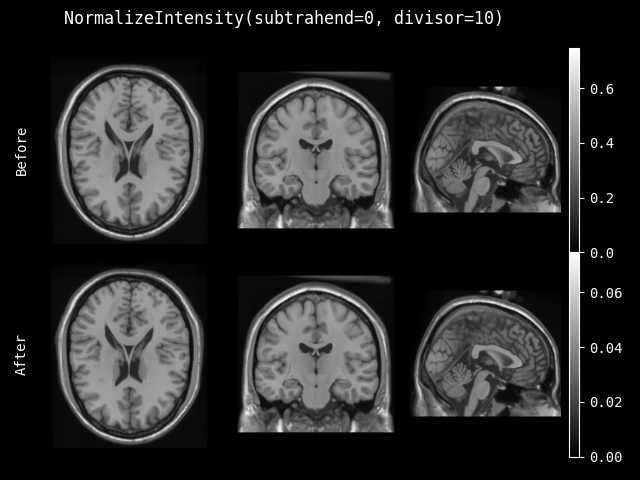class monai.transforms.NormalizeIntensity(subtrahend=None, divisor=None, nonzero=False, channel_wise=False, dtype=<class 'numpy.float32'>)[source]#

Normalize input based on the subtrahend and divisor: (img - subtrahend) / divisor. Use calculated mean or std value of the input image if no subtrahend or divisor provided. This transform can normalize only non-zero values or entire image, and can also calculate mean and std on each channel separately. When channel_wise is True, the first dimension of subtrahend and divisor should be the number of image channels if they are not None.

Parameters:
• subtrahend – the amount to subtract by (usually the mean).

• divisor – the amount to divide by (usually the standard deviation).

• nonzero – whether only normalize non-zero values.

• channel_wise – if True, calculate on each channel separately, otherwise, calculate on the entire image directly. default to False.

• dtype – output data type, if None, same as input image. defaults to float32.

__call__(img)[source]#

Apply the transform to img, assuming img is a channel-first array if self.channel_wise is True,

Return type:

`Union`[`ndarray`, `Tensor`]

#### ThresholdIntensity#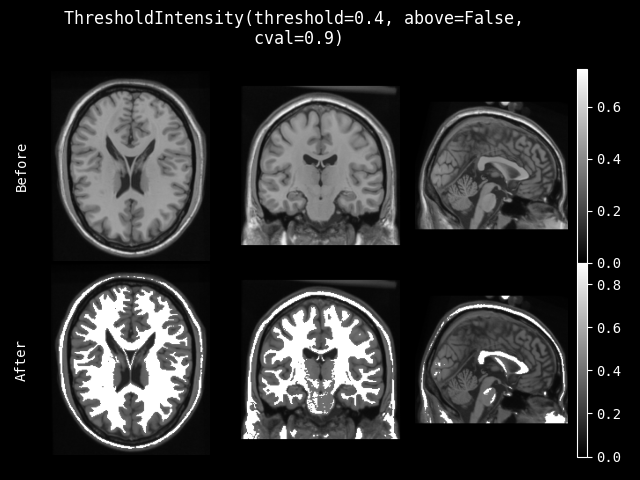class monai.transforms.ThresholdIntensity(threshold, above=True, cval=0.0)[source]#

Filter the intensity values of whole image to below threshold or above threshold. And fill the remaining parts of the image to the cval value.

Parameters:
• threshold (`float`) – the threshold to filter intensity values.

• above (`bool`) – filter values above the threshold or below the threshold, default is True.

• cval (`float`) – value to fill the remaining parts of the image, default is 0.

__call__(img)[source]#

Apply the transform to img.

Return type:

`Union`[`ndarray`, `Tensor`]

#### ScaleIntensityRange#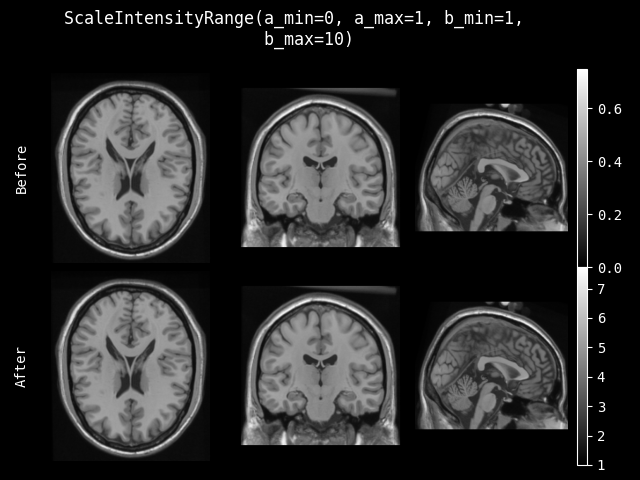class monai.transforms.ScaleIntensityRange(a_min, a_max, b_min=None, b_max=None, clip=False, dtype=<class 'numpy.float32'>)[source]#

Apply specific intensity scaling to the whole numpy array. Scaling from [a_min, a_max] to [b_min, b_max] with clip option.

When b_min or b_max are None, scaled_array * (b_max - b_min) + b_min will be skipped. If clip=True, when b_min/b_max is None, the clipping is not performed on the corresponding edge.

Parameters:
• a_min – intensity original range min.

• a_max – intensity original range max.

• b_min – intensity target range min.

• b_max – intensity target range max.

• clip – whether to perform clip after scaling.

• dtype – output data type, if None, same as input image. defaults to float32.

__call__(img)[source]#

Apply the transform to img.

Return type:

`Union`[`ndarray`, `Tensor`]

#### ScaleIntensityRangePercentiles#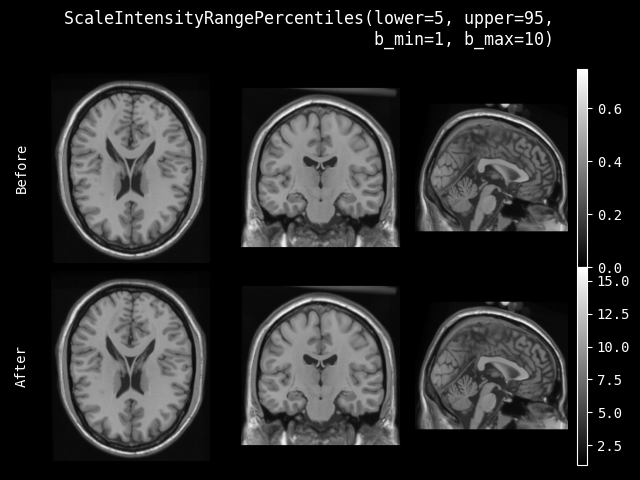class monai.transforms.ScaleIntensityRangePercentiles(lower, upper, b_min, b_max, clip=False, relative=False, channel_wise=False, dtype=<class 'numpy.float32'>)[source]#

Apply range scaling to a numpy array based on the intensity distribution of the input.

By default this transform will scale from [lower_intensity_percentile, upper_intensity_percentile] to [b_min, b_max], where {lower,upper}_intensity_percentile are the intensity values at the corresponding percentiles of `img`.

The `relative` parameter can also be set to scale from [lower_intensity_percentile, upper_intensity_percentile] to the lower and upper percentiles of the output range [b_min, b_max].

For example:

```image = torch.Tensor(
[[[1, 2, 3, 4, 5],
[1, 2, 3, 4, 5],
[1, 2, 3, 4, 5],
[1, 2, 3, 4, 5],
[1, 2, 3, 4, 5],
[1, 2, 3, 4, 5]]])

# Scale from lower and upper image intensity percentiles
# to output range [b_min, b_max]
scaler = ScaleIntensityRangePercentiles(10, 90, 0, 200, False, False)
print(scaler(image))
metatensor([[[  0.,  50., 100., 150., 200.],
[  0.,  50., 100., 150., 200.],
[  0.,  50., 100., 150., 200.],
[  0.,  50., 100., 150., 200.],
[  0.,  50., 100., 150., 200.],
[  0.,  50., 100., 150., 200.]]])

# Scale from lower and upper image intensity percentiles
# to lower and upper percentiles of the output range [b_min, b_max]
rel_scaler = ScaleIntensityRangePercentiles(10, 90, 0, 200, False, True)
print(rel_scaler(image))
metatensor([[[ 20.,  60., 100., 140., 180.],
[ 20.,  60., 100., 140., 180.],
[ 20.,  60., 100., 140., 180.],
[ 20.,  60., 100., 140., 180.],
[ 20.,  60., 100., 140., 180.],
[ 20.,  60., 100., 140., 180.]]])
```
Parameters:
• lower – lower intensity percentile.

• upper – upper intensity percentile.

• b_min – intensity target range min.

• b_max – intensity target range max.

• clip – whether to perform clip after scaling.

• relative – whether to scale to the corresponding percentiles of [b_min, b_max].

• channel_wise – if True, compute intensity percentile and normalize every channel separately. default to False.

• dtype – output data type, if None, same as input image. defaults to float32.

__call__(img)[source]#

Apply the transform to img.

Return type:

`Union`[`ndarray`, `Tensor`]Changes image intensity with gamma transform. Each pixel/voxel intensity is updated as:

```x = ((x - min) / intensity_range) ^ gamma * intensity_range + min
```
Parameters:
• gamma (`float`) – gamma value to adjust the contrast as function.

• invert_image (`bool`) – whether to invert the image before applying gamma augmentation. If True, multiply all intensity values with -1 before the gamma transform and again after the gamma transform. This behaviour is mimicked from nnU-Net, specifically this function.

• retain_stats (`bool`) –

if True, applies a scaling factor and an offset to all intensity values after gamma transform to ensure that the output intensity distribution has the same mean and standard deviation as the intensity distribution of the input. This behaviour is mimicked from nnU-Net, specifically this function.

__call__(img, gamma=None)[source]#

Apply the transform to img. gamma: gamma value to adjust the contrast as function.

Return type:

`Union`[`ndarray`, `Tensor`]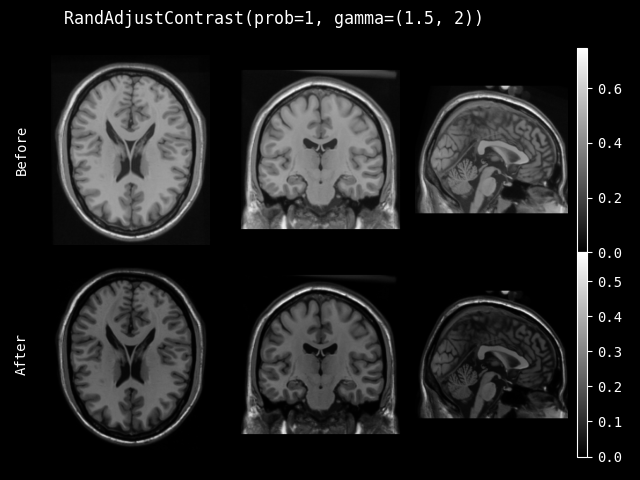class monai.transforms.RandAdjustContrast(prob=0.1, gamma=(0.5, 4.5), invert_image=False, retain_stats=False)[source]#

Randomly changes image intensity with gamma transform. Each pixel/voxel intensity is updated as:

x = ((x - min) / intensity_range) ^ gamma * intensity_range + min

Parameters:
• prob – Probability of adjustment.

• gamma – Range of gamma values. If single number, value is picked from (0.5, gamma), default is (0.5, 4.5).

• invert_image

whether to invert the image before applying gamma augmentation. If True, multiply all intensity values with -1 before the gamma transform and again after the gamma transform. This behaviour is mimicked from nnU-Net, specifically this function.

• retain_stats

if True, applies a scaling factor and an offset to all intensity values after gamma transform to ensure that the output intensity distribution has the same mean and standard deviation as the intensity distribution of the input. This behaviour is mimicked from nnU-Net, specifically this function.

__call__(img, randomize=True)[source]#

Apply the transform to img.

Return type:

`Union`[`ndarray`, `Tensor`]

randomize(data=None)[source]#

Within this method, `self.R` should be used, instead of np.random, to introduce random factors.

all `self.R` calls happen here so that we have a better chance to identify errors of sync the random state.

This method can generate the random factors based on properties of the input data.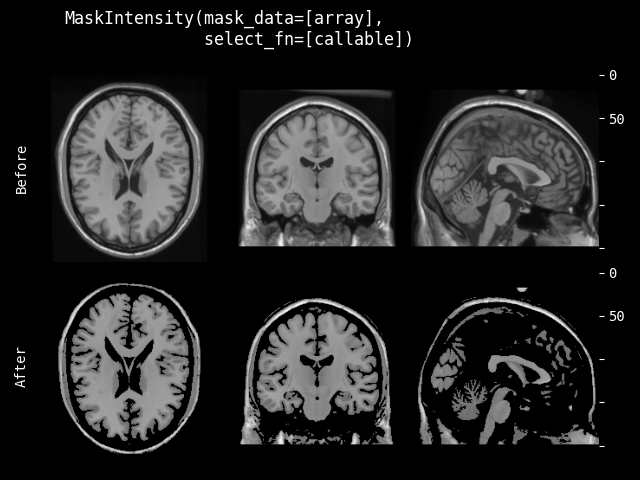Mask the intensity values of input image with the specified mask data. Mask data must have the same spatial size as the input image, and all the intensity values of input image corresponding to the selected values in the mask data will keep the original value, others will be set to 0.

Parameters:
• mask_data – if mask_data is single channel, apply to every channel of input image. if multiple channels, the number of channels must match the input data. the intensity values of input image corresponding to the selected values in the mask data will keep the original value, others will be set to 0. if None, must specify the mask_data at runtime.

• select_fn – function to select valid values of the mask_data, default is to select values > 0.

Parameters:

mask_data – if mask data is single channel, apply to every channel of input image. if multiple channels, the channel number must match input data. mask_data will be converted to bool values by mask_data > 0 before applying transform to input image.

Raises:
• - ValueError – When both `mask_data` and `self.mask_data` are None.

• - ValueError – When `mask_data` and `img` channels differ and `mask_data` is not single channel.

#### SavitzkyGolaySmooth#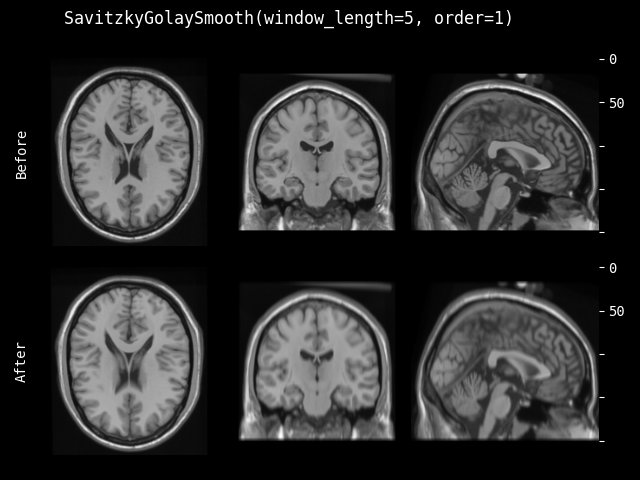class monai.transforms.SavitzkyGolaySmooth(window_length, order, axis=1, mode='zeros')[source]#

Smooth the input data along the given axis using a Savitzky-Golay filter.

Parameters:
• window_length (`int`) – Length of the filter window, must be a positive odd integer.

• order (`int`) – Order of the polynomial to fit to each window, must be less than `window_length`.

• axis (`int`) – Optional axis along which to apply the filter kernel. Default 1 (first spatial dimension).

• mode (`str`) – Optional padding mode, passed to convolution class. `'zeros'`, `'reflect'`, `'replicate'` or `'circular'`. Default: `'zeros'`. See `torch.nn.Conv1d()` for more information.

__call__(img)[source]#
Parameters:

img (`Union`[`ndarray`, `Tensor`]) – array containing input data. Must be real and in shape [channels, spatial1, spatial2, …].

Return type:

`Union`[`ndarray`, `Tensor`]

Returns:

array containing smoothed result.

#### MedianSmooth#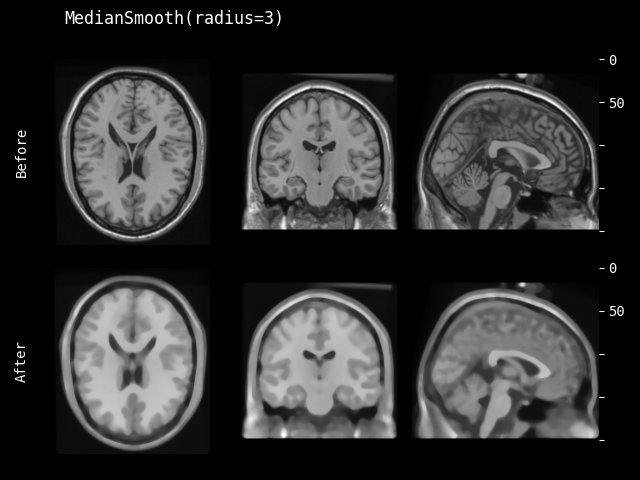Apply median filter to the input data based on specified radius parameter. A default value radius=1 is provided for reference.

Parameters:

radius – if a list of values, must match the count of spatial dimensions of input data, and apply every value in the list to 1 spatial dimension. if only 1 value provided, use it for all spatial dimensions.

__call__(img)[source]#

`data` is an element which often comes from an iteration over an iterable, such as `torch.utils.data.Dataset`. This method should return an updated version of `data`. To simplify the input validations, most of the transforms assume that

• `data` is a Numpy ndarray, PyTorch Tensor or string,

• the data shape can be:

1. string data without shape, LoadImage transform expects file paths,

2. most of the pre-/post-processing transforms expect: `(num_channels, spatial_dim_1[, spatial_dim_2, ...])`, except for example: AddChannel expects (spatial_dim_1[, spatial_dim_2, …])

• the channel dimension is often not omitted even if number of channels is one.

This method can optionally take additional arguments to help execute transformation operation.

Raises:

NotImplementedError – When the subclass does not override this method.

Return type:

~NdarrayTensor

#### GaussianSmooth#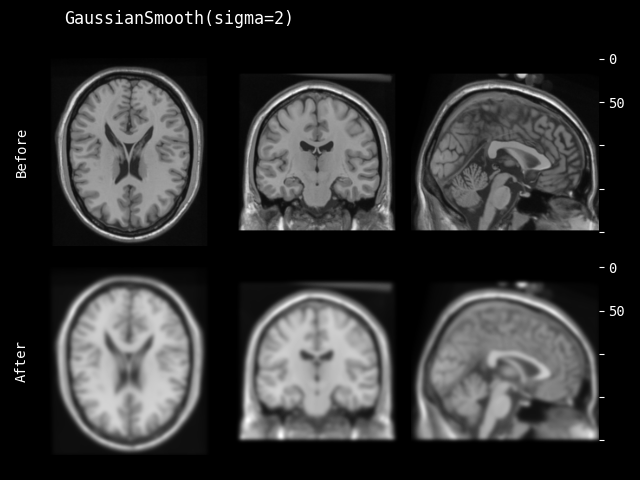class monai.transforms.GaussianSmooth(sigma=1.0, approx='erf')[source]#

Apply Gaussian smooth to the input data based on specified sigma parameter. A default value sigma=1.0 is provided for reference.

Parameters:
• sigma – if a list of values, must match the count of spatial dimensions of input data, and apply every value in the list to 1 spatial dimension. if only 1 value provided, use it for all spatial dimensions.

• approx – discrete Gaussian kernel type, available options are “erf”, “sampled”, and “scalespace”. see also `monai.networks.layers.GaussianFilter()`.

__call__(img)[source]#

`data` is an element which often comes from an iteration over an iterable, such as `torch.utils.data.Dataset`. This method should return an updated version of `data`. To simplify the input validations, most of the transforms assume that

• `data` is a Numpy ndarray, PyTorch Tensor or string,

• the data shape can be:

1. string data without shape, LoadImage transform expects file paths,

2. most of the pre-/post-processing transforms expect: `(num_channels, spatial_dim_1[, spatial_dim_2, ...])`, except for example: AddChannel expects (spatial_dim_1[, spatial_dim_2, …])

• the channel dimension is often not omitted even if number of channels is one.

This method can optionally take additional arguments to help execute transformation operation.

Raises:

NotImplementedError – When the subclass does not override this method.

Return type:

~NdarrayTensor

#### RandGaussianSmooth#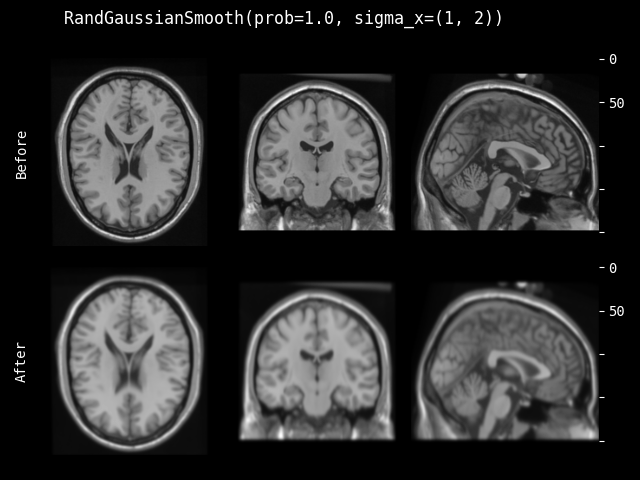class monai.transforms.RandGaussianSmooth(sigma_x=(0.25, 1.5), sigma_y=(0.25, 1.5), sigma_z=(0.25, 1.5), prob=0.1, approx='erf')[source]#

Apply Gaussian smooth to the input data based on randomly selected sigma parameters.

Parameters:
• sigma_x (`tuple`[`float`, `float`]) – randomly select sigma value for the first spatial dimension.

• sigma_y (`tuple`[`float`, `float`]) – randomly select sigma value for the second spatial dimension if have.

• sigma_z (`tuple`[`float`, `float`]) – randomly select sigma value for the third spatial dimension if have.

• prob (`float`) – probability of Gaussian smooth.

• approx (`str`) – discrete Gaussian kernel type, available options are “erf”, “sampled”, and “scalespace”. see also `monai.networks.layers.GaussianFilter()`.

__call__(img, randomize=True)[source]#

`data` is an element which often comes from an iteration over an iterable, such as `torch.utils.data.Dataset`. This method should return an updated version of `data`. To simplify the input validations, most of the transforms assume that

• `data` is a Numpy ndarray, PyTorch Tensor or string,

• the data shape can be:

1. string data without shape, LoadImage transform expects file paths,

2. most of the pre-/post-processing transforms expect: `(num_channels, spatial_dim_1[, spatial_dim_2, ...])`, except for example: AddChannel expects (spatial_dim_1[, spatial_dim_2, …])

• the channel dimension is often not omitted even if number of channels is one.

This method can optionally take additional arguments to help execute transformation operation.

Raises:

NotImplementedError – When the subclass does not override this method.

Return type:

`Union`[`ndarray`, `Tensor`]

randomize(data=None)[source]#

Within this method, `self.R` should be used, instead of np.random, to introduce random factors.

all `self.R` calls happen here so that we have a better chance to identify errors of sync the random state.

This method can generate the random factors based on properties of the input data.

#### GaussianSharpen#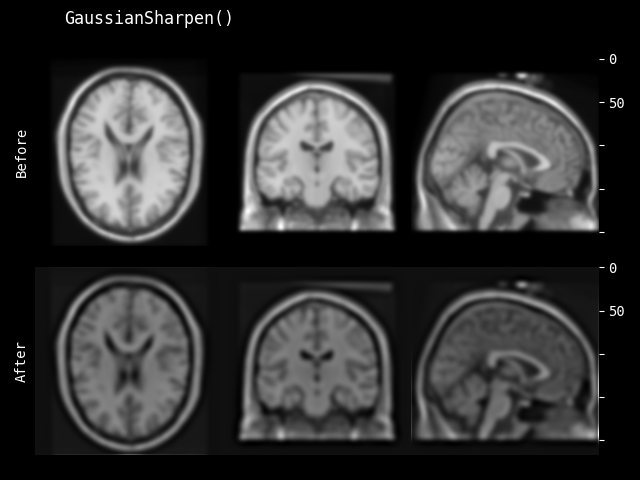class monai.transforms.GaussianSharpen(sigma1=3.0, sigma2=1.0, alpha=30.0, approx='erf')[source]#

Sharpen images using the Gaussian Blur filter. Referring to: http://scipy-lectures.org/advanced/image_processing/auto_examples/plot_sharpen.html. The algorithm is shown as below

```blurred_f = gaussian_filter(img, sigma1)
filter_blurred_f = gaussian_filter(blurred_f, sigma2)
img = blurred_f + alpha * (blurred_f - filter_blurred_f)
```

A set of default values sigma1=3.0, sigma2=1.0 and alpha=30.0 is provide for reference.

Parameters:
• sigma1 – sigma parameter for the first gaussian kernel. if a list of values, must match the count of spatial dimensions of input data, and apply every value in the list to 1 spatial dimension. if only 1 value provided, use it for all spatial dimensions.

• sigma2 – sigma parameter for the second gaussian kernel. if a list of values, must match the count of spatial dimensions of input data, and apply every value in the list to 1 spatial dimension. if only 1 value provided, use it for all spatial dimensions.

• alpha – weight parameter to compute the final result.

• approx – discrete Gaussian kernel type, available options are “erf”, “sampled”, and “scalespace”. see also `monai.networks.layers.GaussianFilter()`.

__call__(img)[source]#

`data` is an element which often comes from an iteration over an iterable, such as `torch.utils.data.Dataset`. This method should return an updated version of `data`. To simplify the input validations, most of the transforms assume that

• `data` is a Numpy ndarray, PyTorch Tensor or string,

• the data shape can be:

1. string data without shape, LoadImage transform expects file paths,

2. most of the pre-/post-processing transforms expect: `(num_channels, spatial_dim_1[, spatial_dim_2, ...])`, except for example: AddChannel expects (spatial_dim_1[, spatial_dim_2, …])

• the channel dimension is often not omitted even if number of channels is one.

This method can optionally take additional arguments to help execute transformation operation.

Raises:

NotImplementedError – When the subclass does not override this method.

Return type:

~NdarrayTensor

#### RandGaussianSharpen#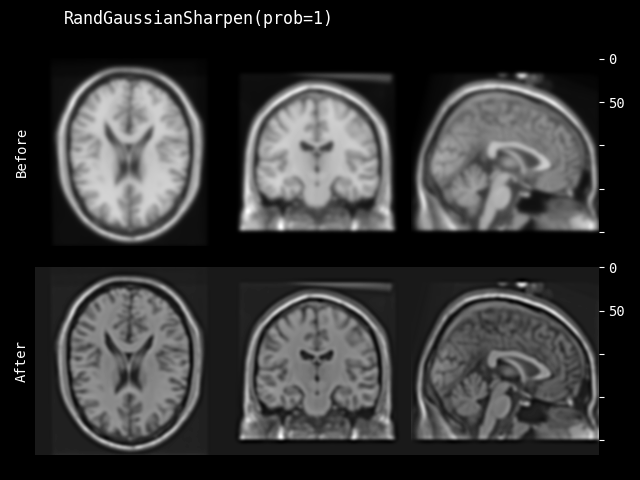class monai.transforms.RandGaussianSharpen(sigma1_x=(0.5, 1.0), sigma1_y=(0.5, 1.0), sigma1_z=(0.5, 1.0), sigma2_x=0.5, sigma2_y=0.5, sigma2_z=0.5, alpha=(10.0, 30.0), approx='erf', prob=0.1)[source]#

Sharpen images using the Gaussian Blur filter based on randomly selected sigma1, sigma2 and alpha. The algorithm is `monai.transforms.GaussianSharpen`.

Parameters:
• sigma1_x – randomly select sigma value for the first spatial dimension of first gaussian kernel.

• sigma1_y – randomly select sigma value for the second spatial dimension(if have) of first gaussian kernel.

• sigma1_z – randomly select sigma value for the third spatial dimension(if have) of first gaussian kernel.

• sigma2_x – randomly select sigma value for the first spatial dimension of second gaussian kernel. if only 1 value X provided, it must be smaller than sigma1_x and randomly select from [X, sigma1_x].

• sigma2_y – randomly select sigma value for the second spatial dimension(if have) of second gaussian kernel. if only 1 value Y provided, it must be smaller than sigma1_y and randomly select from [Y, sigma1_y].

• sigma2_z – randomly select sigma value for the third spatial dimension(if have) of second gaussian kernel. if only 1 value Z provided, it must be smaller than sigma1_z and randomly select from [Z, sigma1_z].

• alpha – randomly select weight parameter to compute the final result.

• approx – discrete Gaussian kernel type, available options are “erf”, “sampled”, and “scalespace”. see also `monai.networks.layers.GaussianFilter()`.

• prob – probability of Gaussian sharpen.

__call__(img, randomize=True)[source]#

`data` is an element which often comes from an iteration over an iterable, such as `torch.utils.data.Dataset`. This method should return an updated version of `data`. To simplify the input validations, most of the transforms assume that

• `data` is a Numpy ndarray, PyTorch Tensor or string,

• the data shape can be:

1. string data without shape, LoadImage transform expects file paths,

2. most of the pre-/post-processing transforms expect: `(num_channels, spatial_dim_1[, spatial_dim_2, ...])`, except for example: AddChannel expects (spatial_dim_1[, spatial_dim_2, …])

• the channel dimension is often not omitted even if number of channels is one.

This method can optionally take additional arguments to help execute transformation operation.

Raises:

NotImplementedError – When the subclass does not override this method.

Return type:

`Union`[`ndarray`, `Tensor`]

randomize(data=None)[source]#

Within this method, `self.R` should be used, instead of np.random, to introduce random factors.

all `self.R` calls happen here so that we have a better chance to identify errors of sync the random state.

This method can generate the random factors based on properties of the input data.

#### RandHistogramShift#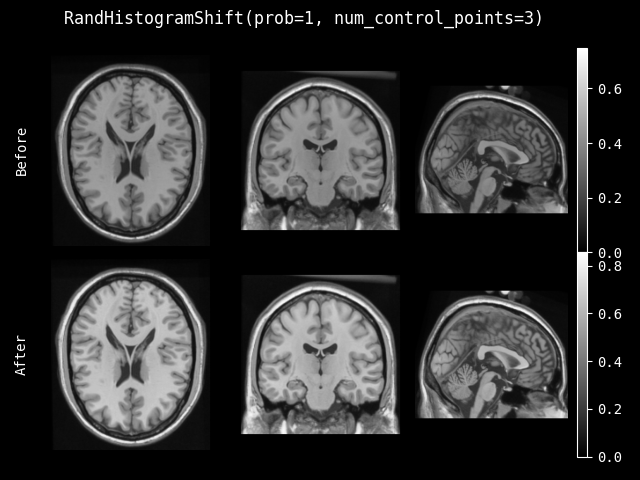class monai.transforms.RandHistogramShift(num_control_points=10, prob=0.1)[source]#

Apply random nonlinear transform to the image’s intensity histogram.

Parameters:
• num_control_points – number of control points governing the nonlinear intensity mapping. a smaller number of control points allows for larger intensity shifts. if two values provided, number of control points selecting from range (min_value, max_value).

• prob – probability of histogram shift.

__call__(img, randomize=True)[source]#

`data` is an element which often comes from an iteration over an iterable, such as `torch.utils.data.Dataset`. This method should return an updated version of `data`. To simplify the input validations, most of the transforms assume that

• `data` is a Numpy ndarray, PyTorch Tensor or string,

• the data shape can be:

1. string data without shape, LoadImage transform expects file paths,

2. most of the pre-/post-processing transforms expect: `(num_channels, spatial_dim_1[, spatial_dim_2, ...])`, except for example: AddChannel expects (spatial_dim_1[, spatial_dim_2, …])

• the channel dimension is often not omitted even if number of channels is one.

This method can optionally take additional arguments to help execute transformation operation.

Raises:

NotImplementedError – When the subclass does not override this method.

Return type:

`Union`[`ndarray`, `Tensor`]

randomize(data=None)[source]#

Within this method, `self.R` should be used, instead of np.random, to introduce random factors.

all `self.R` calls happen here so that we have a better chance to identify errors of sync the random state.

This method can generate the random factors based on properties of the input data.

#### DetectEnvelope#

class monai.transforms.DetectEnvelope(axis=1, n=None)[source]#

Find the envelope of the input data along the requested axis using a Hilbert transform.

Parameters:
• axis – Axis along which to detect the envelope. Default 1, i.e. the first spatial dimension.

• n – FFT size. Default img.shape[axis]. Input will be zero-padded or truncated to this size along dimension

• axis.

__call__(img)[source]#
Parameters:

img (`Union`[`ndarray`, `Tensor`]) – numpy.ndarray containing input data. Must be real and in shape [channels, spatial1, spatial2, …].

Returns:

np.ndarray containing envelope of data in img along the specified axis.

#### GibbsNoise#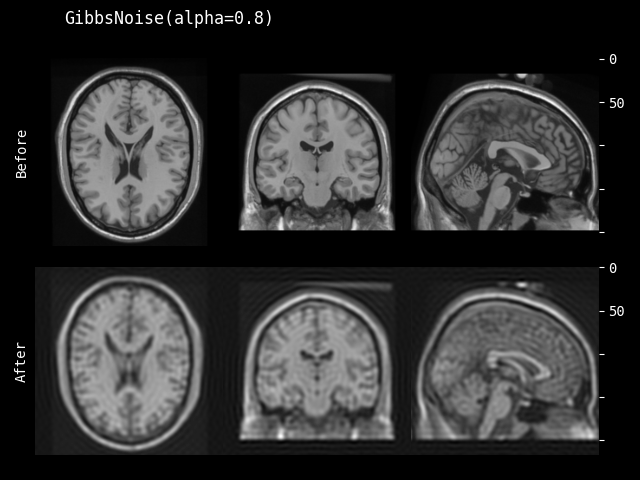class monai.transforms.GibbsNoise(alpha=0.1)[source]#

The transform applies Gibbs noise to 2D/3D MRI images. Gibbs artifacts are one of the common type of type artifacts appearing in MRI scans.

The transform is applied to all the channels in the data.

For general information on Gibbs artifacts, please refer to:

The AAPM/RSNA Physics Tutorial for Residents

Parameters:

alpha (`float`) – Parametrizes the intensity of the Gibbs noise filter applied. Takes values in the interval [0,1] with alpha = 0 acting as the identity mapping.

__call__(img)[source]#

`data` is an element which often comes from an iteration over an iterable, such as `torch.utils.data.Dataset`. This method should return an updated version of `data`. To simplify the input validations, most of the transforms assume that

• `data` is a Numpy ndarray, PyTorch Tensor or string,

• the data shape can be:

1. string data without shape, LoadImage transform expects file paths,

2. most of the pre-/post-processing transforms expect: `(num_channels, spatial_dim_1[, spatial_dim_2, ...])`, except for example: AddChannel expects (spatial_dim_1[, spatial_dim_2, …])

• the channel dimension is often not omitted even if number of channels is one.

This method can optionally take additional arguments to help execute transformation operation.

Raises:

NotImplementedError – When the subclass does not override this method.

Return type:

`Union`[`ndarray`, `Tensor`]

#### RandGibbsNoise#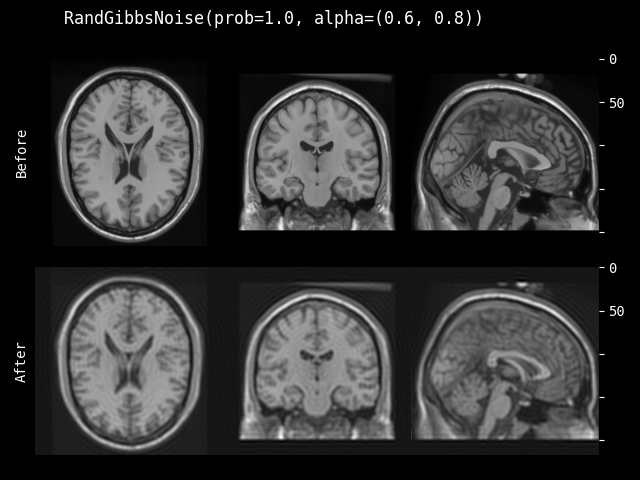class monai.transforms.RandGibbsNoise(prob=0.1, alpha=(0.0, 1.0))[source]#

Naturalistic image augmentation via Gibbs artifacts. The transform randomly applies Gibbs noise to 2D/3D MRI images. Gibbs artifacts are one of the common type of type artifacts appearing in MRI scans.

The transform is applied to all the channels in the data.

Parameters:
• prob (float) – probability of applying the transform.

• alpha (Sequence(float)) – Parametrizes the intensity of the Gibbs noise filter applied. Takes values in the interval [0,1] with alpha = 0 acting as the identity mapping. If a length-2 list is given as [a,b] then the value of alpha will be sampled uniformly from the interval [a,b]. 0 <= a <= b <= 1.

__call__(img, randomize=True)[source]#

`data` is an element which often comes from an iteration over an iterable, such as `torch.utils.data.Dataset`. This method should return an updated version of `data`. To simplify the input validations, most of the transforms assume that

• `data` is a Numpy ndarray, PyTorch Tensor or string,

• the data shape can be:

1. string data without shape, LoadImage transform expects file paths,

2. most of the pre-/post-processing transforms expect: `(num_channels, spatial_dim_1[, spatial_dim_2, ...])`, except for example: AddChannel expects (spatial_dim_1[, spatial_dim_2, …])

• the channel dimension is often not omitted even if number of channels is one.

This method can optionally take additional arguments to help execute transformation operation.

Raises:

NotImplementedError – When the subclass does not override this method.

randomize(data)[source]#
1. Set random variable to apply the transform.

2. Get alpha from uniform distribution.

Return type:

`None`

#### KSpaceSpikeNoise#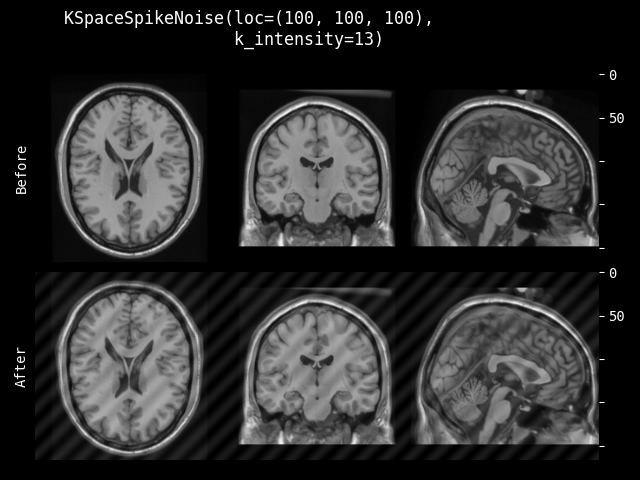class monai.transforms.KSpaceSpikeNoise(loc, k_intensity=None)[source]#

Apply localized spikes in k-space at the given locations and intensities. Spike (Herringbone) artifact is a type of data acquisition artifact which may occur during MRI scans.

For general information on spike artifacts, please refer to:

Parameters:
• loc – spatial location for the spikes. For images with 3D spatial dimensions, the user can provide (C, X, Y, Z) to fix which channel C is affected, or (X, Y, Z) to place the same spike in all channels. For 2D cases, the user can provide (C, X, Y) or (X, Y).

• k_intensity – value for the log-intensity of the k-space version of the image. If one location is passed to `loc` or the channel is not specified, then this argument should receive a float. If `loc` is given a sequence of locations, then this argument should receive a sequence of intensities. This value should be tested as it is data-dependent. The default values are the 2.5 the mean of the log-intensity for each channel.

Example

When working with 4D data, `KSpaceSpikeNoise(loc = ((3,60,64,32), (64,60,32)), k_intensity = (13,14))` will place a spike at [3, 60, 64, 32] with log-intensity = 13, and one spike per channel located respectively at [: , 64, 60, 32] with log-intensity = 14.

__call__(img)[source]#
Parameters:

img (`Union`[`ndarray`, `Tensor`]) – image with dimensions (C, H, W) or (C, H, W, D)

Return type:

`Union`[`ndarray`, `Tensor`]

#### RandKSpaceSpikeNoise#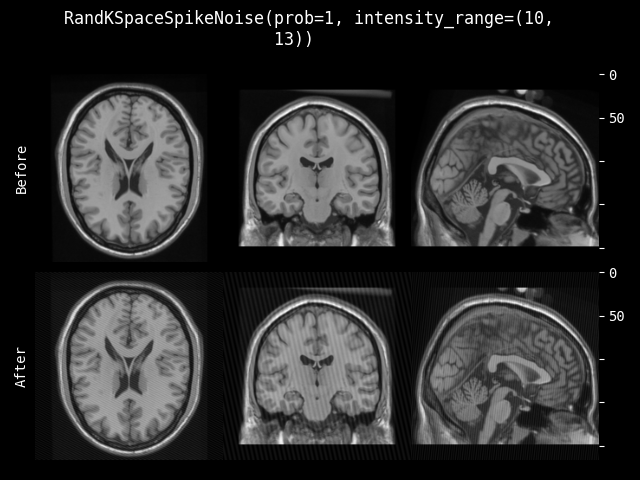class monai.transforms.RandKSpaceSpikeNoise(prob=0.1, intensity_range=None, channel_wise=True)[source]#

Naturalistic data augmentation via spike artifacts. The transform applies localized spikes in k-space, and it is the random version of `monai.transforms.KSpaceSpikeNoise`.

Spike (Herringbone) artifact is a type of data acquisition artifact which may occur during MRI scans. For general information on spike artifacts, please refer to:

Parameters:
• prob – probability of applying the transform, either on all channels at once, or channel-wise if `channel_wise = True`.

• intensity_range – pass a tuple (a, b) to sample the log-intensity from the interval (a, b) uniformly for all channels. Or pass sequence of intervals ((a0, b0), (a1, b1), …) to sample for each respective channel. In the second case, the number of 2-tuples must match the number of channels. Default ranges is (0.95x, 1.10x) where x is the mean log-intensity for each channel.

• channel_wise – treat each channel independently. True by default.

Example

To apply k-space spikes randomly with probability 0.5, and log-intensity sampled from the interval [11, 12] for each channel independently, one uses `RandKSpaceSpikeNoise(prob=0.5, intensity_range=(11, 12), channel_wise=True)`

__call__(img, randomize=True)[source]#

Apply transform to img. Assumes data is in channel-first form.

Parameters:

img (`Union`[`ndarray`, `Tensor`]) – image with dimensions (C, H, W) or (C, H, W, D)

randomize(img, intensity_range)[source]#

Helper method to sample both the location and intensity of the spikes. When not working channel wise (channel_wise=False) it use the random variable `self._do_transform` to decide whether to sample a location and intensity.

When working channel wise, the method randomly samples a location and intensity for each channel depending on `self._do_transform`.

Return type:

`None`

#### RandRicianNoise#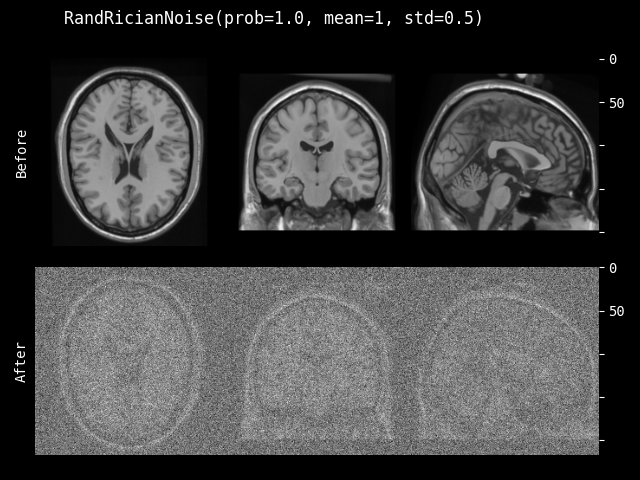class monai.transforms.RandRicianNoise(prob=0.1, mean=0.0, std=1.0, channel_wise=False, relative=False, sample_std=True, dtype=<class 'numpy.float32'>)[source]#

Add Rician noise to image. Rician noise in MRI is the result of performing a magnitude operation on complex data with Gaussian noise of the same variance in both channels, as described in Noise in Magnitude Magnetic Resonance Images. This transform is adapted from DIPY. See also: The rician distribution of noisy mri data.

Parameters:
• prob – Probability to add Rician noise.

• mean – Mean or “centre” of the Gaussian distributions sampled to make up the Rician noise.

• std – Standard deviation (spread) of the Gaussian distributions sampled to make up the Rician noise.

• channel_wise – If True, treats each channel of the image separately.

• relative – If True, the spread of the sampled Gaussian distributions will be std times the standard deviation of the image or channel’s intensity histogram.

• sample_std – If True, sample the spread of the Gaussian distributions uniformly from 0 to std.

• dtype – output data type, if None, same as input image. defaults to float32.

__call__(img, randomize=True)[source]#

Apply the transform to img.

Return type:

`Union`[`ndarray`, `Tensor`]

#### RandCoarseTransform#

class monai.transforms.RandCoarseTransform(holes, spatial_size, max_holes=None, max_spatial_size=None, prob=0.1)[source]#

Randomly select coarse regions in the image, then execute transform operations for the regions. It’s the base class of all kinds of region transforms. Refer to papers: https://arxiv.org/abs/1708.04552

Parameters:
• holes – number of regions to dropout, if max_holes is not None, use this arg as the minimum number to randomly select the expected number of regions.

• spatial_size – spatial size of the regions to dropout, if max_spatial_size is not None, use this arg as the minimum spatial size to randomly select size for every region. if some components of the spatial_size are non-positive values, the transform will use the corresponding components of input img size. For example, spatial_size=(32, -1) will be adapted to (32, 64) if the second spatial dimension size of img is 64.

• max_holes – if not None, define the maximum number to randomly select the expected number of regions.

• max_spatial_size – if not None, define the maximum spatial size to randomly select size for every region. if some components of the max_spatial_size are non-positive values, the transform will use the corresponding components of input img size. For example, max_spatial_size=(32, -1) will be adapted to (32, 64) if the second spatial dimension size of img is 64.

• prob – probability of applying the transform.

__call__(img, randomize=True)[source]#

`data` is an element which often comes from an iteration over an iterable, such as `torch.utils.data.Dataset`. This method should return an updated version of `data`. To simplify the input validations, most of the transforms assume that

• `data` is a Numpy ndarray, PyTorch Tensor or string,

• the data shape can be:

1. string data without shape, LoadImage transform expects file paths,

2. most of the pre-/post-processing transforms expect: `(num_channels, spatial_dim_1[, spatial_dim_2, ...])`, except for example: AddChannel expects (spatial_dim_1[, spatial_dim_2, …])

• the channel dimension is often not omitted even if number of channels is one.

This method can optionally take additional arguments to help execute transformation operation.

Raises:

NotImplementedError – When the subclass does not override this method.

Return type:

`Union`[`ndarray`, `Tensor`]

randomize(img_size)[source]#

Within this method, `self.R` should be used, instead of np.random, to introduce random factors.

all `self.R` calls happen here so that we have a better chance to identify errors of sync the random state.

This method can generate the random factors based on properties of the input data.

Return type:

`None`

#### RandCoarseDropout#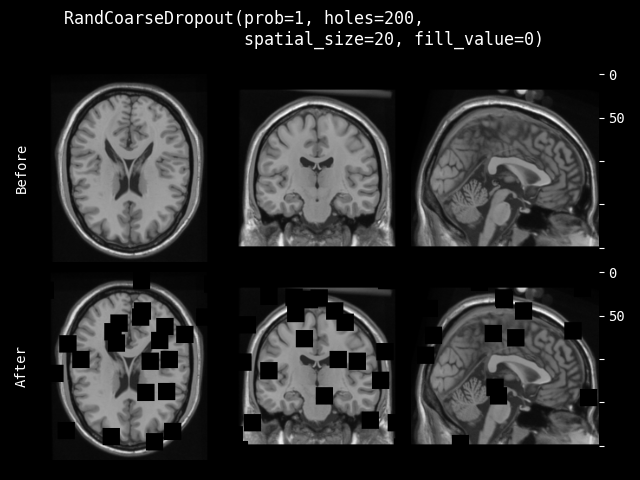class monai.transforms.RandCoarseDropout(holes, spatial_size, dropout_holes=True, fill_value=None, max_holes=None, max_spatial_size=None, prob=0.1)[source]#

Randomly coarse dropout regions in the image, then fill in the rectangular regions with specified value. Or keep the rectangular regions and fill in the other areas with specified value. Refer to papers: https://arxiv.org/abs/1708.04552, https://arxiv.org/pdf/1604.07379 And other implementation: https://albumentations.ai/docs/api_reference/augmentations/transforms/ #albumentations.augmentations.transforms.CoarseDropout.

Parameters:
• holes – number of regions to dropout, if max_holes is not None, use this arg as the minimum number to randomly select the expected number of regions.

• spatial_size – spatial size of the regions to dropout, if max_spatial_size is not None, use this arg as the minimum spatial size to randomly select size for every region. if some components of the spatial_size are non-positive values, the transform will use the corresponding components of input img size. For example, spatial_size=(32, -1) will be adapted to (32, 64) if the second spatial dimension size of img is 64.

• dropout_holes – if True, dropout the regions of holes and fill value, if False, keep the holes and dropout the outside and fill value. default to True.

• fill_value – target value to fill the dropout regions, if providing a number, will use it as constant value to fill all the regions. if providing a tuple for the min and max, will randomly select value for every pixel / voxel from the range [min, max). if None, will compute the min and max value of input image then randomly select value to fill, default to None.

• max_holes – if not None, define the maximum number to randomly select the expected number of regions.

• max_spatial_size – if not None, define the maximum spatial size to randomly select size for every region. if some components of the max_spatial_size are non-positive values, the transform will use the corresponding components of input img size. For example, max_spatial_size=(32, -1) will be adapted to (32, 64) if the second spatial dimension size of img is 64.

• prob – probability of applying the transform.

#### RandCoarseShuffle#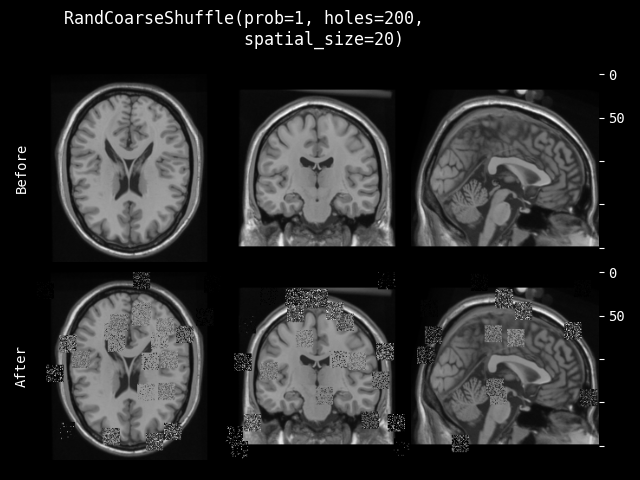class monai.transforms.RandCoarseShuffle(holes, spatial_size, max_holes=None, max_spatial_size=None, prob=0.1)[source]#

Randomly select regions in the image, then shuffle the pixels within every region. It shuffles every channel separately. Refer to paper: Kang, Guoliang, et al. “Patchshuffle regularization.” arXiv preprint arXiv:1707.07103 (2017). https://arxiv.org/abs/1707.07103

Parameters:
• holes – number of regions to dropout, if max_holes is not None, use this arg as the minimum number to randomly select the expected number of regions.

• spatial_size – spatial size of the regions to dropout, if max_spatial_size is not None, use this arg as the minimum spatial size to randomly select size for every region. if some components of the spatial_size are non-positive values, the transform will use the corresponding components of input img size. For example, spatial_size=(32, -1) will be adapted to (32, 64) if the second spatial dimension size of img is 64.

• max_holes – if not None, define the maximum number to randomly select the expected number of regions.

• max_spatial_size – if not None, define the maximum spatial size to randomly select size for every region. if some components of the max_spatial_size are non-positive values, the transform will use the corresponding components of input img size. For example, max_spatial_size=(32, -1) will be adapted to (32, 64) if the second spatial dimension size of img is 64.

• prob – probability of applying the transform.

#### HistogramNormalize#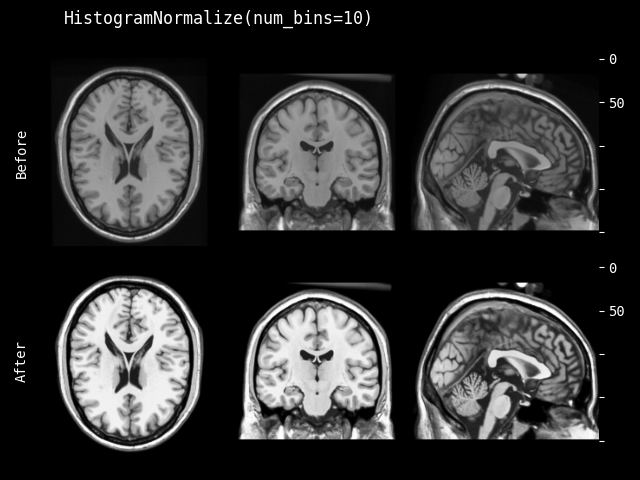class monai.transforms.HistogramNormalize(num_bins=256, min=0, max=255, mask=None, dtype=<class 'numpy.float32'>)[source]#

Apply the histogram normalization to input image. Refer to: facebookresearch/CovidPrognosis.

Parameters:
• num_bins – number of the bins to use in histogram, default to 256. for more details: https://numpy.org/doc/stable/reference/generated/numpy.histogram.html.

• min – the min value to normalize input image, default to 0.

• max – the max value to normalize input image, default to 255.

• mask – if provided, must be ndarray of bools or 0s and 1s, and same shape as image. only points at which mask==True are used for the equalization. can also provide the mask along with img at runtime.

• dtype – data type of the output, if None, same as input image. default to float32.

`data` is an element which often comes from an iteration over an iterable, such as `torch.utils.data.Dataset`. This method should return an updated version of `data`. To simplify the input validations, most of the transforms assume that

• `data` is a Numpy ndarray, PyTorch Tensor or string,

• the data shape can be:

1. string data without shape, LoadImage transform expects file paths,

2. most of the pre-/post-processing transforms expect: `(num_channels, spatial_dim_1[, spatial_dim_2, ...])`, except for example: AddChannel expects (spatial_dim_1[, spatial_dim_2, …])

• the channel dimension is often not omitted even if number of channels is one.

This method can optionally take additional arguments to help execute transformation operation.

Raises:

NotImplementedError – When the subclass does not override this method.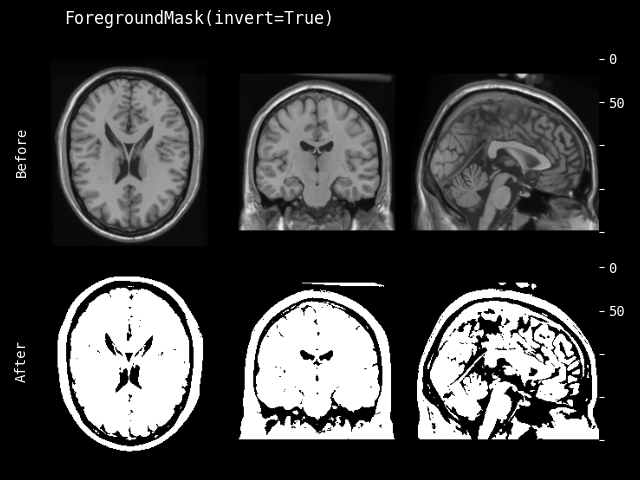Creates a binary mask that defines the foreground based on thresholds in RGB or HSV color space. This transform receives an RGB (or grayscale) image where by default it is assumed that the foreground has low values (dark) while the background has high values (white). Otherwise, set invert argument to True.

Parameters:
• threshold – an int or a float number that defines the threshold that values less than that are foreground. It also can be a callable that receives each dimension of the image and calculate the threshold, or a string that defines such callable from skimage.filter.threshold_…. For the list of available threshold functions, please refer to https://scikit-image.org/docs/stable/api/skimage.filters.html Moreover, a dictionary can be passed that defines such thresholds for each channel, like {“R”: 100, “G”: “otsu”, “B”: skimage.filter.threshold_mean}

• hsv_threshold – similar to threshold but HSV color space (“H”, “S”, and “V”). Unlike RBG, in HSV, value greater than hsv_threshold are considered foreground.

• invert – invert the intensity range of the input image, so that the dtype maximum is now the dtype minimum, and vice-versa.

__call__(image)[source]#

`data` is an element which often comes from an iteration over an iterable, such as `torch.utils.data.Dataset`. This method should return an updated version of `data`. To simplify the input validations, most of the transforms assume that

• `data` is a Numpy ndarray, PyTorch Tensor or string,

• the data shape can be:

1. string data without shape, LoadImage transform expects file paths,

2. most of the pre-/post-processing transforms expect: `(num_channels, spatial_dim_1[, spatial_dim_2, ...])`, except for example: AddChannel expects (spatial_dim_1[, spatial_dim_2, …])

• the channel dimension is often not omitted even if number of channels is one.

This method can optionally take additional arguments to help execute transformation operation.

Raises:

NotImplementedError – When the subclass does not override this method.

#### ComputeHoVerMaps#

class monai.transforms.ComputeHoVerMaps(dtype='float32')[source]#

Compute horizontal and vertical maps from an instance mask It generates normalized horizontal and vertical distances to the center of mass of each region. Input data with the size of [1xHxW[xD]], which channel dim will temporarily removed for calculating coordinates.

Parameters:

dtype (`Union`[`dtype`, `type`, `str`, `None`]) – the data type of output Tensor. Defaults to “float32”.

Returns:

A torch.Tensor with the size of [2xHxW[xD]], which is stack horizontal and vertical maps

`data` is an element which often comes from an iteration over an iterable, such as `torch.utils.data.Dataset`. This method should return an updated version of `data`. To simplify the input validations, most of the transforms assume that

• `data` is a Numpy ndarray, PyTorch Tensor or string,

• the data shape can be:

1. string data without shape, LoadImage transform expects file paths,

2. most of the pre-/post-processing transforms expect: `(num_channels, spatial_dim_1[, spatial_dim_2, ...])`, except for example: AddChannel expects (spatial_dim_1[, spatial_dim_2, …])

• the channel dimension is often not omitted even if number of channels is one.

This method can optionally take additional arguments to help execute transformation operation.

Raises:

NotImplementedError – When the subclass does not override this method.

### IO#

class monai.transforms.LoadImage(reader=None, image_only=True, dtype=<class 'numpy.float32'>, ensure_channel_first=False, simple_keys=False, prune_meta_pattern=None, prune_meta_sep='.', expanduser=True, *args, **kwargs)[source]#

Load image file or files from provided path based on reader. If reader is not specified, this class automatically chooses readers based on the supported suffixes and in the following order:

• Readers from the last to the first in the registered list.

Please note that for png, jpg, bmp, and other 2D formats, readers by default swap axis 0 and 1 after loading the array with `reverse_indexing` set to `True` because the spatial axes definition for non-medical specific file formats is different from other common medical packages.

Parameters:
• filename – path file or file-like object or a list of files. will save the filename to meta_data with key filename_or_obj. if provided a list of files, use the filename of first file to save, and will stack them together as multi-channels data. if provided directory path instead of file path, will treat it as DICOM images series and read.

__init__(reader=None, image_only=True, dtype=<class 'numpy.float32'>, ensure_channel_first=False, simple_keys=False, prune_meta_pattern=None, prune_meta_sep='.', expanduser=True, *args, **kwargs)[source]#
Parameters:
• reader – reader to load image file and metadata - if reader is None, a default set of SUPPORTED_READERS will be used. - if reader is a string, it’s treated as a class name or dotted path (such as `"monai.data.ITKReader"`), the supported built-in reader classes are `"ITKReader"`, `"NibabelReader"`, `"NumpyReader"`, `"PydicomReader"`. a reader instance will be constructed with the *args and **kwargs parameters. - if reader is a reader class/instance, it will be registered to this loader accordingly.

• image_only – if True return only the image MetaTensor, otherwise return image and header dict.

• dtype – if not None convert the loaded image to this data type.

• ensure_channel_first – if True and loaded both image array and metadata, automatically convert the image array shape to channel first. default to False.

• simple_keys – whether to remove redundant metadata keys, default to False for backward compatibility.

• prune_meta_pattern – combined with prune_meta_sep, a regular expression used to match and prune keys in the metadata (nested dictionary), default to None, no key deletion.

• prune_meta_sep – combined with prune_meta_pattern, used to match and prune keys in the metadata (nested dictionary). default is “.”, see also `monai.transforms.DeleteItemsd`. e.g. `prune_meta_pattern=".*_code\$", prune_meta_sep=" "` removes meta keys that ends with `"_code"`.

• expanduser – if True cast filename to Path and call .expanduser on it, otherwise keep filename as is.

Note

• The transform returns a MetaTensor, unless set_track_meta(False) has been used, in which case, a torch.Tensor will be returned.

• If reader is specified, the loader will attempt to use the specified readers and the default supported readers. This might introduce overheads when handling the exceptions of trying the incompatible loaders. In this case, it is therefore recommended setting the most appropriate reader as the last item of the reader parameter.

Parameters:

reader (`ImageReader`) – reader instance to be registered with this loader.

#### SaveImage#

class monai.transforms.SaveImage(output_dir='./', output_postfix='trans', output_ext='.nii.gz', output_dtype=<class 'numpy.float32'>, resample=False, mode='nearest', padding_mode=GridSamplePadMode.BORDER, scale=None, dtype=<class 'numpy.float64'>, squeeze_end_dims=True, data_root_dir='', separate_folder=True, print_log=True, output_format='', writer=None, channel_dim=0, output_name_formatter=None, folder_layout=None, savepath_in_metadict=False)[source]#

Save the image (in the form of torch tensor or numpy ndarray) and metadata dictionary into files.

The name of saved file will be {input_image_name}_{output_postfix}{output_ext}, where the input_image_name is extracted from the provided metadata dictionary. If no metadata provided, a running index starting from 0 will be used as the filename prefix.

Parameters:
• output_dir – output image directory.

• instead (Handled by folder_layout) –

• None. (if folder_layout is not) –

• output_postfix – a string appended to all output file names, default to trans.

• None.

• output_ext – output file extension name.

• None.

• output_dtype – data type (if not None) for saving data. Defaults to `np.float32`.

• resample – whether to resample image (if needed) before saving the data array, based on the `"spatial_shape"` (and `"original_affine"`) from metadata.

• mode

This option is used when `resample=True`. Defaults to `"nearest"`. Depending on the writers, the possible options are

• padding_mode – This option is used when `resample = True`. Defaults to `"border"`. Possible options are {`"zeros"`, `"border"`, `"reflection"`} See also: https://pytorch.org/docs/stable/nn.functional.html#grid-sample

• scale – {`255`, `65535`} postprocess data by clipping to [0, 1] and scaling [0, 255] (`uint8`) or [0, 65535] (`uint16`). Default is `None` (no scaling).

• dtype – data type during resampling computation. Defaults to `np.float64` for best precision. if `None`, use the data type of input data. To set the output data type, use `output_dtype`.

• squeeze_end_dims – if `True`, any trailing singleton dimensions will be removed (after the channel has been moved to the end). So if input is (C,H,W,D), this will be altered to (H,W,D,C), and then if C==1, it will be saved as (H,W,D). If D is also 1, it will be saved as (H,W). If `False`, image will always be saved as (H,W,D,C).

• data_root_dir

if not empty, it specifies the beginning parts of the input file’s absolute path. It’s used to compute `input_file_rel_path`, the relative path to the file from `data_root_dir` to preserve folder structure when saving in case there are files in different folders with the same file names. For example, with the following inputs:

• input_file_name: `/foo/bar/test1/image.nii`

• output_postfix: `seg`

• output_ext: `.nii.gz`

• output_dir: `/output`

• data_root_dir: `/foo/bar`

The output will be: `/output/test1/image/image_seg.nii.gz`

Handled by `folder_layout` instead, if `folder_layout` is not `None`.

• separate_folder – whether to save every file in a separate folder. For example: for the input filename `image.nii`, postfix `seg` and `folder_path` `output`, if `separate_folder=True`, it will be saved as: `output/image/image_seg.nii`, if `False`, saving as `output/image_seg.nii`. Default to `True`. Handled by `folder_layout` instead, if `folder_layout` is not `None`.

• print_log – whether to print logs when saving. Default to `True`.

• output_format – an optional string of filename extension to specify the output image writer. see also: `monai.data.image_writer.SUPPORTED_WRITERS`.

• writer – a customised `monai.data.ImageWriter` subclass to save data arrays. if `None`, use the default writer from `monai.data.image_writer` according to `output_ext`. if it’s a string, it’s treated as a class name or dotted path (such as `"monai.data.ITKWriter"`); the supported built-in writer classes are `"NibabelWriter"`, `"ITKWriter"`, `"PILWriter"`.

• channel_dim – the index of the channel dimension. Default to `0`. `None` to indicate no channel dimension.

• output_name_formatter – a callable function (returning a kwargs dict) to format the output file name. If using a custom `monai.data.FolderLayoutBase` class in `folder_layout`, consider providing your own formatter. see also: `monai.data.folder_layout.default_name_formatter()`.

• folder_layout – A customized `monai.data.FolderLayoutBase` subclass to define file naming schemes. if `None`, uses the default `FolderLayout`.

• savepath_in_metadict – if `True`, adds a key `"saved_to"` to the metadata, which contains the path to where the input image has been saved.

__call__(img, meta_data=None)[source]#
Parameters:
• img – target data content that save into file. The image should be channel-first, shape: [C,H,W,[D]].

• meta_data – key-value pairs of metadata corresponding to the data.

set_options(init_kwargs=None, data_kwargs=None, meta_kwargs=None, write_kwargs=None)[source]#

Set the options for the underlying writer by updating the self.*_kwargs dictionaries.

The arguments correspond to the following usage:

• writer = ImageWriter(**init_kwargs)

• writer.set_data_array(array, **data_kwargs)

• writer.write(filename, **write_kwargs)

### NVIDIA Tool Extension (NVTX)#

#### RangePush#

class monai.transforms.RangePush(msg)[source]#

Pushes a range onto a stack of nested range span. Stores zero-based depth of the range that is started.

Parameters:

msg (`str`) – ASCII message to associate with range

#### RandRangePush#

class monai.transforms.RandRangePush(msg)[source]#

Pushes a range onto a stack of nested range span (for randomizable transforms). Stores zero-based depth of the range that is started.

Parameters:

msg (`str`) – ASCII message to associate with range

#### RangePop#

class monai.transforms.RangePop[source]#

Pops a range off of a stack of nested range spans. Stores zero-based depth of the range that is ended.

#### RandRangePop#

class monai.transforms.RandRangePop[source]#

Pops a range off of a stack of nested range spans (for randomizable transforms). Stores zero-based depth of the range that is ended.

#### Mark#

class monai.transforms.Mark(msg)[source]#

Mark an instantaneous event that occurred at some point.

Parameters:

msg (`str`) – ASCII message to associate with the event.

#### RandMark#

class monai.transforms.RandMark(msg)[source]#

Mark an instantaneous event that occurred at some point (for randomizable transforms).

Parameters:

msg (`str`) – ASCII message to associate with the event.

### Post-processing#

#### Activations#

class monai.transforms.Activations(sigmoid=False, softmax=False, other=None, **kwargs)[source]#

Activation operations, typically Sigmoid or Softmax.

Parameters:
• sigmoid – whether to execute sigmoid function on model output before transform. Defaults to `False`.

• softmax – whether to execute softmax function on model output before transform. Defaults to `False`.

• other – callable function to execute other activation layers, for example: other = lambda x: torch.tanh(x). Defaults to `None`.

• kwargs – additional parameters to torch.softmax (used when `softmax=True`). Defaults to `dim=0`, unrecognized parameters will be ignored.

Raises:

TypeError – When `other` is not an `Optional[Callable]`.

__call__(img, sigmoid=None, softmax=None, other=None)[source]#
Parameters:
• sigmoid – whether to execute sigmoid function on model output before transform. Defaults to `self.sigmoid`.

• softmax – whether to execute softmax function on model output before transform. Defaults to `self.softmax`.

• other – callable function to execute other activation layers, for example: other = torch.tanh. Defaults to `self.other`.

Raises:
• ValueError – When `sigmoid=True` and `softmax=True`. Incompatible values.

• TypeError – When `other` is not an `Optional[Callable]`.

• ValueError – When `self.other=None` and `other=None`. Incompatible values.

#### AsDiscrete#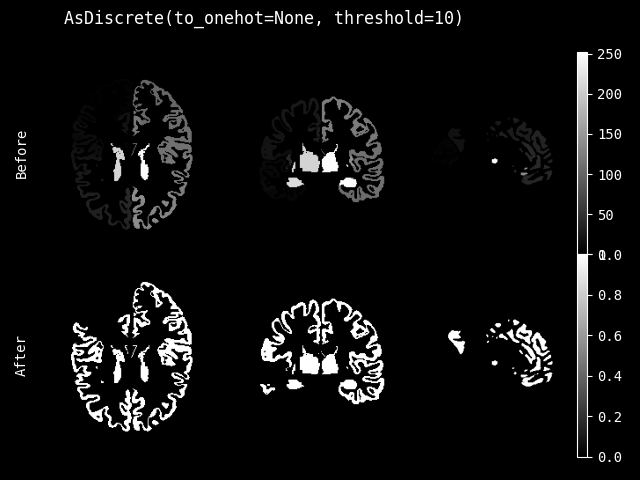class monai.transforms.AsDiscrete(argmax=False, to_onehot=None, threshold=None, rounding=None, **kwargs)[source]#

Convert the input tensor/array into discrete values, possible operations are:

• argmax.

• threshold input value to binary values.

• convert input value to One-Hot format (set `to_one_hot=N`, N is the number of classes).

• round the value to the closest integer.

Parameters:
• argmax – whether to execute argmax function on input data before transform. Defaults to `False`.

• to_onehot – if not None, convert input data into the one-hot format with specified number of classes. Defaults to `None`.

• threshold – if not None, threshold the float values to int number 0 or 1 with specified threshold. Defaults to `None`.

• rounding – if not None, round the data according to the specified option, available options: [“torchrounding”].

• kwargs – additional parameters to torch.argmax, monai.networks.one_hot. currently `dim`, `keepdim`, `dtype` are supported, unrecognized parameters will be ignored. These default to `0`, `True`, `torch.float` respectively.

Example

```>>> transform = AsDiscrete(argmax=True)
>>> print(transform(np.array([[[0.0, 1.0]], [[2.0, 3.0]]])))
# [[[1.0, 1.0]]]
```
```>>> transform = AsDiscrete(threshold=0.6)
>>> print(transform(np.array([[[0.0, 0.5], [0.8, 3.0]]])))
# [[[0.0, 0.0], [1.0, 1.0]]]
```
```>>> transform = AsDiscrete(argmax=True, to_onehot=2, threshold=0.5)
>>> print(transform(np.array([[[0.0, 1.0]], [[2.0, 3.0]]])))
# [[[0.0, 0.0]], [[1.0, 1.0]]]
```
__call__(img, argmax=None, to_onehot=None, threshold=None, rounding=None)[source]#
Parameters:
• img – the input tensor data to convert, if no channel dimension when converting to One-Hot, will automatically add it.

• argmax – whether to execute argmax function on input data before transform. Defaults to `self.argmax`.

• to_onehot – if not None, convert input data into the one-hot format with specified number of classes. Defaults to `self.to_onehot`.

• threshold – if not None, threshold the float values to int number 0 or 1 with specified threshold value. Defaults to `self.threshold`.

• rounding – if not None, round the data according to the specified option, available options: [“torchrounding”].

#### KeepLargestConnectedComponent#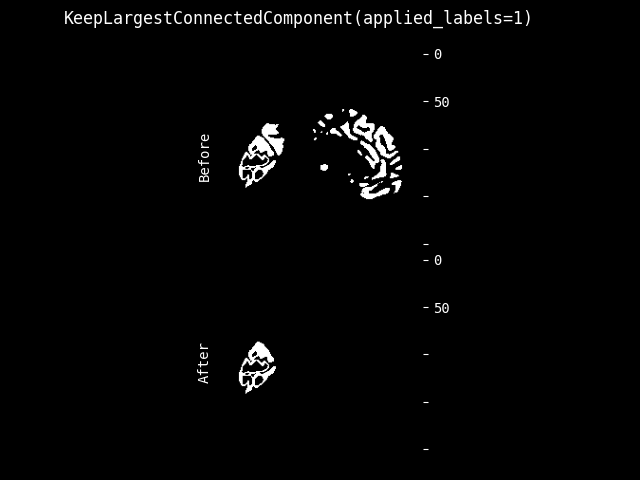class monai.transforms.KeepLargestConnectedComponent(applied_labels=None, is_onehot=None, independent=True, connectivity=None, num_components=1)[source]#

Keeps only the largest connected component in the image. This transform can be used as a post-processing step to clean up over-segment areas in model output.

The input is assumed to be a channel-first PyTorch Tensor:

1) For not OneHot format data, the values correspond to expected labels, 0 will be treated as background and the over-segment pixels will be set to 0. 2) For OneHot format data, the values should be 0, 1 on each labels, the over-segment pixels will be set to 0 in its channel.

For example: Use with applied_labels=, is_onehot=False, connectivity=1:

```[1, 0, 0]         [0, 0, 0]
[0, 1, 1]    =>   [0, 1 ,1]
[0, 1, 1]         [0, 1, 1]
```

Use with applied_labels=[1, 2], is_onehot=False, independent=False, connectivity=1:

```[0, 0, 1, 0 ,0]           [0, 0, 1, 0 ,0]
[0, 2, 1, 1 ,1]           [0, 2, 1, 1 ,1]
[1, 2, 1, 0 ,0]    =>     [1, 2, 1, 0 ,0]
[1, 2, 0, 1 ,0]           [1, 2, 0, 0 ,0]
[2, 2, 0, 0 ,2]           [2, 2, 0, 0 ,0]
```

Use with applied_labels=[1, 2], is_onehot=False, independent=True, connectivity=1:

```[0, 0, 1, 0 ,0]           [0, 0, 1, 0 ,0]
[0, 2, 1, 1 ,1]           [0, 2, 1, 1 ,1]
[1, 2, 1, 0 ,0]    =>     [0, 2, 1, 0 ,0]
[1, 2, 0, 1 ,0]           [0, 2, 0, 0 ,0]
[2, 2, 0, 0 ,2]           [2, 2, 0, 0 ,0]
```

Use with applied_labels=[1, 2], is_onehot=False, independent=False, connectivity=2:

```[0, 0, 1, 0 ,0]           [0, 0, 1, 0 ,0]
[0, 2, 1, 1 ,1]           [0, 2, 1, 1 ,1]
[1, 2, 1, 0 ,0]    =>     [1, 2, 1, 0 ,0]
[1, 2, 0, 1 ,0]           [1, 2, 0, 1 ,0]
[2, 2, 0, 0 ,2]           [2, 2, 0, 0 ,2]
```
__call__(img)[source]#
Parameters:

img (`Union`[`ndarray`, `Tensor`]) – shape must be (C, spatial_dim1[, spatial_dim2, …]).

Return type:

`Union`[`ndarray`, `Tensor`]

Returns:

An array with shape (C, spatial_dim1[, spatial_dim2, …]).

__init__(applied_labels=None, is_onehot=None, independent=True, connectivity=None, num_components=1)[source]#
Parameters:
• applied_labels – Labels for applying the connected component analysis on. If given, voxels whose value is in this list will be analyzed. If None, all non-zero values will be analyzed.

• is_onehot – if True, treat the input data as OneHot format data, otherwise, not OneHot format data. default to None, which treats multi-channel data as OneHot and single channel data as not OneHot.

• independent – whether to treat `applied_labels` as a union of foreground labels. If `True`, the connected component analysis will be performed on each foreground label independently and return the intersection of the largest components. If `False`, the analysis will be performed on the union of foreground labels. default is True.

• connectivity – Maximum number of orthogonal hops to consider a pixel/voxel as a neighbor. Accepted values are ranging from 1 to input.ndim. If `None`, a full connectivity of `input.ndim` is used. for more details: https://scikit-image.org/docs/dev/api/skimage.measure.html#skimage.measure.label.

• num_components – The number of largest components to preserve.

#### DistanceTransformEDT#

class monai.transforms.DistanceTransformEDT(sampling=None)[source]#

Applies the Euclidean distance transform on the input. Either GPU based with CuPy / cuCIM or CPU based with scipy. To use the GPU implementation, make sure cuCIM is available and that the data is a torch.tensor on a GPU device.

Note that the results of the libraries can differ, so stick to one if possible. For details, check out the SciPy and cuCIM documentation and / or `monai.transforms.utils.distance_transform_edt()`.

__call__(img)[source]#
Parameters:
• img (`Union`[`ndarray`, `Tensor`]) – Input image on which the distance transform shall be run. Has to be a channel first array, must have shape: (num_channels, H, W [,D]). Can be of any type but will be converted into binary: 1 wherever image equates to True, 0 elsewhere. Input gets passed channel-wise to the distance-transform, thus results from this function will differ from directly calling `distance_transform_edt()` in CuPy or SciPy.

• sampling – Spacing of elements along each dimension. If a sequence, must be of length equal to the input rank -1; if a single number, this is used for all axes. If not specified, a grid spacing of unity is implied.

Return type:

`Union`[`ndarray`, `Tensor`]

Returns:

An array with the same shape and data type as img

#### RemoveSmallObjects#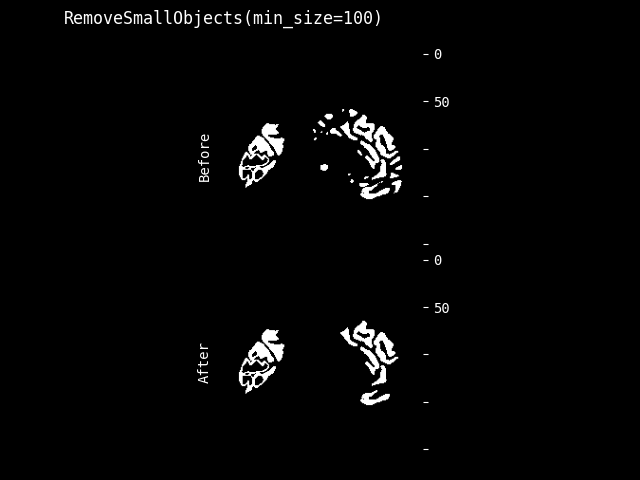class monai.transforms.RemoveSmallObjects(min_size=64, connectivity=1, independent_channels=True, by_measure=False, pixdim=None)[source]#

Use skimage.morphology.remove_small_objects to remove small objects from images. See: https://scikit-image.org/docs/dev/api/skimage.morphology.html#remove-small-objects.

Data should be one-hotted.

Parameters:
• min_size – objects smaller than this size (in number of voxels; or surface area/volume value in whatever units your image is if by_measure is True) are removed.

• connectivity – Maximum number of orthogonal hops to consider a pixel/voxel as a neighbor. Accepted values are ranging from 1 to input.ndim. If `None`, a full connectivity of `input.ndim` is used. For more details refer to linked scikit-image documentation.

• independent_channels – Whether or not to consider channels as independent. If true, then conjoining islands from different labels will be removed if they are below the threshold. If false, the overall size islands made from all non-background voxels will be used.

• by_measure – Whether the specified min_size is in number of voxels. if this is True then min_size represents a surface area or volume value of whatever units your image is in (mm^3, cm^2, etc.) default is False. e.g. if min_size is 3, by_measure is True and the units of your data is mm, objects smaller than 3mm^3 are removed.

• pixdim – the pixdim of the input image. if a single number, this is used for all axes. If a sequence of numbers, the length of the sequence must be equal to the image dimensions.

Example:

```.. code-block:: python

from monai.transforms import RemoveSmallObjects, Spacing, Compose
from monai.data import MetaTensor

data1 = torch.tensor([[[0, 0, 0, 0, 0], [0, 1, 1, 0, 1], [0, 0, 0, 1, 1]]])
affine = torch.as_tensor([[2,0,0,0],
[0,1,0,0],
[0,0,1,0],
[0,0,0,1]], dtype=torch.float64)
data2 = MetaTensor(data1, affine=affine)

# remove objects smaller than 3mm^3, input is MetaTensor
trans = RemoveSmallObjects(min_size=3, by_measure=True)
out = trans(data2)
# remove objects smaller than 3mm^3, input is not MetaTensor
trans = RemoveSmallObjects(min_size=3, by_measure=True, pixdim=(2, 1, 1))
out = trans(data1)

# remove objects smaller than 3 (in pixel)
trans = RemoveSmallObjects(min_size=3)
out = trans(data2)

# If the affine of the data is not identity, you can also add Spacing before.
trans = Compose([
Spacing(pixdim=(1, 1, 1)),
RemoveSmallObjects(min_size=3)
])
```
__call__(img)[source]#
Parameters:

img (`Union`[`ndarray`, `Tensor`]) – shape must be (C, spatial_dim1[, spatial_dim2, …]). Data should be one-hotted.

Return type:

`Union`[`ndarray`, `Tensor`]

Returns:

An array with shape (C, spatial_dim1[, spatial_dim2, …]).

#### LabelFilter#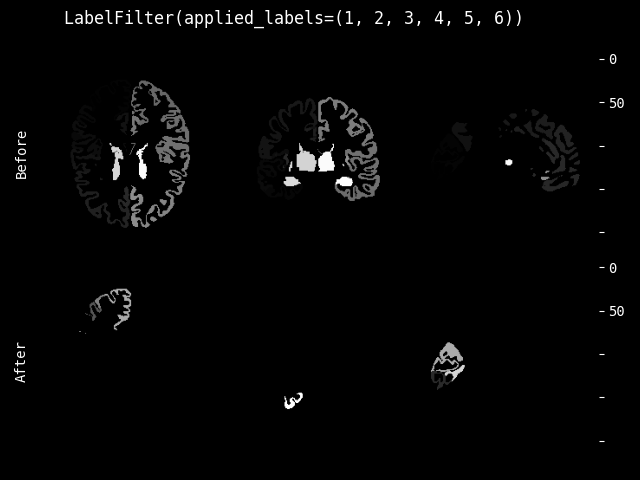class monai.transforms.LabelFilter(applied_labels)[source]#

This transform filters out labels and can be used as a processing step to view only certain labels.

The list of applied labels defines which labels will be kept.

Note

All labels which do not match the applied_labels are set to the background label (0).

For example:

Use LabelFilter with applied_labels=[1, 5, 9]:

```[1, 2, 3]         [1, 0, 0]
[4, 5, 6]    =>   [0, 5 ,0]
[7, 8, 9]         [0, 0, 9]
```
__call__(img)[source]#

Filter the image on the applied_labels.

Parameters:

img (`Union`[`ndarray`, `Tensor`]) – Pytorch tensor or numpy array of any shape.

Raises:

NotImplementedError – The provided image was not a Pytorch Tensor or numpy array.

Return type:

`Union`[`ndarray`, `Tensor`]

Returns:

Pytorch tensor or numpy array of the same shape as the input.

__init__(applied_labels)[source]#

Initialize the LabelFilter class with the labels to filter on.

Parameters:

applied_labels – Label(s) to filter on.

#### FillHoles#

class monai.transforms.FillHoles(applied_labels=None, connectivity=None)[source]#

This transform fills holes in the image and can be used to remove artifacts inside segments.

An enclosed hole is defined as a background pixel/voxel which is only enclosed by a single class. The definition of enclosed can be defined with the connectivity parameter:

```1-connectivity     2-connectivity     diagonal connection close-up

[ ]           [ ]  [ ]  [ ]             [ ]
|               \  |  /                 |  <- hop 2
[ ]--[x]--[ ]      [ ]--[x]--[ ]        [x]--[ ]
|               /  |  \             hop 1
[ ]           [ ]  [ ]  [ ]
```

It is possible to define for which labels the hole filling should be applied. The input image is assumed to be a PyTorch Tensor or numpy array with shape [C, spatial_dim1[, spatial_dim2, …]]. If C = 1, then the values correspond to expected labels. If C > 1, then a one-hot-encoding is expected where the index of C matches the label indexing.

Note

The label 0 will be treated as background and the enclosed holes will be set to the neighboring class label.

The performance of this method heavily depends on the number of labels. It is a bit faster if the list of applied_labels is provided. Limiting the number of applied_labels results in a big decrease in processing time.

For example:

Use FillHoles with default parameters:

```[1, 1, 1, 2, 2, 2, 3, 3]         [1, 1, 1, 2, 2, 2, 3, 3]
[1, 0, 1, 2, 0, 0, 3, 0]    =>   [1, 1 ,1, 2, 0, 0, 3, 0]
[1, 1, 1, 2, 2, 2, 3, 3]         [1, 1, 1, 2, 2, 2, 3, 3]
```

The hole in label 1 is fully enclosed and therefore filled with label 1. The background label near label 2 and 3 is not fully enclosed and therefore not filled.

__call__(img)[source]#

Fill the holes in the provided image.

Note

The value 0 is assumed as background label.

Parameters:

img (`Union`[`ndarray`, `Tensor`]) – Pytorch Tensor or numpy array of shape [C, spatial_dim1[, spatial_dim2, …]].

Raises:

NotImplementedError – The provided image was not a Pytorch Tensor or numpy array.

Return type:

`Union`[`ndarray`, `Tensor`]

Returns:

Pytorch Tensor or numpy array of shape [C, spatial_dim1[, spatial_dim2, …]].

__init__(applied_labels=None, connectivity=None)[source]#

Initialize the connectivity and limit the labels for which holes are filled.

Parameters:
• applied_labels – Labels for which to fill holes. Defaults to None, that is filling holes for all labels.

• connectivity – Maximum number of orthogonal hops to consider a pixel/voxel as a neighbor. Accepted values are ranging from 1 to input.ndim. Defaults to a full connectivity of `input.ndim`.

#### LabelToContour#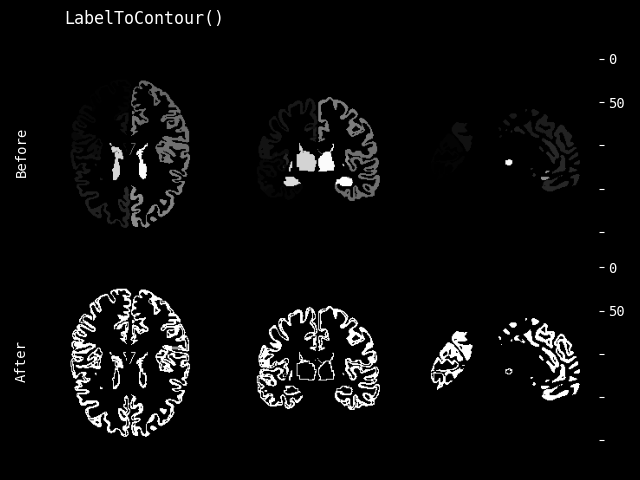class monai.transforms.LabelToContour(kernel_type='Laplace')[source]#

Return the contour of binary input images that only compose of 0 and 1, with Laplacian kernel set as default for edge detection. Typical usage is to plot the edge of label or segmentation output.

Parameters:

kernel_type (`str`) – the method applied to do edge detection, default is “Laplace”.

Raises:

NotImplementedError – When `kernel_type` is not “Laplace”.

__call__(img)[source]#
Parameters:

img (`Union`[`ndarray`, `Tensor`]) – torch tensor data to extract the contour, with shape: [channels, height, width[, depth]]

Raises:

ValueError – When `image` ndim is not one of [3, 4].

Returns:

1. it’s the binary classification result of whether a pixel is edge or not.

2. in order to keep the original shape of mask image, we use padding as default.

3. the edge detection is just approximate because it defects inherent to Laplace kernel, ideally the edge should be thin enough, but now it has a thickness.

Return type:

A torch tensor with the same shape as img, note

#### MeanEnsemble#

class monai.transforms.MeanEnsemble(weights=None)[source]#

Execute mean ensemble on the input data. The input data can be a list or tuple of PyTorch Tensor with shape: [C[, H, W, D]], Or a single PyTorch Tensor with shape: [E, C[, H, W, D]], the E dimension represents the output data from different models. Typically, the input data is model output of segmentation task or classification task. And it also can support to add weights for the input data.

Parameters:

weights – can be a list or tuple of numbers for input data with shape: [E, C, H, W[, D]]. or a Numpy ndarray or a PyTorch Tensor data. the weights will be added to input data from highest dimension, for example: 1. if the weights only has 1 dimension, it will be added to the E dimension of input data. 2. if the weights has 2 dimensions, it will be added to E and C dimensions. it’s a typical practice to add weights for different classes: to ensemble 3 segmentation model outputs, every output has 4 channels(classes), so the input data shape can be: [3, 4, H, W, D]. and add different weights for different classes, so the weights shape can be: [3, 4]. for example: weights = [[1, 2, 3, 4], [4, 3, 2, 1], [1, 1, 1, 1]].

__call__(img)[source]#

Call self as a function.

#### ProbNMS#

class monai.transforms.ProbNMS(spatial_dims=2, sigma=0.0, prob_threshold=0.5, box_size=48)[source]#

Performs probability based non-maximum suppression (NMS) on the probabilities map via iteratively selecting the coordinate with highest probability and then move it as well as its surrounding values. The remove range is determined by the parameter box_size. If multiple coordinates have the same highest probability, only one of them will be selected.

Parameters:
• spatial_dims – number of spatial dimensions of the input probabilities map. Defaults to 2.

• sigma – the standard deviation for gaussian filter. It could be a single value, or spatial_dims number of values. Defaults to 0.0.

• prob_threshold – the probability threshold, the function will stop searching if the highest probability is no larger than the threshold. The value should be no less than 0.0. Defaults to 0.5.

• box_size – the box size (in pixel) to be removed around the pixel with the maximum probability. It can be an integer that defines the size of a square or cube, or a list containing different values for each dimensions. Defaults to 48.

Returns:

a list of selected lists, where inner lists contain probability and coordinates. For example, for 3D input, the inner lists are in the form of [probability, x, y, z].

Raises:
• ValueError – When `prob_threshold` is less than 0.0.

• ValueError – When `box_size` is a list or tuple, and its length is not equal to spatial_dims.

• ValueError – When `box_size` has a less than 1 value.

Calculate Sobel gradients of a grayscale image with the shape of CxH[xWxDx…] or BxH[xWxDx…].

Parameters:
• kernel_size – the size of the Sobel kernel. Defaults to 3.

• spatial_axes – the axes that define the direction of the gradient to be calculated. It calculate the gradient along each of the provide axis. By default it calculate the gradient for all spatial axes.

• normalize_kernels – if normalize the Sobel kernel to provide proper gradients. Defaults to True.

• normalize_gradients – if normalize the output gradient to 0 and 1. Defaults to False.

• padding_mode – the padding mode of the image when convolving with Sobel kernels. Defaults to “reflect”. Acceptable values are `'zeros'`, `'reflect'`, `'replicate'` or `'circular'`. See `torch.nn.Conv1d()` for more information.

• dtype – kernel data type (torch.dtype). Defaults to torch.float32.

__call__(image)[source]#

`data` is an element which often comes from an iteration over an iterable, such as `torch.utils.data.Dataset`. This method should return an updated version of `data`. To simplify the input validations, most of the transforms assume that

• `data` is a Numpy ndarray, PyTorch Tensor or string,

• the data shape can be:

1. string data without shape, LoadImage transform expects file paths,

2. most of the pre-/post-processing transforms expect: `(num_channels, spatial_dim_1[, spatial_dim_2, ...])`, except for example: AddChannel expects (spatial_dim_1[, spatial_dim_2, …])

• the channel dimension is often not omitted even if number of channels is one.

This method can optionally take additional arguments to help execute transformation operation.

Raises:

NotImplementedError – When the subclass does not override this method.

Return type:

`Tensor`

#### VoteEnsemble#

class monai.transforms.VoteEnsemble(num_classes=None)[source]#

Execute vote ensemble on the input data. The input data can be a list or tuple of PyTorch Tensor with shape: [C[, H, W, D]], Or a single PyTorch Tensor with shape: [E[, C, H, W, D]], the E dimension represents the output data from different models. Typically, the input data is model output of segmentation task or classification task.

Note

This vote transform expects the input data is discrete values. It can be multiple channels data in One-Hot format or single channel data. It will vote to select the most common data between items. The output data has the same shape as every item of the input data.

Parameters:

num_classes – if the input is single channel data instead of One-Hot, we can’t get class number from channel, need to explicitly specify the number of classes to vote.

__call__(img)[source]#

Call self as a function.

### Signal#

#### SignalRandDrop#

class monai.transforms.SignalRandDrop(boundaries=(0.0, 1.0))[source]#

Randomly drop a portion of a signal

__call__(signal)[source]#
Parameters:

signal (`Union`[`ndarray`, `Tensor`]) – input 1 dimension signal to be dropped

Return type:

`Union`[`ndarray`, `Tensor`]

__init__(boundaries=(0.0, 1.0))[source]#
Parameters:
• boundaries (`Sequence`[`float`]) – list defining lower and upper boundaries for the signal drop,

• default (lower and upper values need to be positive) – `[0.0, 1.0]`

#### SignalRandScale#

class monai.transforms.SignalRandScale(boundaries=(-1.0, 1.0))[source]#

Apply a random rescaling on a signal

__call__(signal)[source]#
Parameters:

signal (`Union`[`ndarray`, `Tensor`]) – input 1 dimension signal to be scaled

Return type:

`Union`[`ndarray`, `Tensor`]

__init__(boundaries=(-1.0, 1.0))[source]#
Parameters:

boundaries (`Sequence`[`float`]) – list defining lower and upper boundaries for the signal scaling, default : `[-1.0, 1.0]`

#### SignalRandShift#

class monai.transforms.SignalRandShift(mode='wrap', filling=0.0, boundaries=(-1.0, 1.0))[source]#

Apply a random shift on a signal

__call__(signal)[source]#
Parameters:

signal (`Union`[`ndarray`, `Tensor`]) – input 1 dimension signal to be shifted

Return type:

`Union`[`ndarray`, `Tensor`]

__init__(mode='wrap', filling=0.0, boundaries=(-1.0, 1.0))[source]#
Parameters:

Add a random sinusoidal signal to the input signal

__call__(signal)[source]#
Parameters:

signal (`Union`[`ndarray`, `Tensor`]) – input 1 dimension signal to which sinusoidal signal will be added

Return type:

`Union`[`ndarray`, `Tensor`]

__init__(boundaries=(0.1, 0.3), frequencies=(0.001, 0.02))[source]#
Parameters:
• boundaries (`Sequence`[`float`]) – list defining lower and upper boundaries for the sinusoidal magnitude, lower and upper values need to be positive ,default : `[0.1, 0.3]`

• frequencies (`Sequence`[`float`]) – list defining lower and upper frequencies for sinusoidal signal generation ,default : `[0.001, 0.02]`

Add a random square pulse signal to the input signal

__call__(signal)[source]#
Parameters:

signal (`Union`[`ndarray`, `Tensor`]) – input 1 dimension signal to which square pulse will be added

Return type:

`Union`[`ndarray`, `Tensor`]

__init__(boundaries=(0.01, 0.2), frequencies=(0.001, 0.02))[source]#
Parameters:
• boundaries (`Sequence`[`float`]) – list defining lower and upper boundaries for the square pulse magnitude, lower and upper values need to be positive , default : `[0.01, 0.2]`

• frequencies (`Sequence`[`float`]) – list defining lower and upper frequencies for the square pulse signal generation , default : `[0.001, 0.02]`

Add a random gaussian noise to the input signal

__call__(signal)[source]#
Parameters:

signal (`Union`[`ndarray`, `Tensor`]) – input 1 dimension signal to which gaussian noise will be added

Return type:

`Union`[`ndarray`, `Tensor`]

__init__(boundaries=(0.001, 0.02))[source]#
Parameters:

boundaries (`Sequence`[`float`]) – list defining lower and upper boundaries for the signal magnitude, default : `[0.001,0.02]`

class monai.transforms.SignalRandAddSinePartial(boundaries=(0.1, 0.3), frequencies=(0.001, 0.02), fraction=(0.01, 0.2))[source]#

Add a random partial sinusoidal signal to the input signal

__call__(signal)[source]#
Parameters:
• signal (`Union`[`ndarray`, `Tensor`]) – input 1 dimension signal to which a partial sinusoidal signal

Return type:

`Union`[`ndarray`, `Tensor`]

__init__(boundaries=(0.1, 0.3), frequencies=(0.001, 0.02), fraction=(0.01, 0.2))[source]#
Parameters:
• boundaries (`Sequence`[`float`]) – list defining lower and upper boundaries for the sinusoidal magnitude, lower and upper values need to be positive , default : `[0.1, 0.3]`

• frequencies (`Sequence`[`float`]) – list defining lower and upper frequencies for sinusoidal signal generation , default : `[0.001, 0.02]`

• fraction (`Sequence`[`float`]) – list defining lower and upper boundaries for partial signal generation default : `[0.01, 0.2]`

class monai.transforms.SignalRandAddSquarePulsePartial(boundaries=(0.01, 0.2), frequencies=(0.001, 0.02), fraction=(0.01, 0.2))[source]#

Add a random partial square pulse to a signal

__call__(signal)[source]#
Parameters:

signal (`Union`[`ndarray`, `Tensor`]) – input 1 dimension signal to which a partial square pulse will be added

Return type:

`Union`[`ndarray`, `Tensor`]

__init__(boundaries=(0.01, 0.2), frequencies=(0.001, 0.02), fraction=(0.01, 0.2))[source]#
Parameters:
• boundaries (`Sequence`[`float`]) – list defining lower and upper boundaries for the square pulse magnitude, lower and upper values need to be positive , default : `[0.01, 0.2]`

• frequencies (`Sequence`[`float`]) – list defining lower and upper frequencies for square pulse signal generation example : `[0.001, 0.02]`

• fraction (`Sequence`[`float`]) – list defining lower and upper boundaries for partial square pulse generation default: `[0.01, 0.2]`

#### SignalFillEmpty#

class monai.transforms.SignalFillEmpty(replacement=0.0)[source]#

replace empty part of a signal (NaN)

__call__(signal)[source]#
Parameters:

signal (`Union`[`ndarray`, `Tensor`]) – signal to be filled

Return type:

`Union`[`ndarray`, `Tensor`]

__init__(replacement=0.0)[source]#
Parameters:

replacement (`float`) – value to replace nan items in signal

#### SignalRemoveFrequency#

class monai.transforms.SignalRemoveFrequency(frequency=None, quality_factor=None, sampling_freq=None)[source]#

Remove a frequency from a signal

__call__(signal)[source]#
Parameters:

signal (`ndarray`) – signal to be frequency removed

Return type:

`Any`

__init__(frequency=None, quality_factor=None, sampling_freq=None)[source]#
Parameters:

#### SignalContinuousWavelet#

class monai.transforms.SignalContinuousWavelet(type='mexh', length=125.0, frequency=500.0)[source]#

Generate continuous wavelet transform of a signal

__call__(signal)[source]#
Parameters:

signal (`ndarray`) – signal for which to generate continuous wavelet transform

Return type:

`Any`

__init__(type='mexh', length=125.0, frequency=500.0)[source]#
Parameters:
• type (`str`) – mother wavelet type. Available options are: {`"mexh"`, `"morl"`, `"cmorB-C"`, , `"gausP"`}

• length (`float`) – expected length, default `125.0`

• frequency (`float`) – signal frequency, default `500.0`

### Spatial#

#### SpatialResample#

Resample input image from the orientation/spacing defined by `src_affine` affine matrix into the ones specified by `dst_affine` affine matrix.

Internally this transform computes the affine transform matrix from `src_affine` to `dst_affine`, by `xform = linalg.solve(src_affine, dst_affine)`, and call `monai.transforms.Affine` with `xform`.

This transform is capable of lazy execution. See the Lazy Resampling topic for more information.

__call__(img, dst_affine=None, spatial_size=None, mode=None, padding_mode=None, align_corners=None, dtype=None, lazy=None)[source]#
Parameters:
• img – input image to be resampled. It currently supports channel-first arrays with at most three spatial dimensions.

• dst_affine – destination affine matrix. Defaults to `None`, which means the same as img.affine. the shape should be (r+1, r+1) where r is the spatial rank of `img`. when dst_affine and spatial_size are None, the input will be returned without resampling, but the data type will be float32.

• spatial_size – output image spatial size. if spatial_size and self.spatial_size are not defined, the transform will compute a spatial size automatically containing the previous field of view. if spatial_size is `-1` are the transform will use the corresponding input img size.

• mode – {`"bilinear"`, `"nearest"`} or spline interpolation order 0-5 (integers). Interpolation mode to calculate output values. Defaults to `self.mode`. See also: https://pytorch.org/docs/stable/generated/torch.nn.functional.grid_sample.html When it’s an integer, the numpy (cpu tensor)/cupy (cuda tensor) backends will be used and the value represents the order of the spline interpolation. See also: https://docs.scipy.org/doc/scipy/reference/generated/scipy.ndimage.map_coordinates.html

• padding_mode – {`"zeros"`, `"border"`, `"reflection"`} Padding mode for outside grid values. Defaults to `self.padding_mode`. See also: https://pytorch.org/docs/stable/generated/torch.nn.functional.grid_sample.html When mode is an integer, using numpy/cupy backends, this argument accepts {‘reflect’, ‘grid-mirror’, ‘constant’, ‘grid-constant’, ‘nearest’, ‘mirror’, ‘grid-wrap’, ‘wrap’}. See also: https://docs.scipy.org/doc/scipy/reference/generated/scipy.ndimage.map_coordinates.html

• align_corners – Geometrically, we consider the pixels of the input as squares rather than points. See also: https://pytorch.org/docs/stable/generated/torch.nn.functional.grid_sample.html Defaults to `None`, effectively using the value of self.align_corners.

• dtype – data type for resampling computation. Defaults to `self.dtype` or `np.float64` (for best precision). If `None`, use the data type of input data. To be compatible with other modules, the output data type is always float32.

• lazy – a flag to indicate whether this transform should execute lazily or not during this call. Setting this to False or True overrides the `lazy` flag set during initialization for this call. Defaults to None.

The spatial rank is determined by the smallest among `img.ndim -1`, `len(src_affine) - 1`, and `3`.

When both `monai.config.USE_COMPILED` and `align_corners` are set to `True`, MONAI’s resampling implementation will be used. Set dst_affine and spatial_size to None to turn off the resampling step.

Parameters:
inverse(data)[source]#

Inverse of `__call__`.

Raises:

NotImplementedError – When the subclass does not override this method.

Return type:

`Tensor`

#### ResampleToMatch#

Resample an image to match given metadata. The affine matrix will be aligned, and the size of the output image will match.

This transform is capable of lazy execution. See the Lazy Resampling topic for more information.

__call__(img, img_dst, mode=None, padding_mode=None, align_corners=None, dtype=None, lazy=None)[source]#
Parameters:
Raises:

ValueError – When the affine matrix of the source image is not invertible.

Returns:

Resampled input tensor or MetaTensor.

#### Spacing#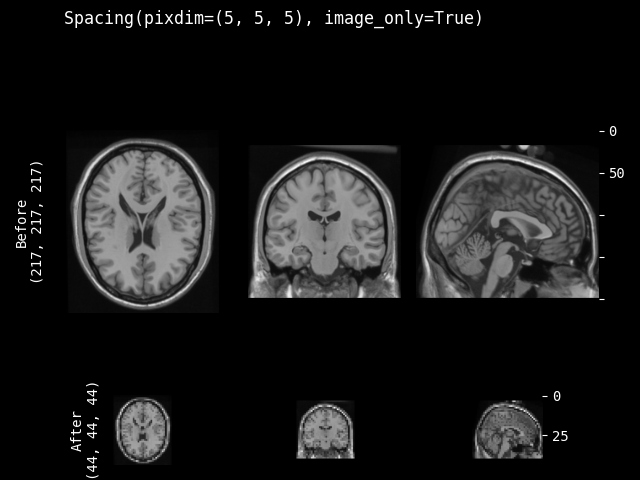class monai.transforms.Spacing(pixdim, diagonal=False, mode=GridSampleMode.BILINEAR, padding_mode=GridSamplePadMode.BORDER, align_corners=False, dtype=<class 'numpy.float64'>, scale_extent=False, recompute_affine=False, min_pixdim=None, max_pixdim=None, lazy=False)[source]#

Resample input image into the specified pixdim.

This transform is capable of lazy execution. See the Lazy Resampling topic for more information.

__call__(data_array, mode=None, padding_mode=None, align_corners=None, dtype=None, scale_extent=None, output_spatial_shape=None, lazy=None)[source]#
Parameters:
• data_array – in shape (num_channels, H[, W, …]).

• mode – {`"bilinear"`, `"nearest"`} or spline interpolation order 0-5 (integers). Interpolation mode to calculate output values. Defaults to `"self.mode"`. See also: https://pytorch.org/docs/stable/generated/torch.nn.functional.grid_sample.html When it’s an integer, the numpy (cpu tensor)/cupy (cuda tensor) backends will be used and the value represents the order of the spline interpolation. See also: https://docs.scipy.org/doc/scipy/reference/generated/scipy.ndimage.map_coordinates.html

• padding_mode – {`"zeros"`, `"border"`, `"reflection"`} Padding mode for outside grid values. Defaults to `"self.padding_mode"`. See also: https://pytorch.org/docs/stable/generated/torch.nn.functional.grid_sample.html When mode is an integer, using numpy/cupy backends, this argument accepts {‘reflect’, ‘grid-mirror’, ‘constant’, ‘grid-constant’, ‘nearest’, ‘mirror’, ‘grid-wrap’, ‘wrap’}. See also: https://docs.scipy.org/doc/scipy/reference/generated/scipy.ndimage.map_coordinates.html

• align_corners – Geometrically, we consider the pixels of the input as squares rather than points. See also: https://pytorch.org/docs/stable/generated/torch.nn.functional.grid_sample.html Defaults to `None`, effectively using the value of self.align_corners.

• dtype – data type for resampling computation. Defaults to `self.dtype`. If None, use the data type of input data. To be compatible with other modules, the output data type is always `float32`.

• scale_extent – whether the scale is computed based on the spacing or the full extent of voxels, The option is ignored if output spatial size is specified when calling this transform. See also: `monai.data.utils.compute_shape_offset()`. When this is True, align_corners should be True because compute_shape_offset already provides the corner alignment shift/scaling.

• output_spatial_shape – specify the shape of the output data_array. This is typically useful for the inverse of Spacingd where sometimes we could not compute the exact shape due to the quantization error with the affine.

• lazy – a flag to indicate whether this transform should execute lazily or not during this call. Setting this to False or True overrides the `lazy` flag set during initialization for this call. Defaults to None.

Raises:
• ValueError – When `data_array` has no spatial dimensions.

• ValueError – When `pixdim` is nonpositive.

Returns:

data tensor or MetaTensor (resampled into self.pixdim).

__init__(pixdim, diagonal=False, mode=GridSampleMode.BILINEAR, padding_mode=GridSamplePadMode.BORDER, align_corners=False, dtype=<class 'numpy.float64'>, scale_extent=False, recompute_affine=False, min_pixdim=None, max_pixdim=None, lazy=False)[source]#
Parameters:
• pixdim – output voxel spacing. if providing a single number, will use it for the first dimension. items of the pixdim sequence map to the spatial dimensions of input image, if length of pixdim sequence is longer than image spatial dimensions, will ignore the longer part, if shorter, will pad with the last value. For example, for 3D image if pixdim is [1.0, 2.0] it will be padded to [1.0, 2.0, 2.0] if the components of the pixdim are non-positive values, the transform will use the corresponding components of the original pixdim, which is computed from the affine matrix of input image.

• diagonal

whether to resample the input to have a diagonal affine matrix. If True, the input data is resampled to the following affine:

```np.diag((pixdim_0, pixdim_1, ..., pixdim_n, 1))
```

This effectively resets the volume to the world coordinate system (RAS+ in nibabel). The original orientation, rotation, shearing are not preserved.

If False, this transform preserves the axes orientation, orthogonal rotation and translation components from the original affine. This option will not flip/swap axes of the original data.

• mode – {`"bilinear"`, `"nearest"`} or spline interpolation order 0-5 (integers). Interpolation mode to calculate output values. Defaults to `"bilinear"`. See also: https://pytorch.org/docs/stable/generated/torch.nn.functional.grid_sample.html When it’s an integer, the numpy (cpu tensor)/cupy (cuda tensor) backends will be used and the value represents the order of the spline interpolation. See also: https://docs.scipy.org/doc/scipy/reference/generated/scipy.ndimage.map_coordinates.html

• padding_mode – {`"zeros"`, `"border"`, `"reflection"`} Padding mode for outside grid values. Defaults to `"border"`. See also: https://pytorch.org/docs/stable/generated/torch.nn.functional.grid_sample.html When mode is an integer, using numpy/cupy backends, this argument accepts {‘reflect’, ‘grid-mirror’, ‘constant’, ‘grid-constant’, ‘nearest’, ‘mirror’, ‘grid-wrap’, ‘wrap’}. See also: https://docs.scipy.org/doc/scipy/reference/generated/scipy.ndimage.map_coordinates.html

• align_corners – Geometrically, we consider the pixels of the input as squares rather than points. See also: https://pytorch.org/docs/stable/generated/torch.nn.functional.grid_sample.html

• dtype – data type for resampling computation. Defaults to `float64` for best precision. If None, use the data type of input data. To be compatible with other modules, the output data type is always `float32`.

• scale_extent – whether the scale is computed based on the spacing or the full extent of voxels, default False. The option is ignored if output spatial size is specified when calling this transform. See also: `monai.data.utils.compute_shape_offset()`. When this is True, align_corners should be True because compute_shape_offset already provides the corner alignment shift/scaling.

• recompute_affine – whether to recompute affine based on the output shape. The affine computed analytically does not reflect the potential quantization errors in terms of the output shape. Set this flag to True to recompute the output affine based on the actual pixdim. Default to `False`.

• min_pixdim – minimal input spacing to be resampled. If provided, input image with a larger spacing than this value will be kept in its original spacing (not be resampled to pixdim). Set it to None to use the value of pixdim. Default to None.

• max_pixdim – maximal input spacing to be resampled. If provided, input image with a smaller spacing than this value will be kept in its original spacing (not be resampled to pixdim). Set it to None to use the value of pixdim. Default to None.

• lazy – a flag to indicate whether this transform should execute lazily or not. Defaults to False

inverse(data)[source]#

Inverse of `__call__`.

Raises:

NotImplementedError – When the subclass does not override this method.

Return type:

`Tensor`

property lazy#

Get whether lazy evaluation is enabled for this transform instance. :returns: True if the transform is operating in a lazy fashion, False if not.

#### Orientation#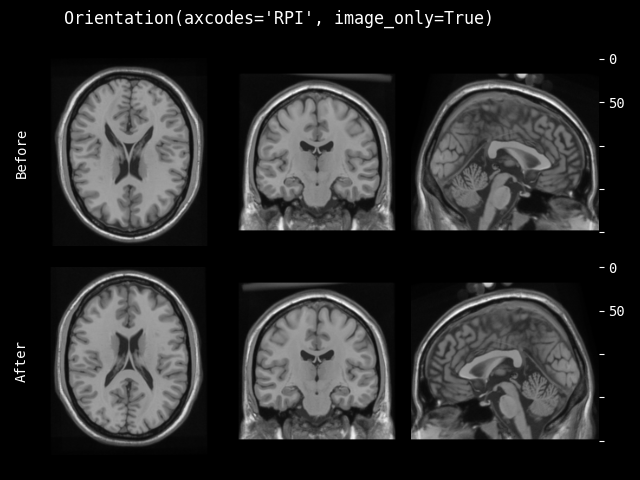class monai.transforms.Orientation(axcodes=None, as_closest_canonical=False, labels=(('L', 'R'), ('P', 'A'), ('I', 'S')), lazy=False)[source]#

Change the input image’s orientation into the specified based on axcodes.

This transform is capable of lazy execution. See the Lazy Resampling topic for more information.

__call__(data_array, lazy=None)[source]#

If input type is MetaTensor, original affine is extracted with data_array.affine. If input type is torch.Tensor, original affine is assumed to be identity.

Parameters:
• data_array – in shape (num_channels, H[, W, …]).

• lazy – a flag to indicate whether this transform should execute lazily or not during this call. Setting this to False or True overrides the `lazy` flag set during initialization for this call. Defaults to None.

Raises:
• ValueError – When `data_array` has no spatial dimensions.

• ValueError – When `axcodes` spatiality differs from `data_array`.

Returns:

data_array [reoriented in self.axcodes]. Output type will be MetaTensor

unless get_track_meta() == False, in which case it will be torch.Tensor.

__init__(axcodes=None, as_closest_canonical=False, labels=(('L', 'R'), ('P', 'A'), ('I', 'S')), lazy=False)[source]#
Parameters:
• axcodes – N elements sequence for spatial ND input’s orientation. e.g. axcodes=’RAS’ represents 3D orientation: (Left, Right), (Posterior, Anterior), (Inferior, Superior). default orientation labels options are: ‘L’ and ‘R’ for the first dimension, ‘P’ and ‘A’ for the second, ‘I’ and ‘S’ for the third.

• as_closest_canonical – if True, load the image as closest to canonical axis format.

• labels – optional, None or sequence of (2,) sequences (2,) sequences are labels for (beginning, end) of output axis. Defaults to `(('L', 'R'), ('P', 'A'), ('I', 'S'))`.

• lazy – a flag to indicate whether this transform should execute lazily or not. Defaults to False

Raises:

ValueError – When `axcodes=None` and `as_closest_canonical=True`. Incompatible values.

inverse(data)[source]#

Inverse of `__call__`.

Raises:

NotImplementedError – When the subclass does not override this method.

Return type:

`Tensor`

#### RandRotate#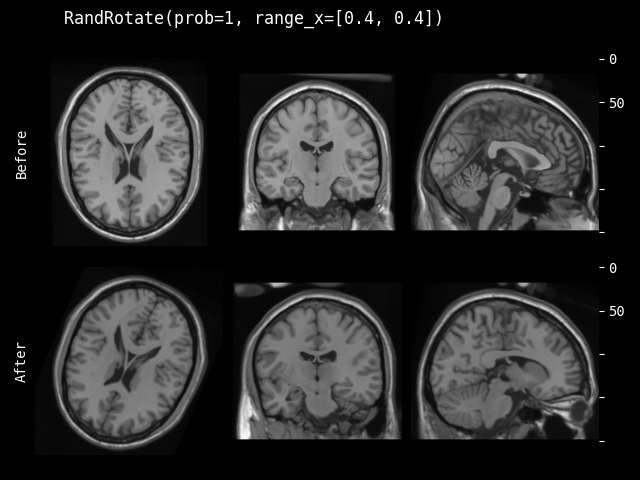class monai.transforms.RandRotate(range_x=0.0, range_y=0.0, range_z=0.0, prob=0.1, keep_size=True, mode=GridSampleMode.BILINEAR, padding_mode=GridSamplePadMode.BORDER, align_corners=False, dtype=<class 'numpy.float32'>, lazy=False)[source]#

Randomly rotate the input arrays.

This transform is capable of lazy execution. See the Lazy Resampling topic for more information.

Parameters:
• range_x – Range of rotation angle in radians in the plane defined by the first and second axes. If single number, angle is uniformly sampled from (-range_x, range_x).

• range_y – Range of rotation angle in radians in the plane defined by the first and third axes. If single number, angle is uniformly sampled from (-range_y, range_y). only work for 3D data.

• range_z – Range of rotation angle in radians in the plane defined by the second and third axes. If single number, angle is uniformly sampled from (-range_z, range_z). only work for 3D data.

• prob – Probability of rotation.

• keep_size – If it is False, the output shape is adapted so that the input array is contained completely in the output. If it is True, the output shape is the same as the input. Default is True.

• mode – {`"bilinear"`, `"nearest"`} Interpolation mode to calculate output values. Defaults to `"bilinear"`. See also: https://pytorch.org/docs/stable/generated/torch.nn.functional.grid_sample.html

• padding_mode – {`"zeros"`, `"border"`, `"reflection"`} Padding mode for outside grid values. Defaults to `"border"`. See also: https://pytorch.org/docs/stable/generated/torch.nn.functional.grid_sample.html

• dtype – data type for resampling computation. Defaults to `float32`. If None, use the data type of input data. To be compatible with other modules, the output data type is always `float32`.

• lazy – a flag to indicate whether this transform should execute lazily or not. Defaults to False

__call__(img, mode=None, padding_mode=None, align_corners=None, dtype=None, randomize=True, lazy=None)[source]#
Parameters:
• img – channel first array, must have shape 2D: (nchannels, H, W), or 3D: (nchannels, H, W, D).

• mode – {`"bilinear"`, `"nearest"`} Interpolation mode to calculate output values. Defaults to `self.mode`. See also: https://pytorch.org/docs/stable/generated/torch.nn.functional.grid_sample.html

• padding_mode – {`"zeros"`, `"border"`, `"reflection"`} Padding mode for outside grid values. Defaults to `self.padding_mode`. See also: https://pytorch.org/docs/stable/generated/torch.nn.functional.grid_sample.html

• align_corners – Defaults to `self.align_corners`. See also: https://pytorch.org/docs/stable/generated/torch.nn.functional.grid_sample.html

• dtype – data type for resampling computation. Defaults to `self.dtype`. If None, use the data type of input data. To be compatible with other modules, the output data type is always `float32`.

• randomize – whether to execute randomize() function first, default to True.

• lazy – a flag to indicate whether this transform should execute lazily or not during this call. Setting this to False or True overrides the `lazy` flag set during initialization for this call. Defaults to None.

inverse(data)[source]#

Inverse of `__call__`.

Raises:

NotImplementedError – When the subclass does not override this method.

Return type:

`Tensor`

randomize(data=None)[source]#

Within this method, `self.R` should be used, instead of np.random, to introduce random factors.

all `self.R` calls happen here so that we have a better chance to identify errors of sync the random state.

This method can generate the random factors based on properties of the input data.

#### RandFlip#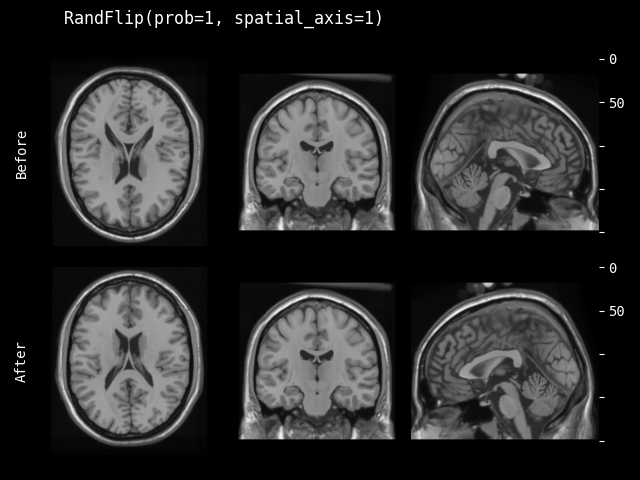class monai.transforms.RandFlip(prob=0.1, spatial_axis=None, lazy=False)[source]#

Randomly flips the image along axes. Preserves shape. See numpy.flip for additional details. https://docs.scipy.org/doc/numpy/reference/generated/numpy.flip.html

This transform is capable of lazy execution. See the Lazy Resampling topic for more information.

Parameters:
• prob – Probability of flipping.

• spatial_axis – Spatial axes along which to flip over. Default is None.

• lazy – a flag to indicate whether this transform should execute lazily or not. Defaults to False

__call__(img, randomize=True, lazy=None)[source]#
Parameters:
• img – channel first array, must have shape: (num_channels, H[, W, …, ]),

• randomize – whether to execute randomize() function first, default to True.

• lazy – a flag to indicate whether this transform should execute lazily or not during this call. Setting this to False or True overrides the `lazy` flag set during initialization for this call. Defaults to None.

inverse(data)[source]#

Inverse of `__call__`.

Raises:

NotImplementedError – When the subclass does not override this method.

Return type:

`Tensor`

property lazy#

Get whether lazy evaluation is enabled for this transform instance. :returns: True if the transform is operating in a lazy fashion, False if not.

#### RandAxisFlip#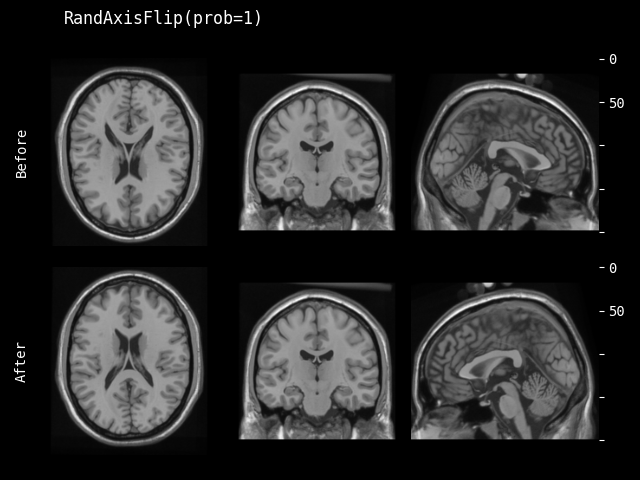class monai.transforms.RandAxisFlip(prob=0.1, lazy=False)[source]#

Randomly select a spatial axis and flip along it. See numpy.flip for additional details. https://docs.scipy.org/doc/numpy/reference/generated/numpy.flip.html

This transform is capable of lazy execution. See the Lazy Resampling topic for more information.

Parameters:
• prob (`float`) – Probability of flipping.

• lazy (`bool`) – a flag to indicate whether this transform should execute lazily or not. Defaults to False

__call__(img, randomize=True, lazy=None)[source]#
Parameters:
• img – channel first array, must have shape: (num_channels, H[, W, …, ])

• randomize – whether to execute randomize() function first, default to True.

• lazy – a flag to indicate whether this transform should execute lazily or not during this call. Setting this to False or True overrides the `lazy` flag set during initialization for this call. Defaults to None.

inverse(data)[source]#

Inverse of `__call__`.

Raises:

NotImplementedError – When the subclass does not override this method.

Return type:

`Tensor`

property lazy#

Get whether lazy evaluation is enabled for this transform instance. :returns: True if the transform is operating in a lazy fashion, False if not.

randomize(data)[source]#

Within this method, `self.R` should be used, instead of np.random, to introduce random factors.

all `self.R` calls happen here so that we have a better chance to identify errors of sync the random state.

This method can generate the random factors based on properties of the input data.

Return type:

`None`

#### RandZoom#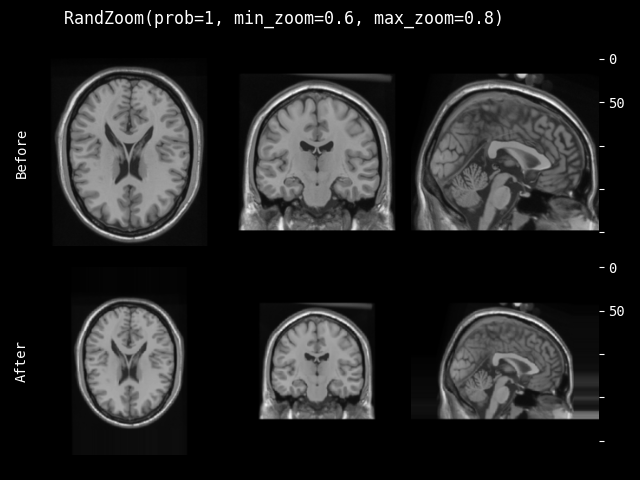Randomly zooms input arrays with given probability within given zoom range.

This transform is capable of lazy execution. See the Lazy Resampling topic for more information.

Parameters:
• prob – Probability of zooming.

• min_zoom – Min zoom factor. Can be float or sequence same size as image. If a float, select a random factor from [min_zoom, max_zoom] then apply to all spatial dims to keep the original spatial shape ratio. If a sequence, min_zoom should contain one value for each spatial axis. If 2 values provided for 3D data, use the first value for both H & W dims to keep the same zoom ratio.

• max_zoom – Max zoom factor. Can be float or sequence same size as image. If a float, select a random factor from [min_zoom, max_zoom] then apply to all spatial dims to keep the original spatial shape ratio. If a sequence, max_zoom should contain one value for each spatial axis. If 2 values provided for 3D data, use the first value for both H & W dims to keep the same zoom ratio.

• mode – {`"nearest"`, `"nearest-exact"`, `"linear"`, `"bilinear"`, `"bicubic"`, `"trilinear"`, `"area"`} The interpolation mode. Defaults to `"area"`. See also: https://pytorch.org/docs/stable/generated/torch.nn.functional.interpolate.html

• padding_mode – available modes for numpy array:{`"constant"`, `"edge"`, `"linear_ramp"`, `"maximum"`, `"mean"`, `"median"`, `"minimum"`, `"reflect"`, `"symmetric"`, `"wrap"`, `"empty"`} available modes for PyTorch Tensor: {`"constant"`, `"reflect"`, `"replicate"`, `"circular"`}. One of the listed string values or a user supplied function. Defaults to `"constant"`. The mode to pad data after zooming. See also: https://numpy.org/doc/1.18/reference/generated/numpy.pad.html https://pytorch.org/docs/stable/generated/torch.nn.functional.pad.html

• align_corners – This only has an effect when mode is ‘linear’, ‘bilinear’, ‘bicubic’ or ‘trilinear’. Default: None. See also: https://pytorch.org/docs/stable/generated/torch.nn.functional.interpolate.html

• dtype – data type for resampling computation. Defaults to `float32`. If None, use the data type of input data.

• keep_size – Should keep original size (pad if needed), default is True.

• lazy – a flag to indicate whether this transform should execute lazily or not. Defaults to False

• kwargs – other arguments for the np.pad or torch.pad function. note that np.pad treats channel dimension as the first dimension.

__call__(img, mode=None, padding_mode=None, align_corners=None, dtype=None, randomize=True, lazy=None)[source]#
Parameters:
• img – channel first array, must have shape 2D: (nchannels, H, W), or 3D: (nchannels, H, W, D).

• mode – {`"nearest"`, `"nearest-exact"`, `"linear"`, `"bilinear"`, `"bicubic"`, `"trilinear"`, `"area"`}, the interpolation mode. Defaults to `self.mode`. See also: https://pytorch.org/docs/stable/generated/torch.nn.functional.interpolate.html

• padding_mode – available modes for numpy array:{`"constant"`, `"edge"`, `"linear_ramp"`, `"maximum"`, `"mean"`, `"median"`, `"minimum"`, `"reflect"`, `"symmetric"`, `"wrap"`, `"empty"`} available modes for PyTorch Tensor: {`"constant"`, `"reflect"`, `"replicate"`, `"circular"`}. One of the listed string values or a user supplied function. Defaults to `"constant"`. The mode to pad data after zooming. See also: https://numpy.org/doc/1.18/reference/generated/numpy.pad.html https://pytorch.org/docs/stable/generated/torch.nn.functional.pad.html

• align_corners – This only has an effect when mode is ‘linear’, ‘bilinear’, ‘bicubic’ or ‘trilinear’. Defaults to `self.align_corners`. See also: https://pytorch.org/docs/stable/generated/torch.nn.functional.interpolate.html

• dtype – data type for resampling computation. Defaults to `self.dtype`. If None, use the data type of input data.

• randomize – whether to execute randomize() function first, default to True.

• lazy – a flag to indicate whether this transform should execute lazily or not during this call. Setting this to False or True overrides the `lazy` flag set during initialization for this call. Defaults to None.

inverse(data)[source]#

Inverse of `__call__`.

Raises:

NotImplementedError – When the subclass does not override this method.

Return type:

`Tensor`

randomize(img)[source]#

Within this method, `self.R` should be used, instead of np.random, to introduce random factors.

all `self.R` calls happen here so that we have a better chance to identify errors of sync the random state.

This method can generate the random factors based on properties of the input data.

Return type:

`None`

#### Affine#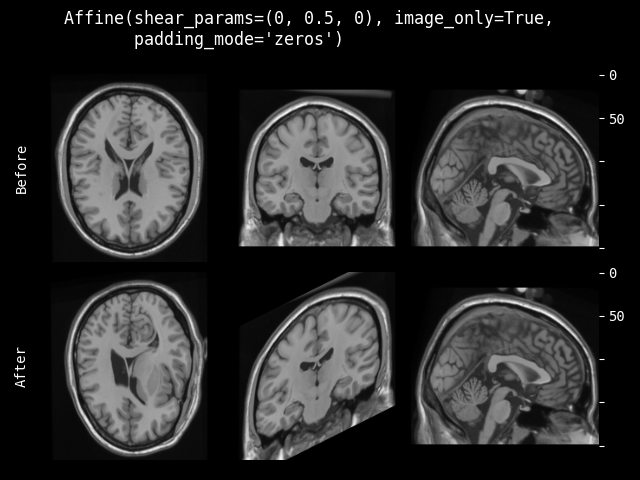class monai.transforms.Affine(rotate_params=None, shear_params=None, translate_params=None, scale_params=None, affine=None, spatial_size=None, mode=GridSampleMode.BILINEAR, padding_mode=GridSamplePadMode.REFLECTION, normalized=False, device=None, dtype=<class 'numpy.float32'>, align_corners=False, image_only=False, lazy=False)[source]#

Transform `img` given the affine parameters. A tutorial is available: Project-MONAI/tutorials.

This transform is capable of lazy execution. See the Lazy Resampling topic for more information.

Parameters:
• img – shape must be (num_channels, H, W[, D]),

• spatial_size – output image spatial size. if spatial_size and self.spatial_size are not defined, or smaller than 1, the transform will use the spatial size of img. if img has two spatial dimensions, spatial_size should have 2 elements [h, w]. if img has three spatial dimensions, spatial_size should have 3 elements [h, w, d].

• mode – {`"bilinear"`, `"nearest"`} or spline interpolation order 0-5 (integers). Interpolation mode to calculate output values. Defaults to `self.mode`. See also: https://pytorch.org/docs/stable/generated/torch.nn.functional.grid_sample.html When it’s an integer, the numpy (cpu tensor)/cupy (cuda tensor) backends will be used and the value represents the order of the spline interpolation. See also: https://docs.scipy.org/doc/scipy/reference/generated/scipy.ndimage.map_coordinates.html

• padding_mode – {`"zeros"`, `"border"`, `"reflection"`} Padding mode for outside grid values. Defaults to `self.padding_mode`. See also: https://pytorch.org/docs/stable/generated/torch.nn.functional.grid_sample.html When mode is an integer, using numpy/cupy backends, this argument accepts {‘reflect’, ‘grid-mirror’, ‘constant’, ‘grid-constant’, ‘nearest’, ‘mirror’, ‘grid-wrap’, ‘wrap’}. See also: https://docs.scipy.org/doc/scipy/reference/generated/scipy.ndimage.map_coordinates.html

• lazy – a flag to indicate whether this transform should execute lazily or not during this call. Setting this to False or True overrides the `lazy` flag set during initialization for this call. Defaults to None.

__init__(rotate_params=None, shear_params=None, translate_params=None, scale_params=None, affine=None, spatial_size=None, mode=GridSampleMode.BILINEAR, padding_mode=GridSamplePadMode.REFLECTION, normalized=False, device=None, dtype=<class 'numpy.float32'>, align_corners=False, image_only=False, lazy=False)[source]#

The affine transformations are applied in rotate, shear, translate, scale order.

Parameters:
• rotate_params – a rotation angle in radians, a scalar for 2D image, a tuple of 3 floats for 3D. Defaults to no rotation.

• shear_params

shearing factors for affine matrix, take a 3D affine as example:

```[
[1.0, params, params, 0.0],
[params, 1.0, params, 0.0],
[params, params, 1.0, 0.0],
[0.0, 0.0, 0.0, 1.0],
]

a tuple of 2 floats for 2D, a tuple of 6 floats for 3D. Defaults to no shearing.
```

• translate_params – a tuple of 2 floats for 2D, a tuple of 3 floats for 3D. Translation is in pixel/voxel relative to the center of the input image. Defaults to no translation.

• scale_params – scale factor for every spatial dims. a tuple of 2 floats for 2D, a tuple of 3 floats for 3D. Defaults to 1.0.

• affine – If applied, ignore the params (rotate_params, etc.) and use the supplied matrix. Should be square with each side = num of image spatial dimensions + 1.

• spatial_size – output image spatial size. if spatial_size and self.spatial_size are not defined, or smaller than 1, the transform will use the spatial size of img. if some components of the spatial_size are non-positive values, the transform will use the corresponding components of img size. For example, spatial_size=(32, -1) will be adapted to (32, 64) if the second spatial dimension size of img is 64.

• mode – {`"bilinear"`, `"nearest"`} or spline interpolation order 0-5 (integers). Interpolation mode to calculate output values. Defaults to `"bilinear"`. See also: https://pytorch.org/docs/stable/generated/torch.nn.functional.grid_sample.html When it’s an integer, the numpy (cpu tensor)/cupy (cuda tensor) backends will be used and the value represents the order of the spline interpolation. See also: https://docs.scipy.org/doc/scipy/reference/generated/scipy.ndimage.map_coordinates.html

• padding_mode – {`"zeros"`, `"border"`, `"reflection"`} Padding mode for outside grid values. Defaults to `"reflection"`. See also: https://pytorch.org/docs/stable/generated/torch.nn.functional.grid_sample.html When mode is an integer, using numpy/cupy backends, this argument accepts {‘reflect’, ‘grid-mirror’, ‘constant’, ‘grid-constant’, ‘nearest’, ‘mirror’, ‘grid-wrap’, ‘wrap’}. See also: https://docs.scipy.org/doc/scipy/reference/generated/scipy.ndimage.map_coordinates.html

• normalized – indicating whether the provided affine is defined to include a normalization transform converting the coordinates from [-(size-1)/2, (size-1)/2] (defined in `create_grid`) to [0, size - 1] or [-1, 1] in order to be compatible with the underlying resampling API. If normalized=False, additional coordinate normalization will be applied before resampling. See also: `monai.networks.utils.normalize_transform()`.

• device – device on which the tensor will be allocated.

• dtype – data type for resampling computation. Defaults to `float32`. If `None`, use the data type of input data. To be compatible with other modules, the output data type is always float32.

• image_only – if True return only the image volume, otherwise return (image, affine).

• lazy – a flag to indicate whether this transform should execute lazily or not. Defaults to False

inverse(data)[source]#

Inverse of `__call__`.

Raises:

NotImplementedError – When the subclass does not override this method.

Return type:

`Tensor`

property lazy#

Get whether lazy evaluation is enabled for this transform instance. :returns: True if the transform is operating in a lazy fashion, False if not.

#### Resample#

__call__(img, grid=None, mode=None, padding_mode=None, dtype=None, align_corners=None)[source]#
Parameters:

`monai.config.USE_COMPILED`

computes output image using values from img, locations from grid using pytorch. supports spatially 2D or 3D (num_channels, H, W[, D]).

Parameters:

#### RandAffine#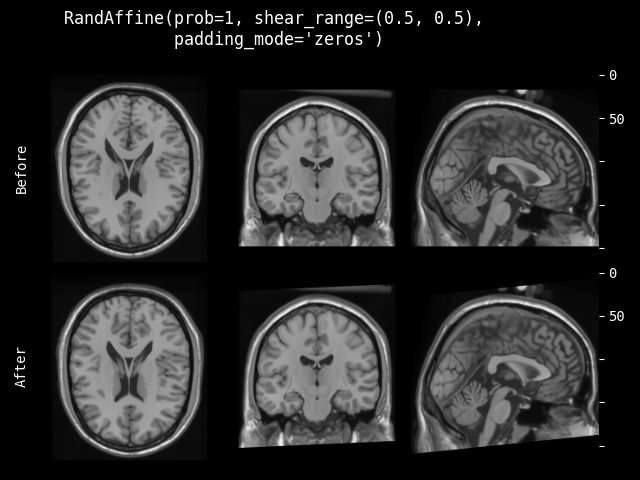class monai.transforms.RandAffine(prob=0.1, rotate_range=None, shear_range=None, translate_range=None, scale_range=None, spatial_size=None, mode=GridSampleMode.BILINEAR, padding_mode=GridSamplePadMode.REFLECTION, cache_grid=False, device=None, lazy=False)[source]#

Random affine transform. A tutorial is available: Project-MONAI/tutorials.

This transform is capable of lazy execution. See the Lazy Resampling topic for more information.

__call__(img, spatial_size=None, mode=None, padding_mode=None, randomize=True, grid=None, lazy=None)[source]#
Parameters:
• img – shape must be (num_channels, H, W[, D]),

• spatial_size – output image spatial size. if spatial_size and self.spatial_size are not defined, or smaller than 1, the transform will use the spatial size of img. if img has two spatial dimensions, spatial_size should have 2 elements [h, w]. if img has three spatial dimensions, spatial_size should have 3 elements [h, w, d].

• mode – {`"bilinear"`, `"nearest"`} or spline interpolation order 0-5 (integers). Interpolation mode to calculate output values. Defaults to `self.mode`. See also: https://pytorch.org/docs/stable/generated/torch.nn.functional.grid_sample.html When it’s an integer, the numpy (cpu tensor)/cupy (cuda tensor) backends will be used and the value represents the order of the spline interpolation. See also: https://docs.scipy.org/doc/scipy/reference/generated/scipy.ndimage.map_coordinates.html

• padding_mode – {`"zeros"`, `"border"`, `"reflection"`} Padding mode for outside grid values. Defaults to `self.padding_mode`. See also: https://pytorch.org/docs/stable/generated/torch.nn.functional.grid_sample.html When mode is an integer, using numpy/cupy backends, this argument accepts {‘reflect’, ‘grid-mirror’, ‘constant’, ‘grid-constant’, ‘nearest’, ‘mirror’, ‘grid-wrap’, ‘wrap’}. See also: https://docs.scipy.org/doc/scipy/reference/generated/scipy.ndimage.map_coordinates.html

• randomize – whether to execute randomize() function first, default to True.

• grid – precomputed grid to be used (mainly to accelerate RandAffined).

• lazy – a flag to indicate whether this transform should execute lazily or not during this call. Setting this to False or True overrides the `lazy` flag set during initialization for this call. Defaults to None.

Parameters:
• prob – probability of returning a randomized affine grid. defaults to 0.1, with 10% chance returns a randomized grid.

• rotate_range – angle range in radians. If element i is a pair of (min, max) values, then uniform[-rotate_range[i], rotate_range[i]) will be used to generate the rotation parameter for the i`th spatial dimension. If not, `uniform[-rotate_range[i], rotate_range[i]) will be used. This can be altered on a per-dimension basis. E.g., ((0,3), 1, …): for dim0, rotation will be in range [0, 3], and for dim1 [-1, 1] will be used. Setting a single value will use [-x, x] for dim0 and nothing for the remaining dimensions.

• shear_range

shear range with format matching rotate_range, it defines the range to randomly select shearing factors(a tuple of 2 floats for 2D, a tuple of 6 floats for 3D) for affine matrix, take a 3D affine as example:

```[
[1.0, params, params, 0.0],
[params, 1.0, params, 0.0],
[params, params, 1.0, 0.0],
[0.0, 0.0, 0.0, 1.0],
]
```

• translate_range – translate range with format matching rotate_range, it defines the range to randomly select pixel/voxel to translate for every spatial dims.

• scale_range – scaling range with format matching rotate_range. it defines the range to randomly select the scale factor to translate for every spatial dims. A value of 1.0 is added to the result. This allows 0 to correspond to no change (i.e., a scaling of 1.0).

• spatial_size – output image spatial size. if spatial_size and self.spatial_size are not defined, or smaller than 1, the transform will use the spatial size of img. if some components of the spatial_size are non-positive values, the transform will use the corresponding components of img size. For example, spatial_size=(32, -1) will be adapted to (32, 64) if the second spatial dimension size of img is 64.

• mode – {`"bilinear"`, `"nearest"`} or spline interpolation order 0-5 (integers). Interpolation mode to calculate output values. Defaults to `bilinear`. See also: https://pytorch.org/docs/stable/generated/torch.nn.functional.grid_sample.html When it’s an integer, the numpy (cpu tensor)/cupy (cuda tensor) backends will be used and the value represents the order of the spline interpolation. See also: https://docs.scipy.org/doc/scipy/reference/generated/scipy.ndimage.map_coordinates.html

• padding_mode – {`"zeros"`, `"border"`, `"reflection"`} Padding mode for outside grid values. Defaults to `reflection`. See also: https://pytorch.org/docs/stable/generated/torch.nn.functional.grid_sample.html When mode is an integer, using numpy/cupy backends, this argument accepts {‘reflect’, ‘grid-mirror’, ‘constant’, ‘grid-constant’, ‘nearest’, ‘mirror’, ‘grid-wrap’, ‘wrap’}. See also: https://docs.scipy.org/doc/scipy/reference/generated/scipy.ndimage.map_coordinates.html

• cache_grid – whether to cache the identity sampling grid. If the spatial size is not dynamically defined by input image, enabling this option could accelerate the transform.

• device – device on which the tensor will be allocated.

• lazy – a flag to indicate whether this transform should execute lazily or not. Defaults to False

get_identity_grid(spatial_size, lazy)[source]#

Return a cached or new identity grid depends on the availability.

Parameters:

spatial_size (`Sequence`[`int`]) – non-dynamic spatial size

inverse(data)[source]#

Inverse of `__call__`.

Raises:

NotImplementedError – When the subclass does not override this method.

Return type:

`Tensor`

property lazy#

Get whether lazy evaluation is enabled for this transform instance. :returns: True if the transform is operating in a lazy fashion, False if not.

randomize(data=None)[source]#

Within this method, `self.R` should be used, instead of np.random, to introduce random factors.

all `self.R` calls happen here so that we have a better chance to identify errors of sync the random state.

This method can generate the random factors based on properties of the input data.

set_random_state(seed=None, state=None)[source]#

Set the random state locally, to control the randomness, the derived classes should use `self.R` instead of np.random to introduce random factors.

Parameters:
• seed – set the random state with an integer seed.

• state – set the random state with a np.random.RandomState object.

Raises:

TypeError – When `state` is not an `Optional[np.random.RandomState]`.

Returns:

a Randomizable instance.

#### RandDeformGrid#

class monai.transforms.RandDeformGrid(spacing, magnitude_range, device=None)[source]#

Generate random deformation grid.

__call__(spatial_size)[source]#
Parameters:

spatial_size (`Sequence`[`int`]) – spatial size of the grid.

Return type:

`Tensor`

__init__(spacing, magnitude_range, device=None)[source]#
Parameters:
• spacing – spacing of the grid in 2D or 3D. e.g., spacing=(1, 1) indicates pixel-wise deformation in 2D, spacing=(1, 1, 1) indicates voxel-wise deformation in 3D, spacing=(2, 2) indicates deformation field defined on every other pixel in 2D.

• magnitude_range – the random offsets will be generated from uniform[magnitude, magnitude).

• device – device to store the output grid data.

randomize(grid_size)[source]#

Within this method, `self.R` should be used, instead of np.random, to introduce random factors.

all `self.R` calls happen here so that we have a better chance to identify errors of sync the random state.

This method can generate the random factors based on properties of the input data.

Raises:

NotImplementedError – When the subclass does not override this method.

Return type:

`None`

#### AffineGrid#

class monai.transforms.AffineGrid(rotate_params=None, shear_params=None, translate_params=None, scale_params=None, device=None, dtype=<class 'numpy.float32'>, align_corners=False, affine=None, lazy=False)[source]#

Affine transforms on the coordinates.

This transform is capable of lazy execution. See the Lazy Resampling topic for more information.

Parameters:
• rotate_params – a rotation angle in radians, a scalar for 2D image, a tuple of 3 floats for 3D. Defaults to no rotation.

• shear_params

shearing factors for affine matrix, take a 3D affine as example:

```[
[1.0, params, params, 0.0],
[params, 1.0, params, 0.0],
[params, params, 1.0, 0.0],
[0.0, 0.0, 0.0, 1.0],
]

a tuple of 2 floats for 2D, a tuple of 6 floats for 3D. Defaults to no shearing.
```

• translate_params – a tuple of 2 floats for 2D, a tuple of 3 floats for 3D. Translation is in pixel/voxel relative to the center of the input image. Defaults to no translation.

• scale_params – scale factor for every spatial dims. a tuple of 2 floats for 2D, a tuple of 3 floats for 3D. Defaults to 1.0.

• dtype – data type for the grid computation. Defaults to `float32`. If `None`, use the data type of input data (if grid is provided).

• device – device on which the tensor will be allocated, if a new grid is generated.

• affine – If applied, ignore the params (rotate_params, etc.) and use the supplied matrix. Should be square with each side = num of image spatial dimensions + 1.

• lazy – a flag to indicate whether this transform should execute lazily or not. Defaults to False

__call__(spatial_size=None, grid=None, lazy=None)[source]#

The grid can be initialized with a spatial_size parameter, or provided directly as grid. Therefore, either spatial_size or grid must be provided. When initialising from spatial_size, the backend “torch” will be used.

Parameters:
• spatial_size – output grid size.

• grid – grid to be transformed. Shape must be (3, H, W) for 2D or (4, H, W, D) for 3D.

• lazy – a flag to indicate whether this transform should execute lazily or not during this call. Setting this to False or True overrides the `lazy` flag set during initialization for this call. Defaults to None.

Raises:

ValueError – When `grid=None` and `spatial_size=None`. Incompatible values.

#### RandAffineGrid#

class monai.transforms.RandAffineGrid(rotate_range=None, shear_range=None, translate_range=None, scale_range=None, device=None, dtype=<class 'numpy.float32'>, lazy=False)[source]#

Generate randomised affine grid.

This transform is capable of lazy execution. See the Lazy Resampling topic for more information.

__call__(spatial_size=None, grid=None, randomize=True, lazy=None)[source]#
Parameters:
• spatial_size – output grid size.

• grid – grid to be transformed. Shape must be (3, H, W) for 2D or (4, H, W, D) for 3D.

• randomize – boolean as to whether the grid parameters governing the grid should be randomized.

• lazy – a flag to indicate whether this transform should execute lazily or not during this call. Setting this to False or True overrides the `lazy` flag set during initialization for this call. Defaults to None.

Returns:

a 2D (3xHxW) or 3D (4xHxWxD) grid.

__init__(rotate_range=None, shear_range=None, translate_range=None, scale_range=None, device=None, dtype=<class 'numpy.float32'>, lazy=False)[source]#
Parameters:
• rotate_range – angle range in radians. If element i is a pair of (min, max) values, then uniform[-rotate_range[i], rotate_range[i]) will be used to generate the rotation parameter for the i`th spatial dimension. If not, `uniform[-rotate_range[i], rotate_range[i]) will be used. This can be altered on a per-dimension basis. E.g., ((0,3), 1, …): for dim0, rotation will be in range [0, 3], and for dim1 [-1, 1] will be used. Setting a single value will use [-x, x] for dim0 and nothing for the remaining dimensions.

• shear_range

shear range with format matching rotate_range, it defines the range to randomly select shearing factors(a tuple of 2 floats for 2D, a tuple of 6 floats for 3D) for affine matrix, take a 3D affine as example:

```[
[1.0, params, params, 0.0],
[params, 1.0, params, 0.0],
[params, params, 1.0, 0.0],
[0.0, 0.0, 0.0, 1.0],
]
```

• translate_range – translate range with format matching rotate_range, it defines the range to randomly select voxels to translate for every spatial dims.

• scale_range – scaling range with format matching rotate_range. it defines the range to randomly select the scale factor to translate for every spatial dims. A value of 1.0 is added to the result. This allows 0 to correspond to no change (i.e., a scaling of 1.0).

• device – device to store the output grid data.

• dtype – data type for the grid computation. Defaults to `np.float32`. If `None`, use the data type of input data (if grid is provided).

• lazy – a flag to indicate whether this transform should execute lazily or not. Defaults to False

get_transformation_matrix()[source]#

Get the most recently applied transformation matrix

randomize(data=None)[source]#

Within this method, `self.R` should be used, instead of np.random, to introduce random factors.

all `self.R` calls happen here so that we have a better chance to identify errors of sync the random state.

This method can generate the random factors based on properties of the input data.

Raises:

NotImplementedError – When the subclass does not override this method.

#### GridDistortion#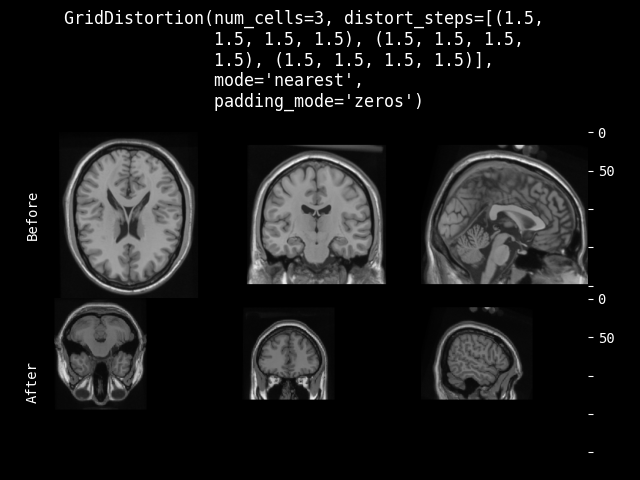Parameters:

Grid distortion transform. Refer to: albumentations-team/albumentations

Parameters:

#### RandGridDistortion#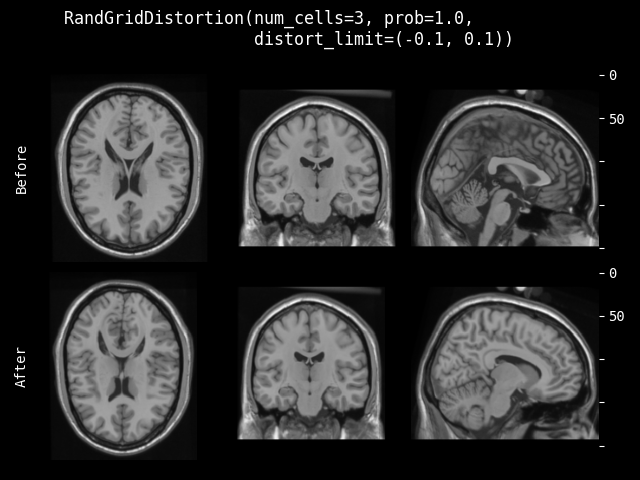Parameters:

Random grid distortion transform. Refer to: albumentations-team/albumentations

Parameters:
randomize(spatial_shape)[source]#

Within this method, `self.R` should be used, instead of np.random, to introduce random factors.

all `self.R` calls happen here so that we have a better chance to identify errors of sync the random state.

This method can generate the random factors based on properties of the input data.

Return type:

`None`

#### Rand2DElastic#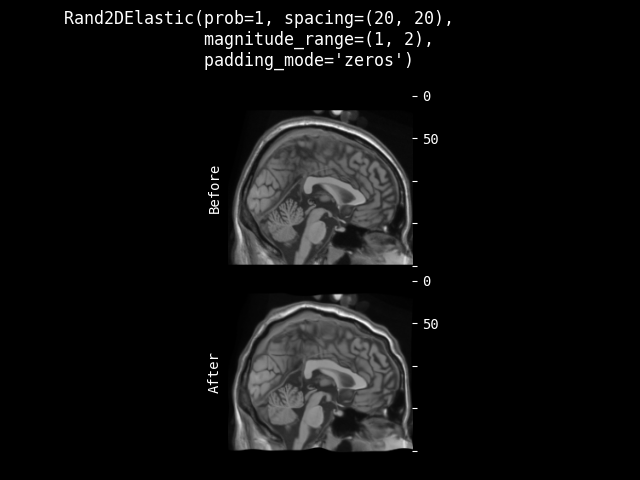class monai.transforms.Rand2DElastic(spacing, magnitude_range, prob=0.1, rotate_range=None, shear_range=None, translate_range=None, scale_range=None, spatial_size=None, mode=GridSampleMode.BILINEAR, padding_mode=GridSamplePadMode.REFLECTION, device=None)[source]#

Random elastic deformation and affine in 2D. A tutorial is available: Project-MONAI/tutorials.

Parameters:
Parameters:
• spacing – distance in between the control points.

• magnitude_range – the random offsets will be generated from `uniform[magnitude, magnitude)`.

• prob – probability of returning a randomized elastic transform. defaults to 0.1, with 10% chance returns a randomized elastic transform, otherwise returns a `spatial_size` centered area extracted from the input image.

• rotate_range – angle range in radians. If element i is a pair of (min, max) values, then uniform[-rotate_range[i], rotate_range[i]) will be used to generate the rotation parameter for the i`th spatial dimension. If not, `uniform[-rotate_range[i], rotate_range[i]) will be used. This can be altered on a per-dimension basis. E.g., ((0,3), 1, …): for dim0, rotation will be in range [0, 3], and for dim1 [-1, 1] will be used. Setting a single value will use [-x, x] for dim0 and nothing for the remaining dimensions.

• shear_range

shear range with format matching rotate_range, it defines the range to randomly select shearing factors(a tuple of 2 floats for 2D) for affine matrix, take a 2D affine as example:

```[
[1.0, params, 0.0],
[params, 1.0, 0.0],
[0.0, 0.0, 1.0],
]
```

• translate_range – translate range with format matching rotate_range, it defines the range to randomly select pixel to translate for every spatial dims.

• scale_range – scaling range with format matching rotate_range. it defines the range to randomly select the scale factor to translate for every spatial dims. A value of 1.0 is added to the result. This allows 0 to correspond to no change (i.e., a scaling of 1.0).

• spatial_size – specifying output image spatial size [h, w]. if spatial_size and self.spatial_size are not defined, or smaller than 1, the transform will use the spatial size of img. if some components of the spatial_size are non-positive values, the transform will use the corresponding components of img size. For example, spatial_size=(32, -1) will be adapted to (32, 64) if the second spatial dimension size of img is 64.

• mode – {`"bilinear"`, `"nearest"`} or spline interpolation order 0-5 (integers). Interpolation mode to calculate output values. Defaults to `"bilinear"`. See also: https://pytorch.org/docs/stable/generated/torch.nn.functional.grid_sample.html When it’s an integer, the numpy (cpu tensor)/cupy (cuda tensor) backends will be used and the value represents the order of the spline interpolation. See also: https://docs.scipy.org/doc/scipy/reference/generated/scipy.ndimage.map_coordinates.html

• padding_mode – {`"zeros"`, `"border"`, `"reflection"`} Padding mode for outside grid values. Defaults to `"reflection"`. See also: https://pytorch.org/docs/stable/generated/torch.nn.functional.grid_sample.html When mode is an integer, using numpy/cupy backends, this argument accepts {‘reflect’, ‘grid-mirror’, ‘constant’, ‘grid-constant’, ‘nearest’, ‘mirror’, ‘grid-wrap’, ‘wrap’}. See also: https://docs.scipy.org/doc/scipy/reference/generated/scipy.ndimage.map_coordinates.html

• device – device on which the tensor will be allocated.

randomize(spatial_size)[source]#

Within this method, `self.R` should be used, instead of np.random, to introduce random factors.

all `self.R` calls happen here so that we have a better chance to identify errors of sync the random state.

This method can generate the random factors based on properties of the input data.

Return type:

`None`

set_random_state(seed=None, state=None)[source]#

Set the random state locally, to control the randomness, the derived classes should use `self.R` instead of np.random to introduce random factors.

Parameters:
• seed – set the random state with an integer seed.

• state – set the random state with a np.random.RandomState object.

Raises:

TypeError – When `state` is not an `Optional[np.random.RandomState]`.

Returns:

a Randomizable instance.

#### Rand3DElastic#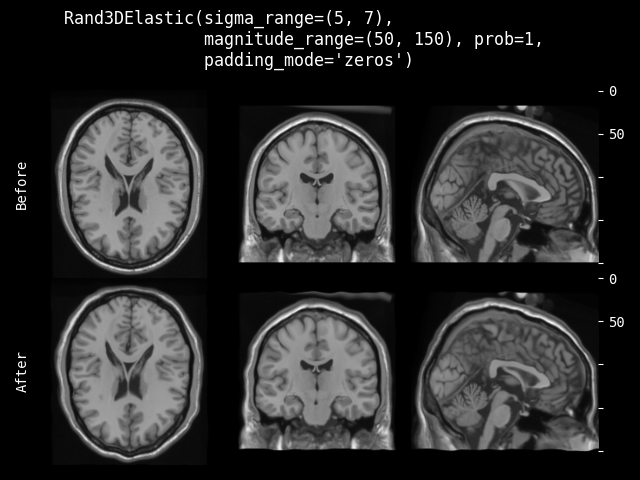class monai.transforms.Rand3DElastic(sigma_range, magnitude_range, prob=0.1, rotate_range=None, shear_range=None, translate_range=None, scale_range=None, spatial_size=None, mode=GridSampleMode.BILINEAR, padding_mode=GridSamplePadMode.REFLECTION, device=None)[source]#

Random elastic deformation and affine in 3D. A tutorial is available: Project-MONAI/tutorials.

Parameters:
Parameters:
• sigma_range – a Gaussian kernel with standard deviation sampled from `uniform[sigma_range, sigma_range)` will be used to smooth the random offset grid.

• magnitude_range – the random offsets on the grid will be generated from `uniform[magnitude, magnitude)`.

• prob – probability of returning a randomized elastic transform. defaults to 0.1, with 10% chance returns a randomized elastic transform, otherwise returns a `spatial_size` centered area extracted from the input image.

• rotate_range – angle range in radians. If element i is a pair of (min, max) values, then uniform[-rotate_range[i], rotate_range[i]) will be used to generate the rotation parameter for the i`th spatial dimension. If not, `uniform[-rotate_range[i], rotate_range[i]) will be used. This can be altered on a per-dimension basis. E.g., ((0,3), 1, …): for dim0, rotation will be in range [0, 3], and for dim1 [-1, 1] will be used. Setting a single value will use [-x, x] for dim0 and nothing for the remaining dimensions.

• shear_range

shear range with format matching rotate_range, it defines the range to randomly select shearing factors(a tuple of 6 floats for 3D) for affine matrix, take a 3D affine as example:

```[
[1.0, params, params, 0.0],
[params, 1.0, params, 0.0],
[params, params, 1.0, 0.0],
[0.0, 0.0, 0.0, 1.0],
]
```

• translate_range – translate range with format matching rotate_range, it defines the range to randomly select voxel to translate for every spatial dims.

• scale_range – scaling range with format matching rotate_range. it defines the range to randomly select the scale factor to translate for every spatial dims. A value of 1.0 is added to the result. This allows 0 to correspond to no change (i.e., a scaling of 1.0).

• spatial_size – specifying output image spatial size [h, w, d]. if spatial_size and self.spatial_size are not defined, or smaller than 1, the transform will use the spatial size of img. if some components of the spatial_size are non-positive values, the transform will use the corresponding components of img size. For example, spatial_size=(32, 32, -1) will be adapted to (32, 32, 64) if the third spatial dimension size of img is 64.

• mode – {`"bilinear"`, `"nearest"`} or spline interpolation order 0-5 (integers). Interpolation mode to calculate output values. Defaults to `"bilinear"`. See also: https://pytorch.org/docs/stable/generated/torch.nn.functional.grid_sample.html When it’s an integer, the numpy (cpu tensor)/cupy (cuda tensor) backends will be used and the value represents the order of the spline interpolation. See also: https://docs.scipy.org/doc/scipy/reference/generated/scipy.ndimage.map_coordinates.html

• padding_mode – {`"zeros"`, `"border"`, `"reflection"`} Padding mode for outside grid values. Defaults to `"reflection"`. See also: https://pytorch.org/docs/stable/generated/torch.nn.functional.grid_sample.html When mode is an integer, using numpy/cupy backends, this argument accepts {‘reflect’, ‘grid-mirror’, ‘constant’, ‘grid-constant’, ‘nearest’, ‘mirror’, ‘grid-wrap’, ‘wrap’}. See also: https://docs.scipy.org/doc/scipy/reference/generated/scipy.ndimage.map_coordinates.html

• device – device on which the tensor will be allocated.

randomize(grid_size)[source]#

Within this method, `self.R` should be used, instead of np.random, to introduce random factors.

all `self.R` calls happen here so that we have a better chance to identify errors of sync the random state.

This method can generate the random factors based on properties of the input data.

Return type:

`None`

set_random_state(seed=None, state=None)[source]#

Set the random state locally, to control the randomness, the derived classes should use `self.R` instead of np.random to introduce random factors.

Parameters:
• seed – set the random state with an integer seed.

• state – set the random state with a np.random.RandomState object.

Raises:

TypeError – When `state` is not an `Optional[np.random.RandomState]`.

Returns:

a Randomizable instance.

#### Rotate90#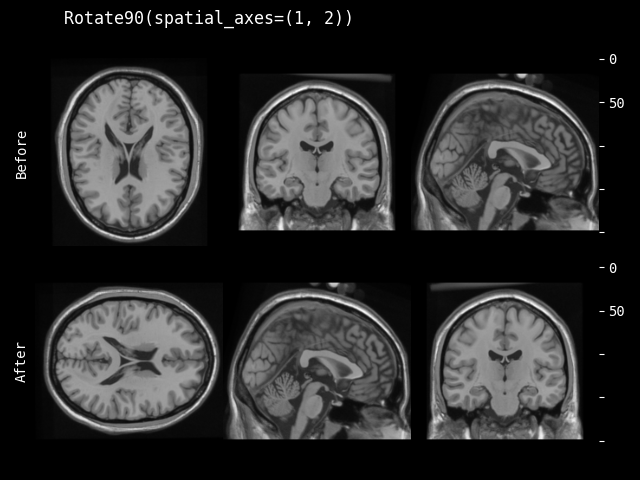class monai.transforms.Rotate90(k=1, spatial_axes=(0, 1), lazy=False)[source]#

Rotate an array by 90 degrees in the plane specified by axes. See torch.rot90 for additional details: https://pytorch.org/docs/stable/generated/torch.rot90.html#torch-rot90.

This transform is capable of lazy execution. See the Lazy Resampling topic for more information.

__call__(img, lazy=None)[source]#
Parameters:
• img – channel first array, must have shape: (num_channels, H[, W, …, ]),

• lazy – a flag to indicate whether this transform should execute lazily or not during this call. Setting this to False or True overrides the `lazy` flag set during initialization for this call. Defaults to None.

__init__(k=1, spatial_axes=(0, 1), lazy=False)[source]#
Parameters:
• k (`int`) – number of times to rotate by 90 degrees.

• spatial_axes (`tuple`[`int`, `int`]) – 2 int numbers, defines the plane to rotate with 2 spatial axes. Default: (0, 1), this is the first two axis in spatial dimensions. If axis is negative it counts from the last to the first axis.

• lazy (`bool`) – a flag to indicate whether this transform should execute lazily or not. Defaults to False

inverse(data)[source]#

Inverse of `__call__`.

Raises:

NotImplementedError – When the subclass does not override this method.

Return type:

`Tensor`

#### RandRotate90#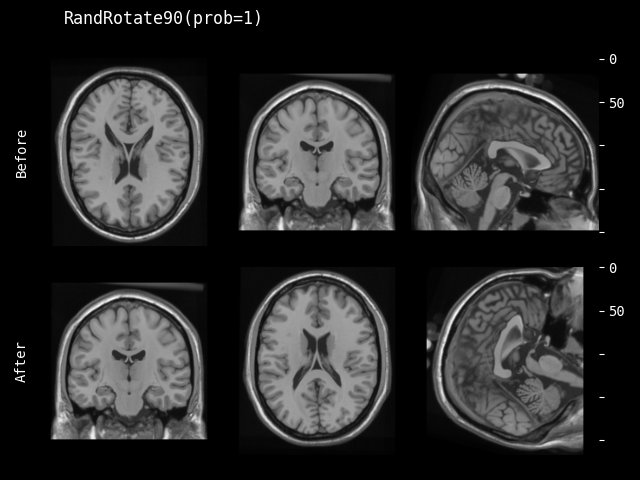class monai.transforms.RandRotate90(prob=0.1, max_k=3, spatial_axes=(0, 1), lazy=False)[source]#

With probability prob, input arrays are rotated by 90 degrees in the plane specified by spatial_axes.

This transform is capable of lazy execution. See the Lazy Resampling topic for more information.

__call__(img, randomize=True, lazy=None)[source]#
Parameters:
• img – channel first array, must have shape: (num_channels, H[, W, …, ]),

• randomize – whether to execute randomize() function first, default to True.

• lazy – a flag to indicate whether this transform should execute lazily or not during this call. Setting this to False or True overrides the `lazy` flag set during initialization for this call. Defaults to None.

__init__(prob=0.1, max_k=3, spatial_axes=(0, 1), lazy=False)[source]#
Parameters:
• prob (`float`) – probability of rotating. (Default 0.1, with 10% probability it returns a rotated array)

• max_k (`int`) – number of rotations will be sampled from np.random.randint(max_k) + 1, (Default 3).

• spatial_axes (`tuple`[`int`, `int`]) – 2 int numbers, defines the plane to rotate with 2 spatial axes. Default: (0, 1), this is the first two axis in spatial dimensions.

• lazy (`bool`) – a flag to indicate whether this transform should execute lazily or not. Defaults to False

inverse(data)[source]#

Inverse of `__call__`.

Raises:

NotImplementedError – When the subclass does not override this method.

Return type:

`Tensor`

randomize(data=None)[source]#

Within this method, `self.R` should be used, instead of np.random, to introduce random factors.

all `self.R` calls happen here so that we have a better chance to identify errors of sync the random state.

This method can generate the random factors based on properties of the input data.

#### Flip#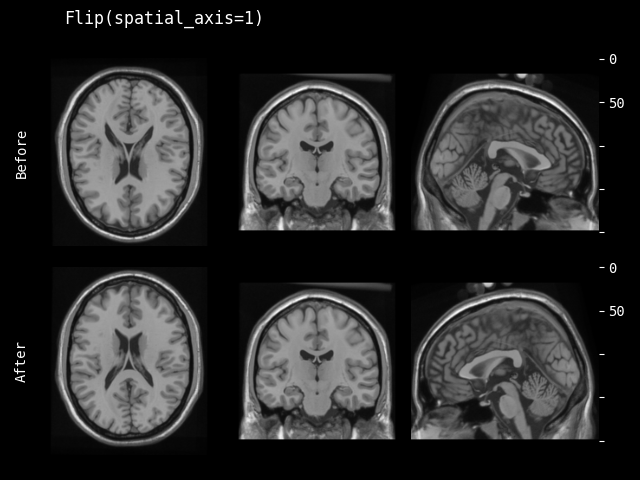class monai.transforms.Flip(spatial_axis=None, lazy=False)[source]#

Reverses the order of elements along the given spatial axis. Preserves shape. See torch.flip documentation for additional details: https://pytorch.org/docs/stable/generated/torch.flip.html

This transform is capable of lazy execution. See the Lazy Resampling topic for more information.

Parameters:
• spatial_axis – spatial axes along which to flip over. Default is None. The default axis=None will flip over all of the axes of the input array. If axis is negative it counts from the last to the first axis. If axis is a tuple of ints, flipping is performed on all of the axes specified in the tuple.

• lazy – a flag to indicate whether this transform should execute lazily or not. Defaults to False

__call__(img, lazy=None)[source]#
Parameters:
• img – channel first array, must have shape: (num_channels, H[, W, …, ])

• lazy – a flag to indicate whether this transform should execute lazily or not during this call. Setting this to False or True overrides the `lazy` flag set during initialization for this call. Defaults to None.

inverse(data)[source]#

Inverse of `__call__`.

Raises:

NotImplementedError – When the subclass does not override this method.

Return type:

`Tensor`

#### Resize#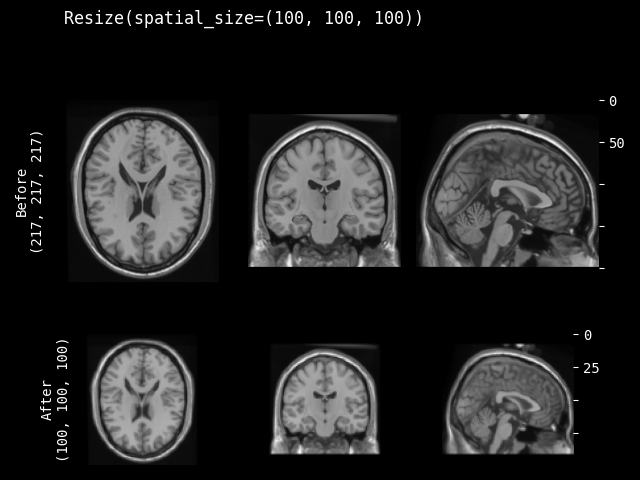class monai.transforms.Resize(spatial_size, size_mode='all', mode=InterpolateMode.AREA, align_corners=None, anti_aliasing=False, anti_aliasing_sigma=None, dtype=torch.float32, lazy=False)[source]#

Resize the input image to given spatial size (with scaling, not cropping/padding). Implemented using `torch.nn.functional.interpolate`.

This transform is capable of lazy execution. See the Lazy Resampling topic for more information.

Parameters:
• spatial_size – expected shape of spatial dimensions after resize operation. if some components of the spatial_size are non-positive values, the transform will use the corresponding components of img size. For example, spatial_size=(32, -1) will be adapted to (32, 64) if the second spatial dimension size of img is 64.

• size_mode – should be “all” or “longest”, if “all”, will use spatial_size for all the spatial dims, if “longest”, rescale the image so that only the longest side is equal to specified spatial_size, which must be an int number in this case, keeping the aspect ratio of the initial image, refer to: https://albumentations.ai/docs/api_reference/augmentations/geometric/resize/ #albumentations.augmentations.geometric.resize.LongestMaxSize.

• mode – {`"nearest"`, `"nearest-exact"`, `"linear"`, `"bilinear"`, `"bicubic"`, `"trilinear"`, `"area"`} The interpolation mode. Defaults to `"area"`. See also: https://pytorch.org/docs/stable/generated/torch.nn.functional.interpolate.html

• align_corners – This only has an effect when mode is ‘linear’, ‘bilinear’, ‘bicubic’ or ‘trilinear’. Default: None. See also: https://pytorch.org/docs/stable/generated/torch.nn.functional.interpolate.html

• anti_aliasing – bool Whether to apply a Gaussian filter to smooth the image prior to downsampling. It is crucial to filter when downsampling the image to avoid aliasing artifacts. See also `skimage.transform.resize`

• anti_aliasing_sigma – {float, tuple of floats}, optional Standard deviation for Gaussian filtering used when anti-aliasing. By default, this value is chosen as (s - 1) / 2 where s is the downsampling factor, where s > 1. For the up-size case, s < 1, no anti-aliasing is performed prior to rescaling.

• dtype – data type for resampling computation. Defaults to `float32`. If None, use the data type of input data.

• lazy – a flag to indicate whether this transform should execute lazily or not. Defaults to False

__call__(img, mode=None, align_corners=None, anti_aliasing=None, anti_aliasing_sigma=None, dtype=None, lazy=None)[source]#
Parameters:
• img – channel first array, must have shape: (num_channels, H[, W, …, ]).

• mode – {`"nearest"`, `"nearest-exact"`, `"linear"`, `"bilinear"`, `"bicubic"`, `"trilinear"`, `"area"`} The interpolation mode. Defaults to `self.mode`. See also: https://pytorch.org/docs/stable/generated/torch.nn.functional.interpolate.html

• align_corners – This only has an effect when mode is ‘linear’, ‘bilinear’, ‘bicubic’ or ‘trilinear’. Defaults to `self.align_corners`. See also: https://pytorch.org/docs/stable/generated/torch.nn.functional.interpolate.html

• anti_aliasing – bool, optional Whether to apply a Gaussian filter to smooth the image prior to downsampling. It is crucial to filter when downsampling the image to avoid aliasing artifacts. See also `skimage.transform.resize`

• anti_aliasing_sigma – {float, tuple of floats}, optional Standard deviation for Gaussian filtering used when anti-aliasing. By default, this value is chosen as (s - 1) / 2 where s is the downsampling factor, where s > 1. For the up-size case, s < 1, no anti-aliasing is performed prior to rescaling.

• dtype – data type for resampling computation. Defaults to `self.dtype`. If None, use the data type of input data.

• lazy – a flag to indicate whether this transform should execute lazily or not during this call. Setting this to False or True overrides the `lazy` flag set during initialization for this call. Defaults to None.

Raises:

ValueError – When `self.spatial_size` length is less than `img` spatial dimensions.

inverse(data)[source]#

Inverse of `__call__`.

Raises:

NotImplementedError – When the subclass does not override this method.

Return type:

`Tensor`

#### Rotate#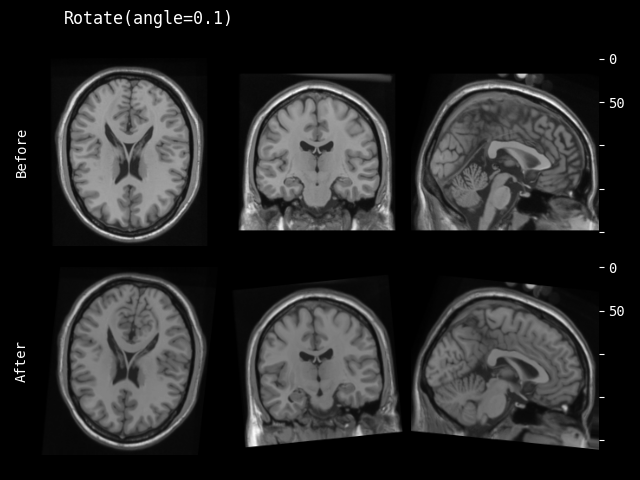Rotates an input image by given angle using `monai.networks.layers.AffineTransform`.

This transform is capable of lazy execution. See the Lazy Resampling topic for more information.

Parameters:
• angle – Rotation angle(s) in radians. should a float for 2D, three floats for 3D.

• keep_size – If it is True, the output shape is kept the same as the input. If it is False, the output shape is adapted so that the input array is contained completely in the output. Default is True.

• mode – {`"bilinear"`, `"nearest"`} Interpolation mode to calculate output values. Defaults to `"bilinear"`. See also: https://pytorch.org/docs/stable/generated/torch.nn.functional.grid_sample.html

• padding_mode – {`"zeros"`, `"border"`, `"reflection"`} Padding mode for outside grid values. Defaults to `"border"`. See also: https://pytorch.org/docs/stable/generated/torch.nn.functional.grid_sample.html

• dtype – data type for resampling computation. Defaults to `float32`. If None, use the data type of input data. To be compatible with other modules, the output data type is always `float32`.

• lazy – a flag to indicate whether this transform should execute lazily or not. Defaults to False

__call__(img, mode=None, padding_mode=None, align_corners=None, dtype=None, lazy=None)[source]#
Parameters:
Raises:

ValueError – When `img` spatially is not one of [2D, 3D].

inverse(data)[source]#

Inverse of `__call__`.

Raises:

NotImplementedError – When the subclass does not override this method.

Return type:

`Tensor`

#### Zoom#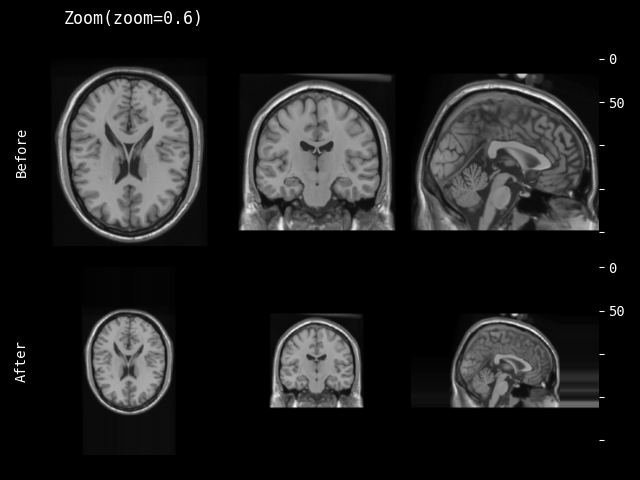Zooms an ND image using `torch.nn.functional.interpolate`. For details, please see https://pytorch.org/docs/stable/generated/torch.nn.functional.interpolate.html.

Different from `monai.transforms.resize`, this transform takes scaling factors as input, and provides an option of preserving the input spatial size.

This transform is capable of lazy execution. See the Lazy Resampling topic for more information.

Parameters:
• zoom – The zoom factor along the spatial axes. If a float, zoom is the same for each spatial axis. If a sequence, zoom should contain one value for each spatial axis.

• mode – {`"nearest"`, `"nearest-exact"`, `"linear"`, `"bilinear"`, `"bicubic"`, `"trilinear"`, `"area"`} The interpolation mode. Defaults to `"area"`. See also: https://pytorch.org/docs/stable/generated/torch.nn.functional.interpolate.html

• padding_mode – available modes for numpy array:{`"constant"`, `"edge"`, `"linear_ramp"`, `"maximum"`, `"mean"`, `"median"`, `"minimum"`, `"reflect"`, `"symmetric"`, `"wrap"`, `"empty"`} available modes for PyTorch Tensor: {`"constant"`, `"reflect"`, `"replicate"`, `"circular"`}. One of the listed string values or a user supplied function. Defaults to `"edge"`. The mode to pad data after zooming. See also: https://numpy.org/doc/1.18/reference/generated/numpy.pad.html https://pytorch.org/docs/stable/generated/torch.nn.functional.pad.html

• align_corners – This only has an effect when mode is ‘linear’, ‘bilinear’, ‘bicubic’ or ‘trilinear’. Default: None. See also: https://pytorch.org/docs/stable/generated/torch.nn.functional.interpolate.html

• dtype – data type for resampling computation. Defaults to `float32`. If None, use the data type of input data.

• keep_size – Should keep original size (padding/slicing if needed), default is True.

• lazy – a flag to indicate whether this transform should execute lazily or not. Defaults to False

• kwargs – other arguments for the np.pad or torch.pad function. note that np.pad treats channel dimension as the first dimension.

__call__(img, mode=None, padding_mode=None, align_corners=None, dtype=None, lazy=None)[source]#
Parameters:
• img – channel first array, must have shape: (num_channels, H[, W, …, ]).

• mode – {`"nearest"`, `"nearest-exact"`, `"linear"`, `"bilinear"`, `"bicubic"`, `"trilinear"`, `"area"`} The interpolation mode. Defaults to `self.mode`. See also: https://pytorch.org/docs/stable/generated/torch.nn.functional.interpolate.html

• padding_mode – available modes for numpy array:{`"constant"`, `"edge"`, `"linear_ramp"`, `"maximum"`, `"mean"`, `"median"`, `"minimum"`, `"reflect"`, `"symmetric"`, `"wrap"`, `"empty"`} available modes for PyTorch Tensor: {`"constant"`, `"reflect"`, `"replicate"`, `"circular"`}. One of the listed string values or a user supplied function. Defaults to `"edge"`. The mode to pad data after zooming. See also: https://numpy.org/doc/1.18/reference/generated/numpy.pad.html https://pytorch.org/docs/stable/generated/torch.nn.functional.pad.html

• align_corners – This only has an effect when mode is ‘linear’, ‘bilinear’, ‘bicubic’ or ‘trilinear’. Defaults to `self.align_corners`. See also: https://pytorch.org/docs/stable/generated/torch.nn.functional.interpolate.html

• dtype – data type for resampling computation. Defaults to `self.dtype`. If None, use the data type of input data.

• lazy – a flag to indicate whether this transform should execute lazily or not during this call. Setting this to False or True overrides the `lazy` flag set during initialization for this call. Defaults to None.

inverse(data)[source]#

Inverse of `__call__`.

Raises:

NotImplementedError – When the subclass does not override this method.

Return type:

`Tensor`

#### GridPatch#

Extract all the patches sweeping the entire image in a row-major sliding-window manner with possible overlaps. It can sort the patches and return all or a subset of them.

Parameters:
• patch_size – size of patches to generate slices for, 0 or None selects whole dimension

• offset – offset of starting position in the array, default is 0 for each dimension.

• num_patches – number of patches (or maximum number of patches) to return. If the requested number of patches is greater than the number of available patches, padding will be applied to provide exactly num_patches patches unless threshold is set. When threshold is set, this value is treated as the maximum number of patches. Defaults to None, which does not limit number of the patches.

• overlap – the amount of overlap of neighboring patches in each dimension (a value between 0.0 and 1.0). If only one float number is given, it will be applied to all dimensions. Defaults to 0.0.

• sort_fn – when num_patches is provided, it determines if keep patches with highest values (“max”), lowest values (“min”), or in their default order (None). Default to None.

• threshold – a value to keep only the patches whose sum of intensities are less than the threshold. Defaults to no filtering.

• pad_mode – the mode for padding the input image by patch_size to include patches that cross boundaries. Available modes: (Numpy) {`"constant"`, `"edge"`, `"linear_ramp"`, `"maximum"`, `"mean"`, `"median"`, `"minimum"`, `"reflect"`, `"symmetric"`, `"wrap"`, `"empty"`} (PyTorch) {`"constant"`, `"reflect"`, `"replicate"`, `"circular"`}. One of the listed string values or a user supplied function. Defaults to None, which means no padding will be applied. See also: https://numpy.org/doc/stable/reference/generated/numpy.pad.html https://pytorch.org/docs/stable/generated/torch.nn.functional.pad.html requires pytorch >= 1.10 for best compatibility.

Returns:

the extracted patches as a single tensor (with patch dimension as the first dimension),

• PatchKeys.LOCATION: the starting location of the patch in the image,

• PatchKeys.COUNT: total number of patches in the image,

• ”spatial_shape”: spatial size of the extracted patch, and

• ”offset”: the amount of offset for the patches in the image (starting position of the first patch)

Return type:

MetaTensor

__call__(array)[source]#

Extract the patches (sweeping the entire image in a row-major sliding-window manner with possible overlaps).

Parameters:

array (`Union`[`ndarray`, `Tensor`]) – a input image as numpy.ndarray or torch.Tensor

Returns:

the extracted patches as a single tensor (with patch dimension as the first dimension),

with defined PatchKeys.LOCATION and PatchKeys.COUNT metadata.

Return type:

MetaTensor

filter_count(image_np, locations)[source]#

Sort the patches based on the sum of their intensity, and just keep self.num_patches of them.

Parameters:
• image_np (`Union`[`ndarray`, `Tensor`]) – a numpy.ndarray or torch.Tensor representing a stack of patches.

• locations (`ndarray`) – a numpy.ndarray representing the stack of location of each patch.

Return type:

`tuple`[`Union`[`ndarray`, `Tensor`], `ndarray`]

filter_threshold(image_np, locations)[source]#

Filter the patches and their locations according to a threshold.

Parameters:
• image_np (`Union`[`ndarray`, `Tensor`]) – a numpy.ndarray or torch.Tensor representing a stack of patches.

• locations (`ndarray`) – a numpy.ndarray representing the stack of location of each patch.

Returns:

tuple of filtered patches and locations.

Return type:

tuple[NdarrayOrTensor, numpy.ndarray]

#### RandGridPatch#

Extract all the patches sweeping the entire image in a row-major sliding-window manner with possible overlaps, and with random offset for the minimal corner of the image, (0,0) for 2D and (0,0,0) for 3D. It can sort the patches and return all or a subset of them.

Parameters:
• patch_size – size of patches to generate slices for, 0 or None selects whole dimension

• min_offset – the minimum range of offset to be selected randomly. Defaults to 0.

• max_offset – the maximum range of offset to be selected randomly. Defaults to image size modulo patch size.

• num_patches – number of patches (or maximum number of patches) to return. If the requested number of patches is greater than the number of available patches, padding will be applied to provide exactly num_patches patches unless threshold is set. When threshold is set, this value is treated as the maximum number of patches. Defaults to None, which does not limit number of the patches.

• overlap – the amount of overlap of neighboring patches in each dimension (a value between 0.0 and 1.0). If only one float number is given, it will be applied to all dimensions. Defaults to 0.0.

• sort_fn – when num_patches is provided, it determines if keep patches with highest values (“max”), lowest values (“min”), in random (“random”), or in their default order (None). Default to None.

• threshold – a value to keep only the patches whose sum of intensities are less than the threshold. Defaults to no filtering.

• pad_mode – the mode for padding the input image by patch_size to include patches that cross boundaries. Available modes: (Numpy) {`"constant"`, `"edge"`, `"linear_ramp"`, `"maximum"`, `"mean"`, `"median"`, `"minimum"`, `"reflect"`, `"symmetric"`, `"wrap"`, `"empty"`} (PyTorch) {`"constant"`, `"reflect"`, `"replicate"`, `"circular"`}. One of the listed string values or a user supplied function. Defaults to None, which means no padding will be applied. See also: https://numpy.org/doc/stable/reference/generated/numpy.pad.html https://pytorch.org/docs/stable/generated/torch.nn.functional.pad.html requires pytorch >= 1.10 for best compatibility.

Returns:

the extracted patches as a single tensor (with patch dimension as the first dimension),

• PatchKeys.LOCATION: the starting location of the patch in the image,

• PatchKeys.COUNT: total number of patches in the image,

• ”spatial_shape”: spatial size of the extracted patch, and

• ”offset”: the amount of offset for the patches in the image (starting position of the first patch)

Return type:

MetaTensor

__call__(array, randomize=True)[source]#

Extract the patches (sweeping the entire image in a row-major sliding-window manner with possible overlaps).

Parameters:

array (`Union`[`ndarray`, `Tensor`]) – a input image as numpy.ndarray or torch.Tensor

Returns:

the extracted patches as a single tensor (with patch dimension as the first dimension),

with defined PatchKeys.LOCATION and PatchKeys.COUNT metadata.

Return type:

MetaTensor

filter_count(image_np, locations)[source]#

Sort the patches based on the sum of their intensity, and just keep self.num_patches of them.

Parameters:
• image_np (`Union`[`ndarray`, `Tensor`]) – a numpy.ndarray or torch.Tensor representing a stack of patches.

• locations (`ndarray`) – a numpy.ndarray representing the stack of location of each patch.

Return type:

`tuple`[`Union`[`ndarray`, `Tensor`], `ndarray`]

randomize(array)[source]#

Within this method, `self.R` should be used, instead of np.random, to introduce random factors.

all `self.R` calls happen here so that we have a better chance to identify errors of sync the random state.

This method can generate the random factors based on properties of the input data.

#### GridSplit#

class monai.transforms.GridSplit(grid=(2, 2), size=None)[source]#

Split the image into patches based on the provided grid in 2D.

Parameters:
• grid – a tuple define the shape of the grid upon which the image is split. Defaults to (2, 2)

• size – a tuple or an integer that defines the output patch sizes. If it’s an integer, the value will be repeated for each dimension. The default is None, where the patch size will be inferred from the grid shape.

Example

Given an image (torch.Tensor or numpy.ndarray) with size of (3, 10, 10) and a grid of (2, 2), it will return a Tensor or array with the size of (4, 3, 5, 5). Here, if the size is provided, the returned shape will be (4, 3, size, size)

Note: This transform currently support only image with two spatial dimensions.

__call__(image, size=None)[source]#

`data` is an element which often comes from an iteration over an iterable, such as `torch.utils.data.Dataset`. This method should return an updated version of `data`. To simplify the input validations, most of the transforms assume that

• `data` is a Numpy ndarray, PyTorch Tensor or string,

• the data shape can be:

1. string data without shape, LoadImage transform expects file paths,

2. most of the pre-/post-processing transforms expect: `(num_channels, spatial_dim_1[, spatial_dim_2, ...])`, except for example: AddChannel expects (spatial_dim_1[, spatial_dim_2, …])

• the channel dimension is often not omitted even if number of channels is one.

This method can optionally take additional arguments to help execute transformation operation.

Raises:

NotImplementedError – When the subclass does not override this method.

#### RandSimulateLowResolution#

class monai.transforms.RandSimulateLowResolution(prob=0.1, downsample_mode=InterpolateMode.NEAREST, upsample_mode=InterpolateMode.TRILINEAR, zoom_range=(0.5, 1.0), align_corners=False, device=None)[source]#

Random simulation of low resolution corresponding to nnU-Net’s SimulateLowResolutionTransform (MIC-DKFZ/batchgenerators) First, the array/tensor is resampled at lower resolution as determined by the zoom_factor which is uniformly sampled from the zoom_range. Then, the array/tensor is resampled at the original resolution.

__call__(img, randomize=True)[source]#
Parameters:
• img (`Tensor`) – shape must be (num_channels, H, W[, D]),

• randomize (`bool`) – whether to execute randomize() function first, defaults to True.

Return type:

`Tensor`

__init__(prob=0.1, downsample_mode=InterpolateMode.NEAREST, upsample_mode=InterpolateMode.TRILINEAR, zoom_range=(0.5, 1.0), align_corners=False, device=None)[source]#
Parameters:
• prob – probability of performing this augmentation

• downsample_mode – interpolation mode for downsampling operation

• upsample_mode – interpolation mode for upsampling operation

• zoom_range – range from which the random zoom factor for the downsampling and upsampling operation is

• tensor. (sampled. It determines the shape of the downsampled) –

• align_corners – This only has an effect when downsample_mode or upsample_mode is ‘linear’, ‘bilinear’, ‘bicubic’ or ‘trilinear’. Default: False See also: https://pytorch.org/docs/stable/generated/torch.nn.functional.interpolate.html

• device – device on which the tensor will be allocated.

randomize(data=None)[source]#

Within this method, `self.R` should be used, instead of np.random, to introduce random factors.

all `self.R` calls happen here so that we have a better chance to identify errors of sync the random state.

This method can generate the random factors based on properties of the input data.

### Smooth Field#